# ML Aggarwal Solutions for Class 10 Maths Chapter 9 Arithmetic and Geometric Progression

ML Aggarwal Solutions for Class 10 Maths Chapter 9 Arithmetic and Geometric Progression are provided here. BYJU’S tutor team has prepared solutions, which help students attain good marks in Maths. From the exam point of view, the solutions are solved in a simpler manner where students can secure an excellent score by solving the ML Aggarwal textbook. Solutions that are provided here will help you in getting acquainted with a wide variety of questions and thus, develop problem-solving skills. Download pdf of ML Aggarwal Solutions for Class 10 Maths Chapter 9 in their respective links

Chapter 9 – Arithmetic and Geometric Progression. Arithmetic progression is a sequence of numbers such that the difference between the consecutive terms is constant. Difference here means the second minus the first. Geometric progression is defined as a sequence of numbers, where each term after the first is found by multiplying the previous one by a fixed, non-zero number called the common ratio. In these ML Aggarwal Solutions for Class 10 Maths Chapter 9, students are going to learn solving different types of problems and topics of Arithmetic and Geometric Progression.

## ML Aggarwal Solutions for Class 10 Maths Chapter 9 :-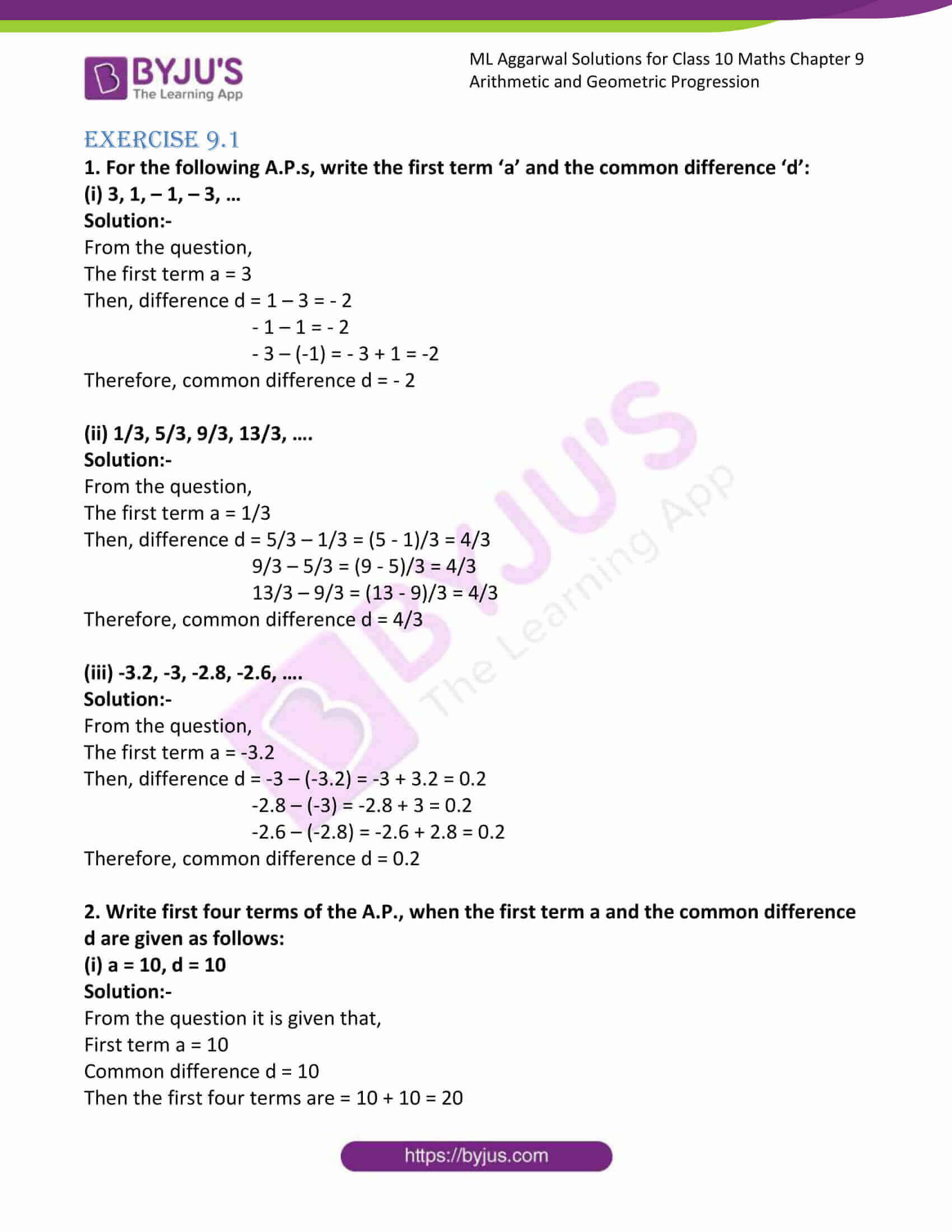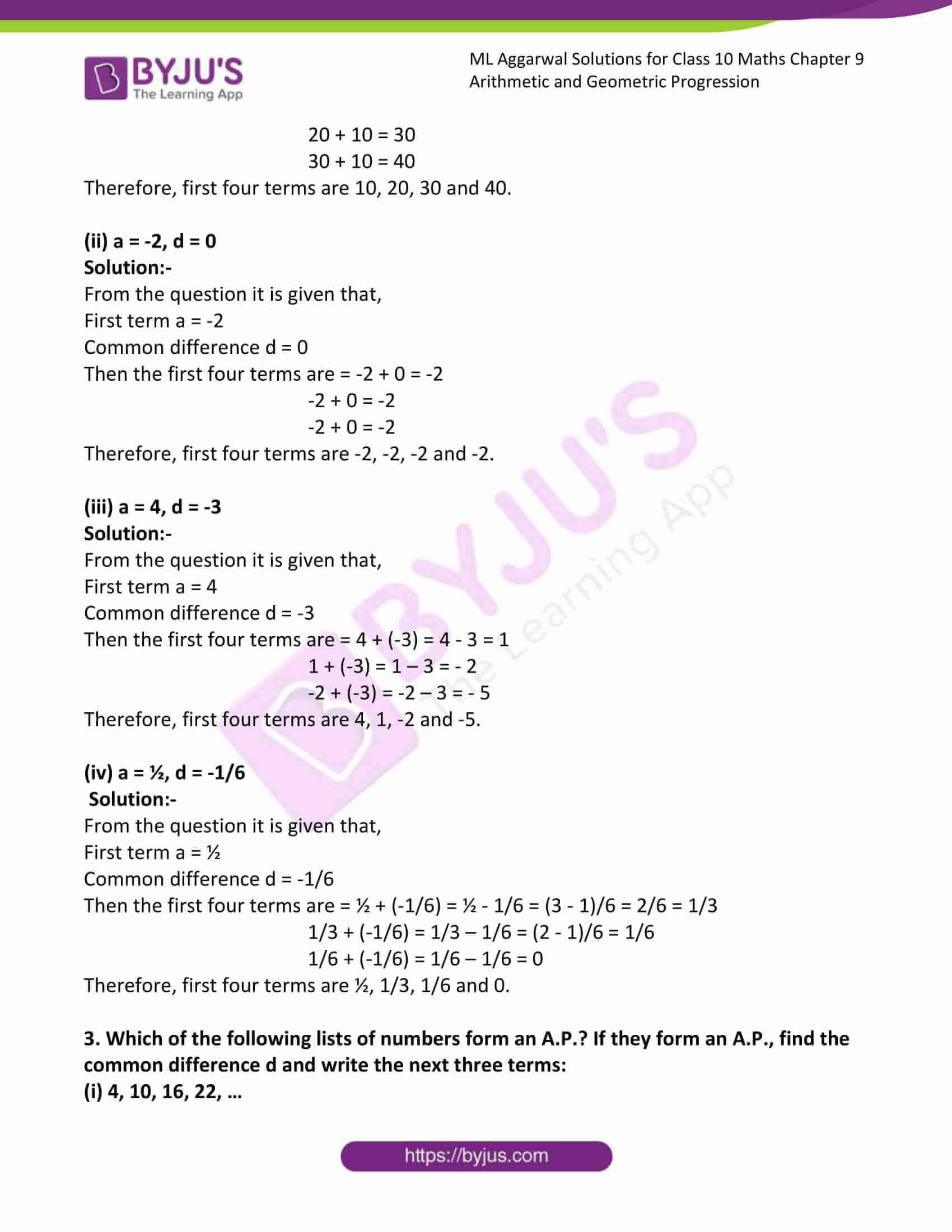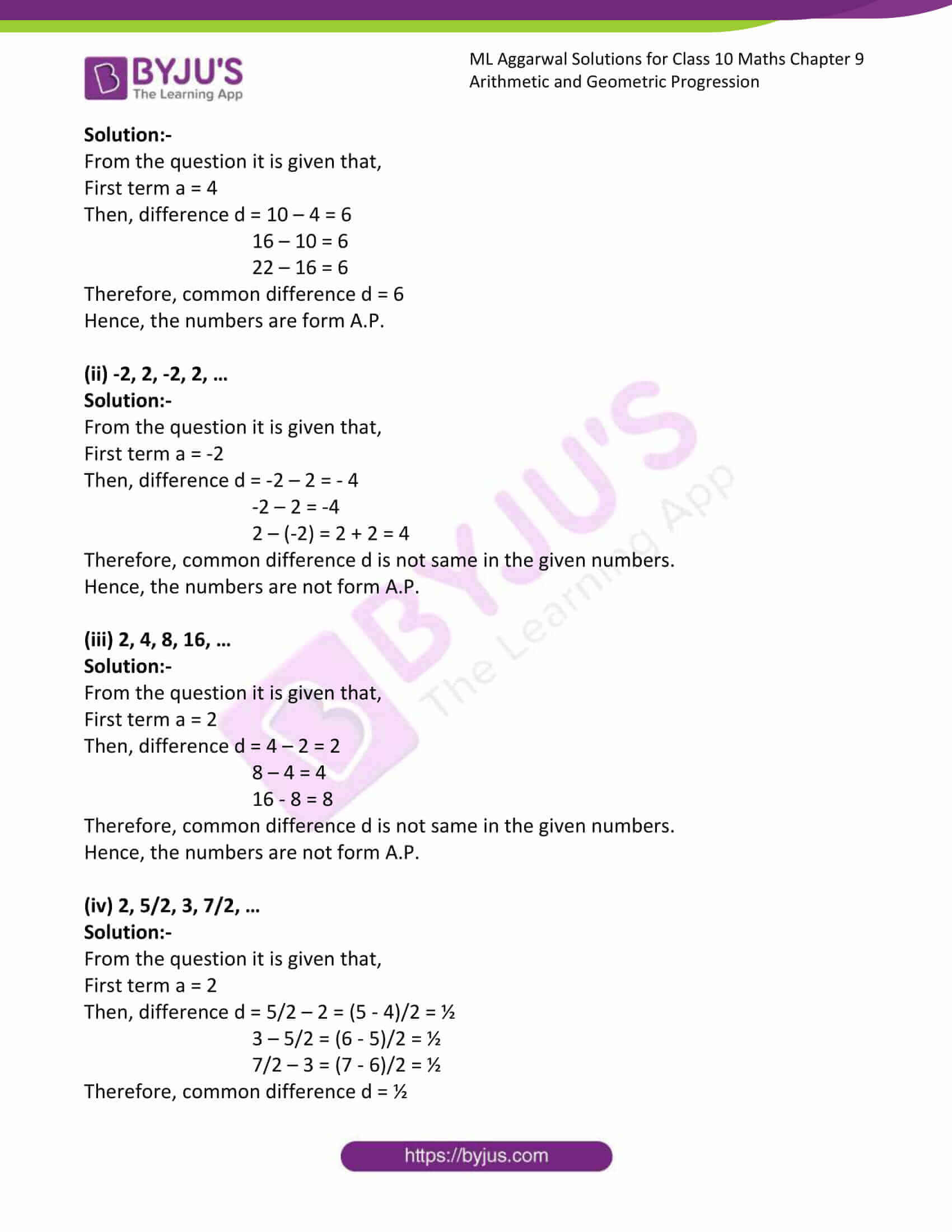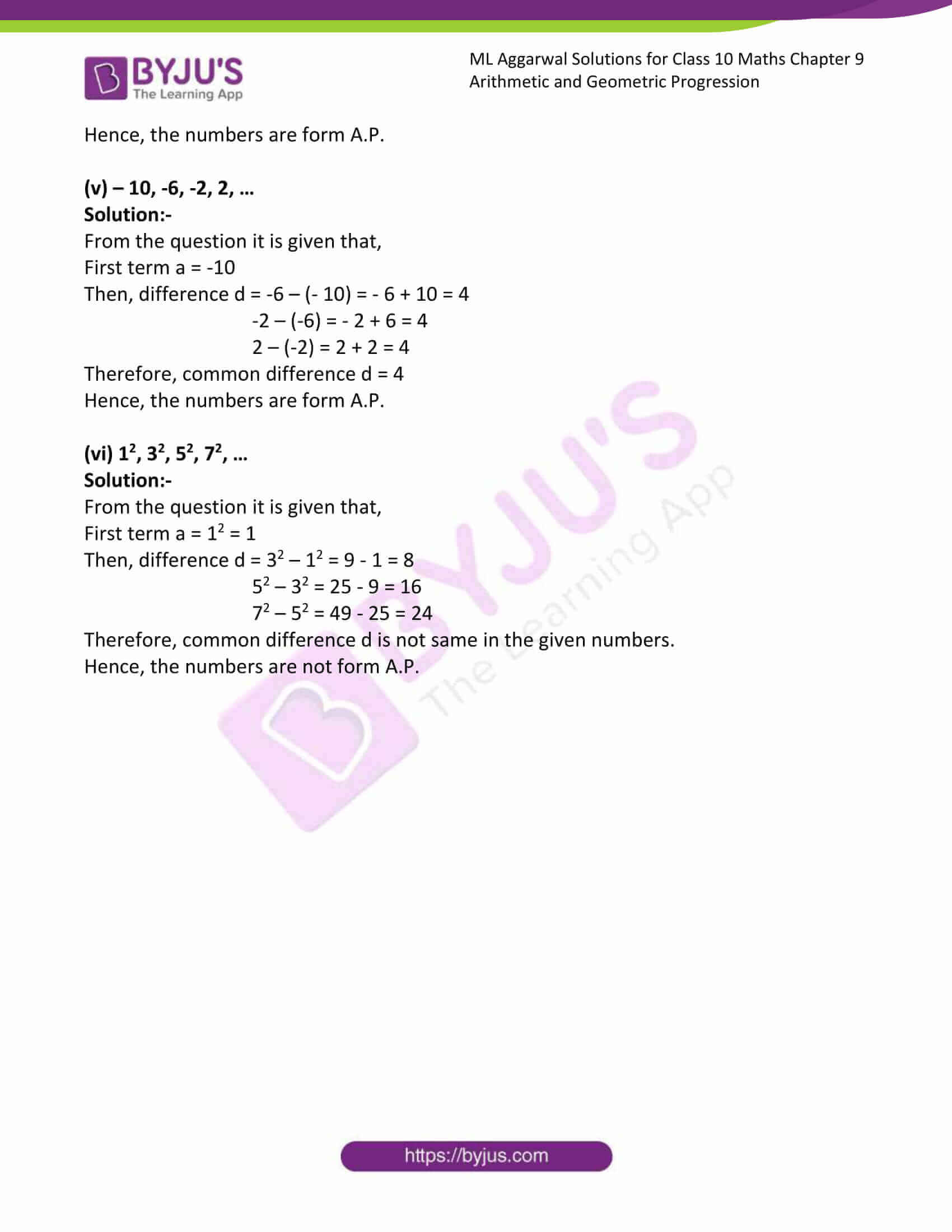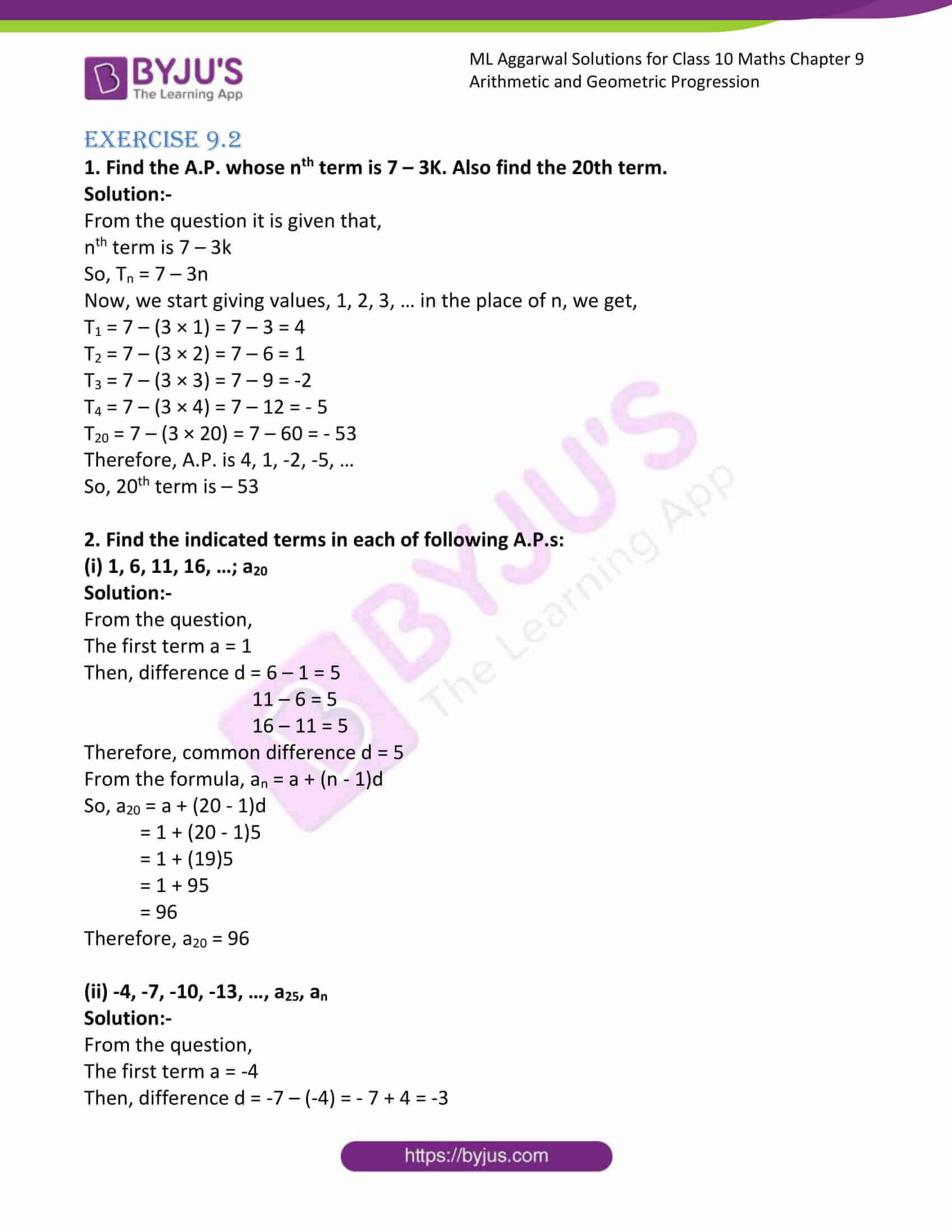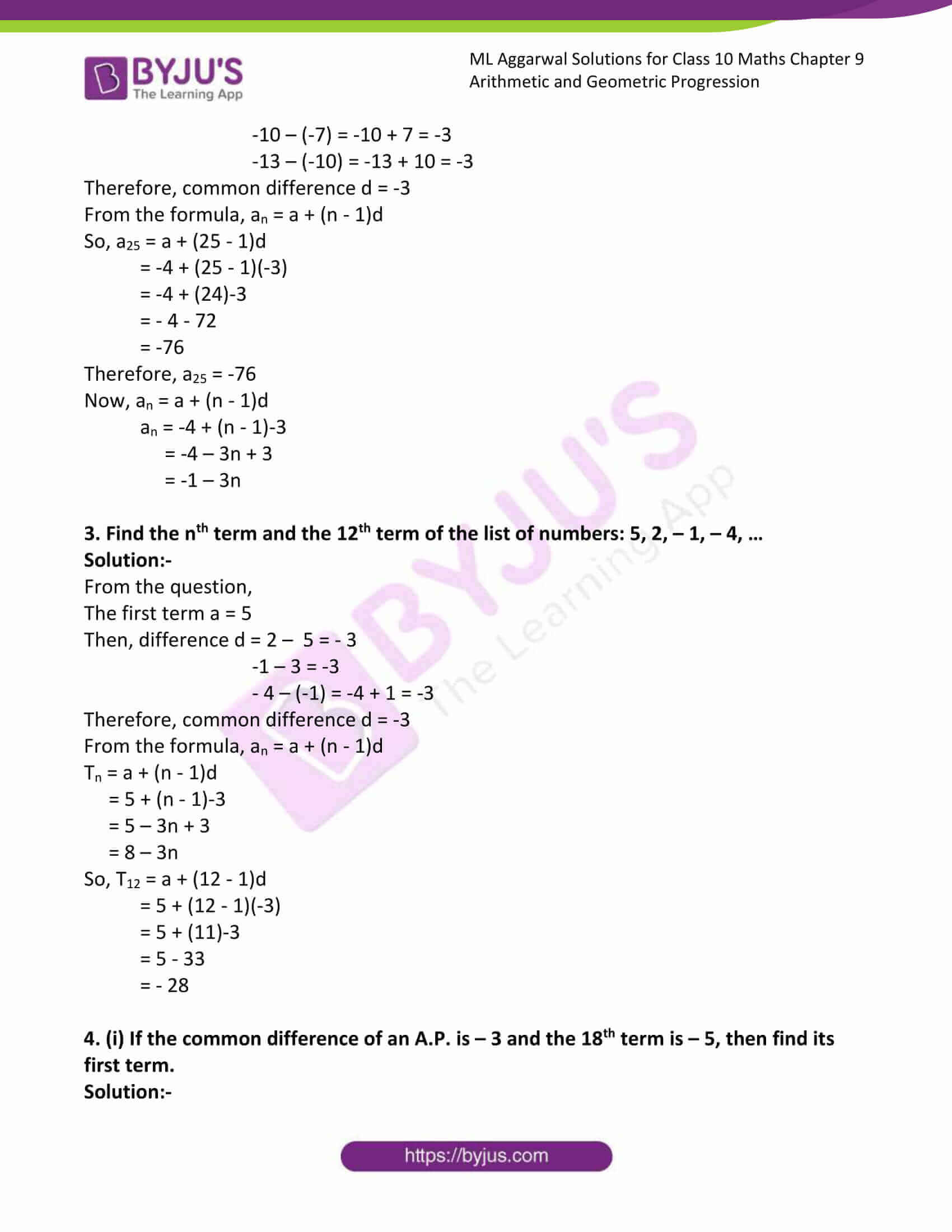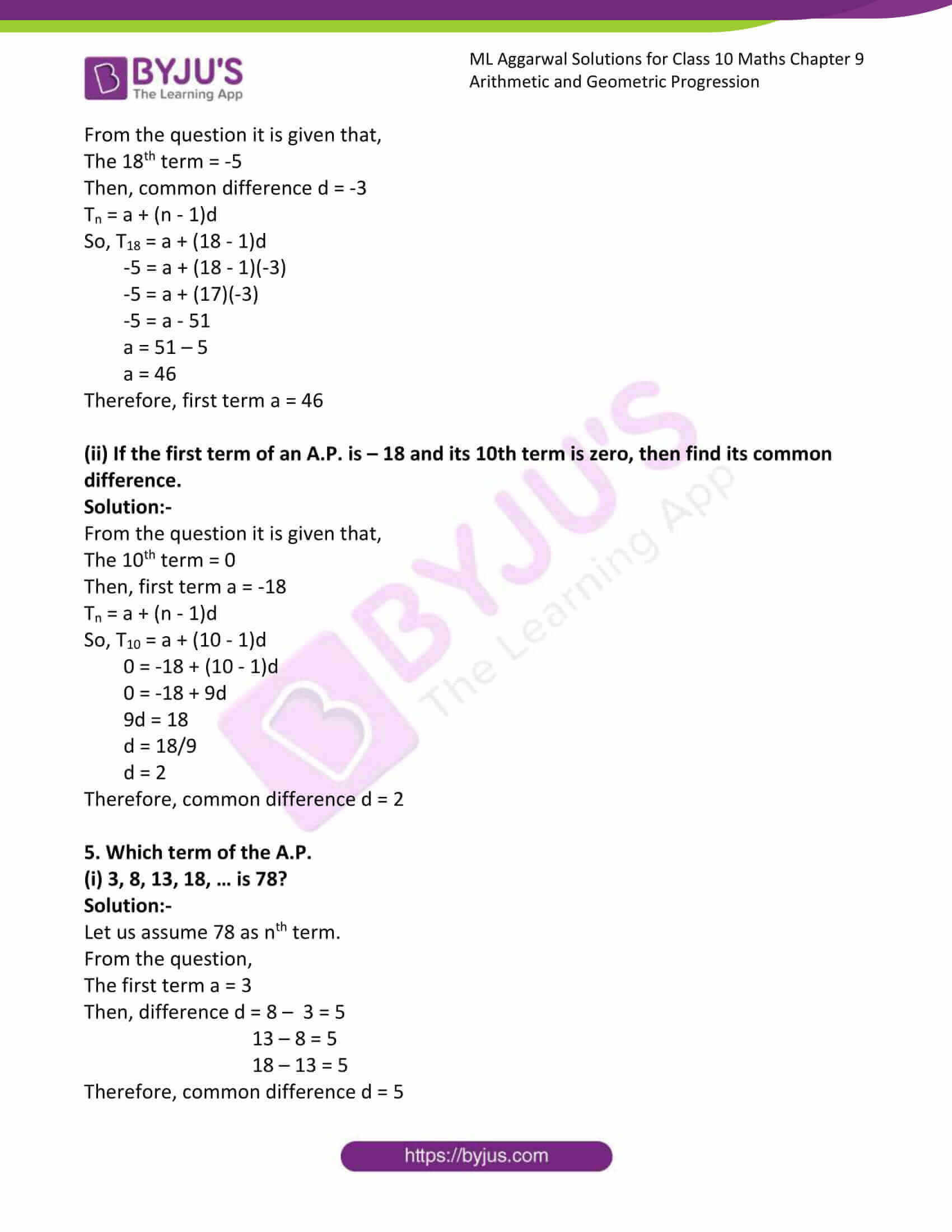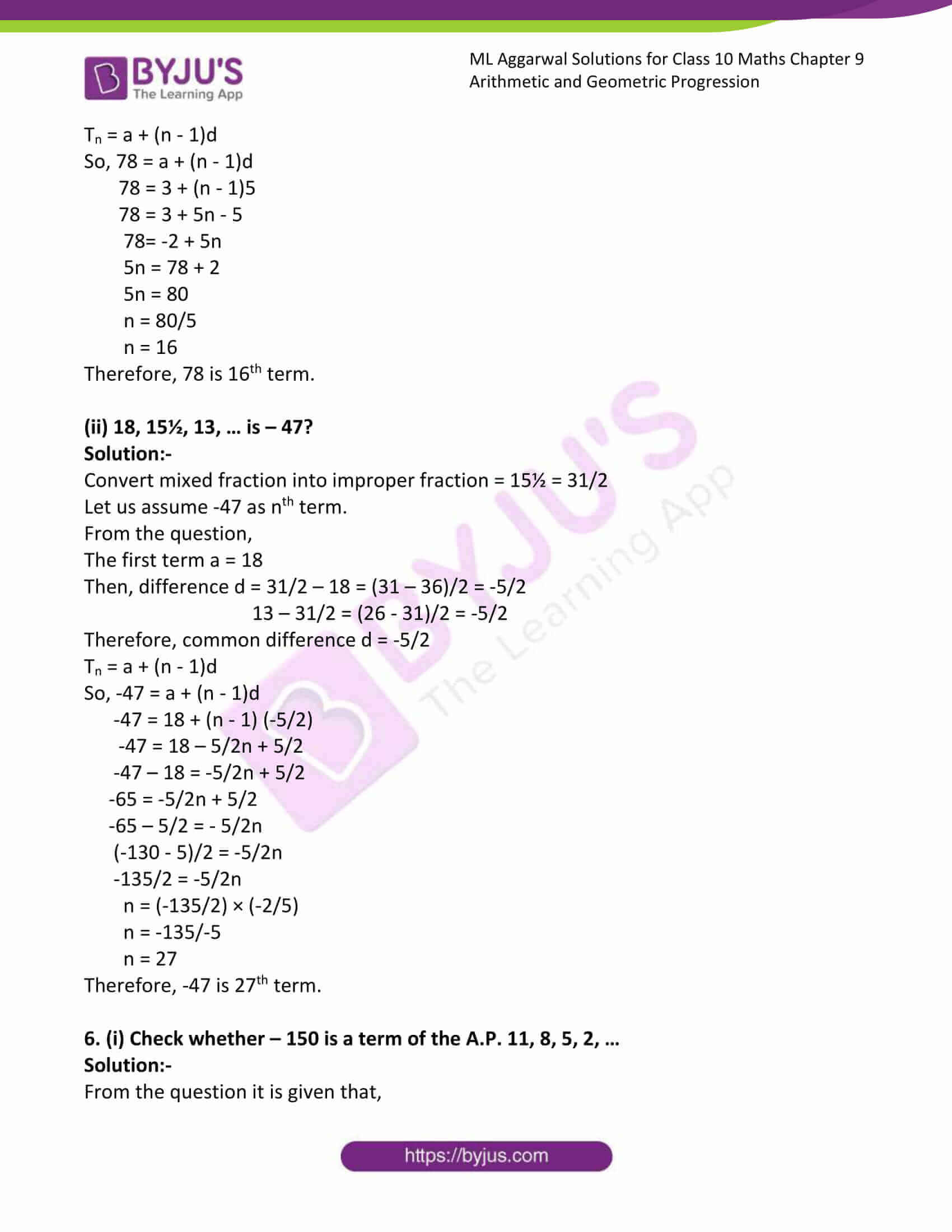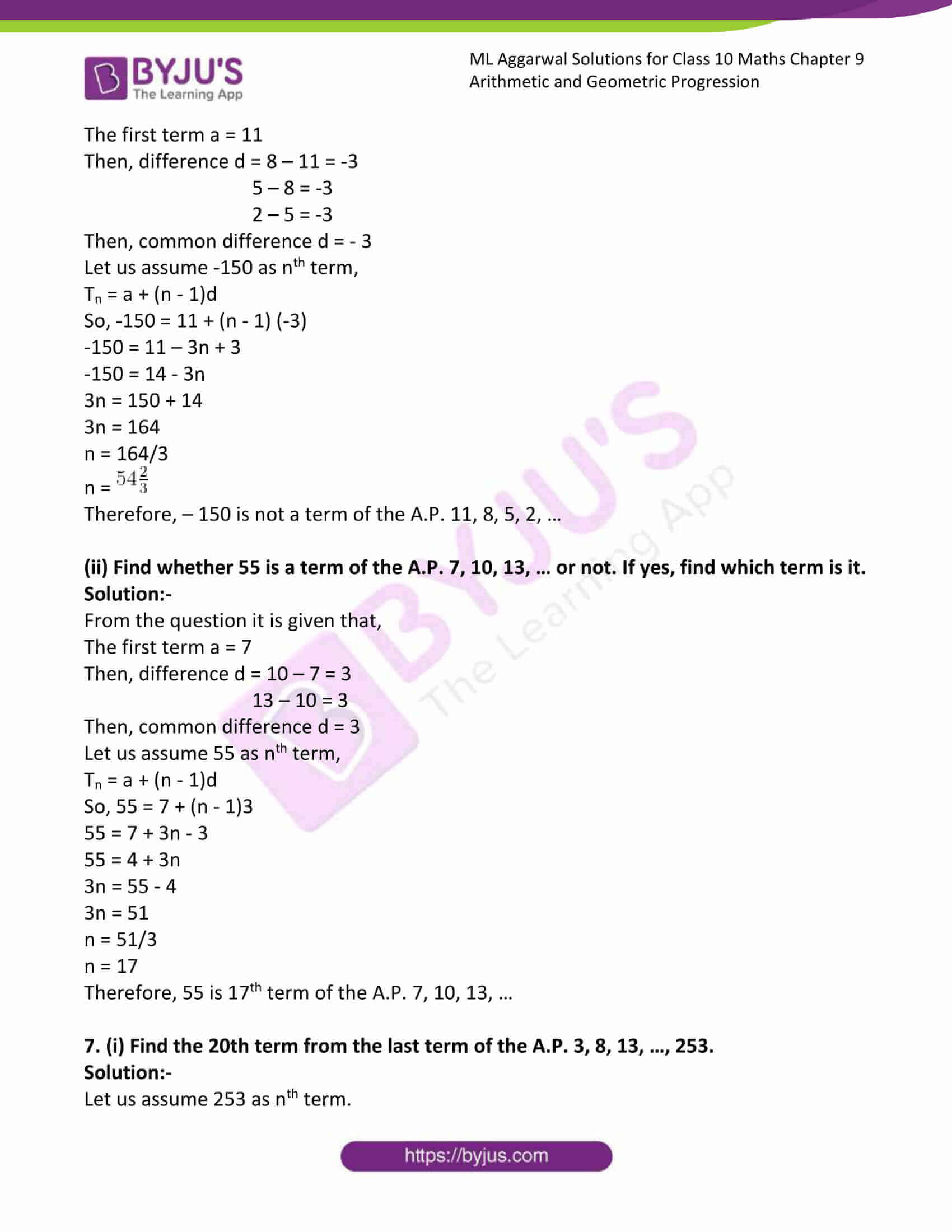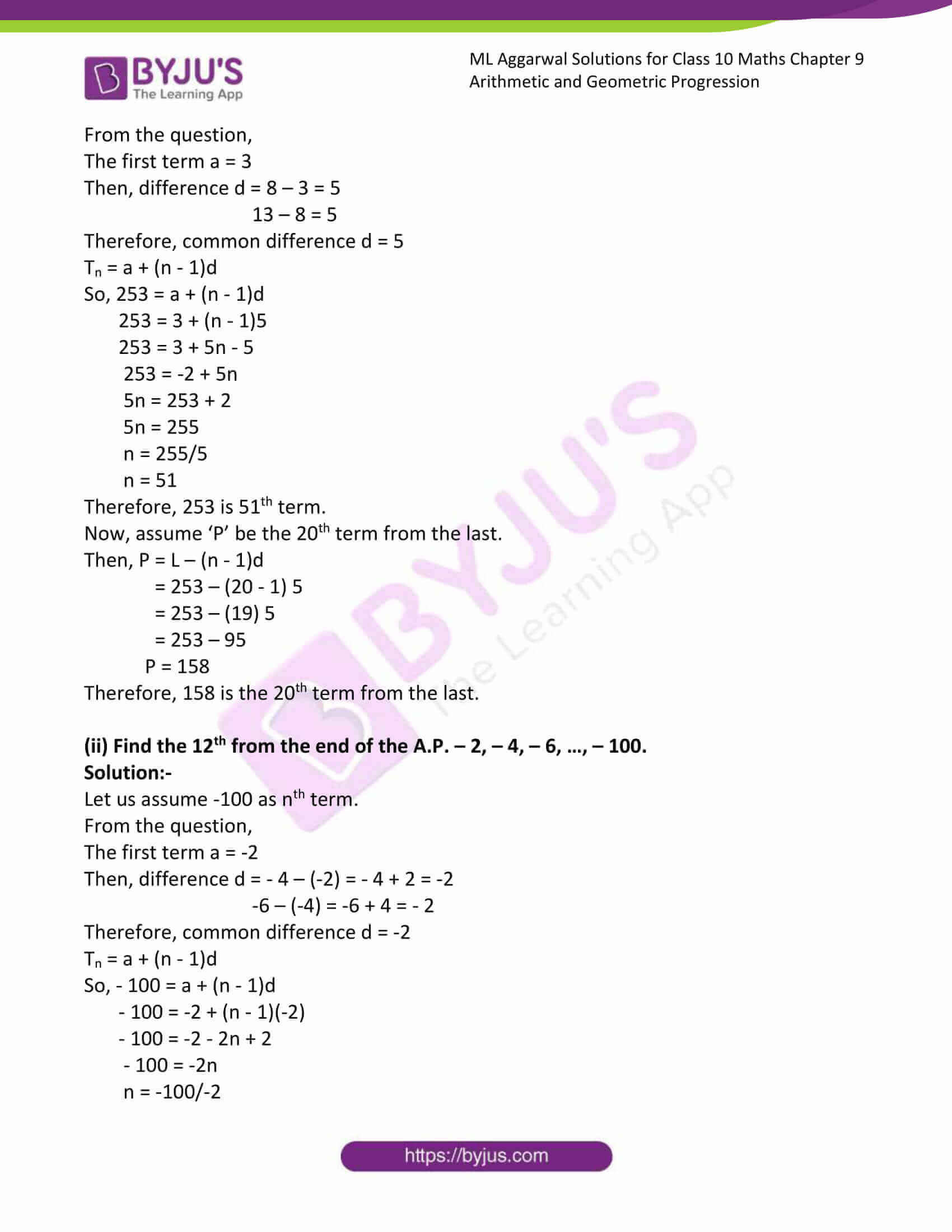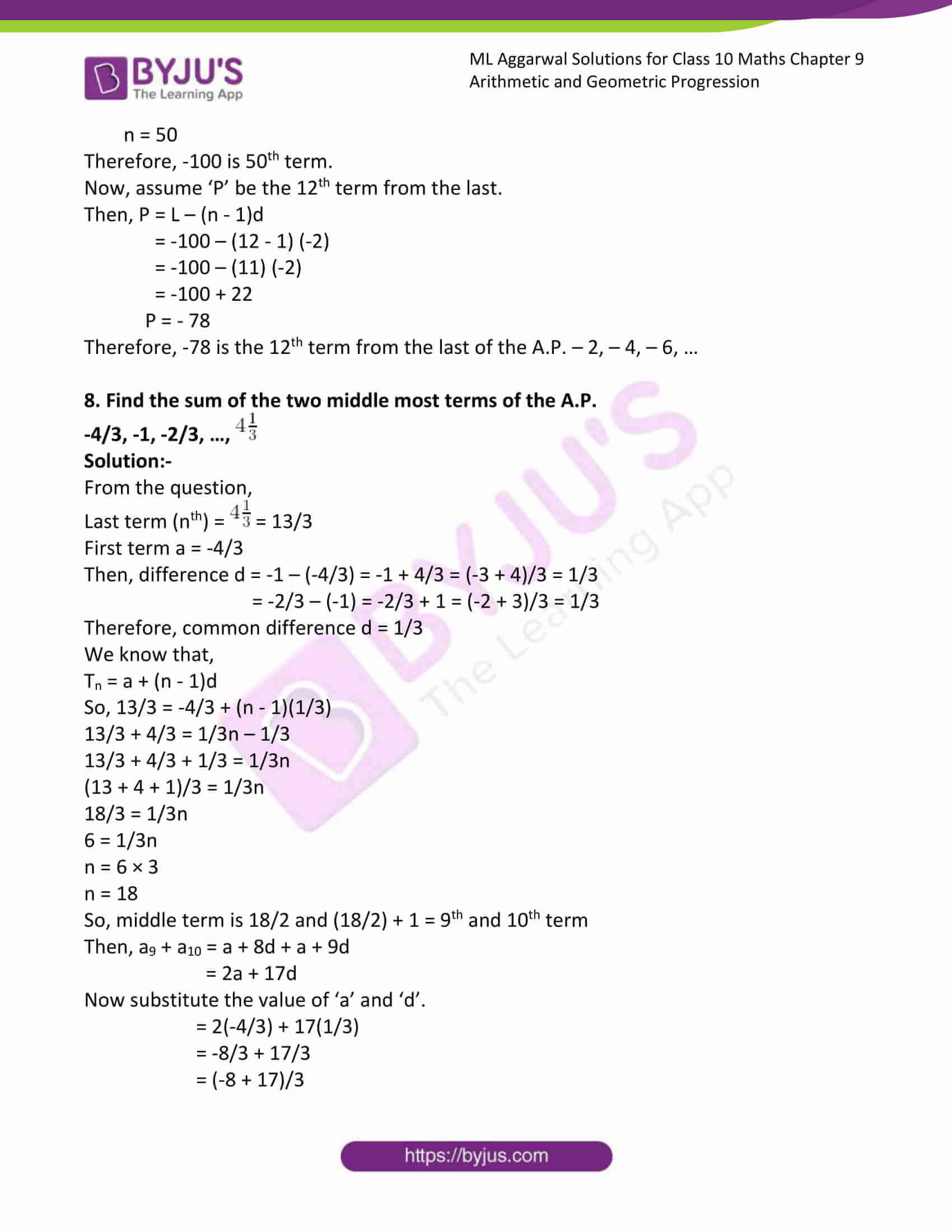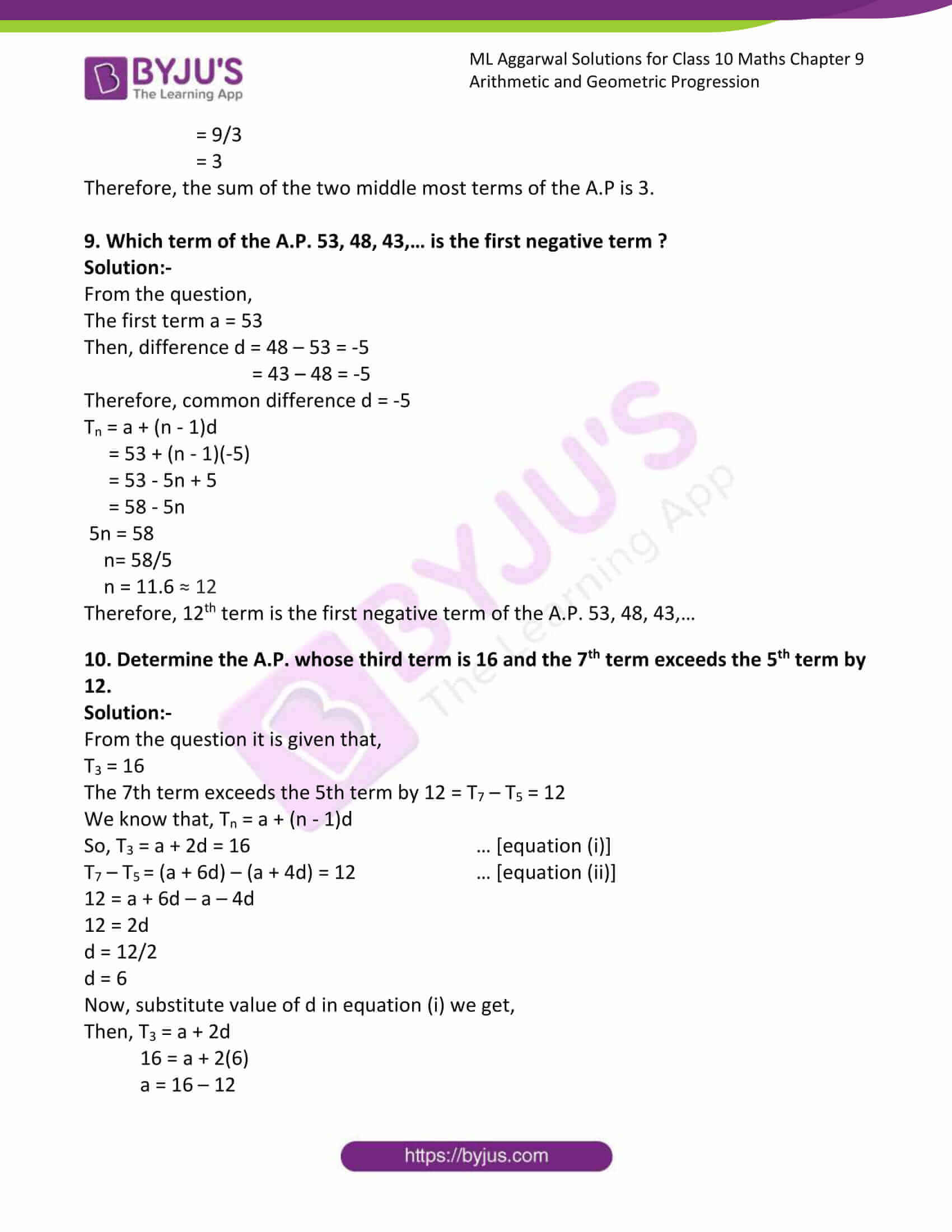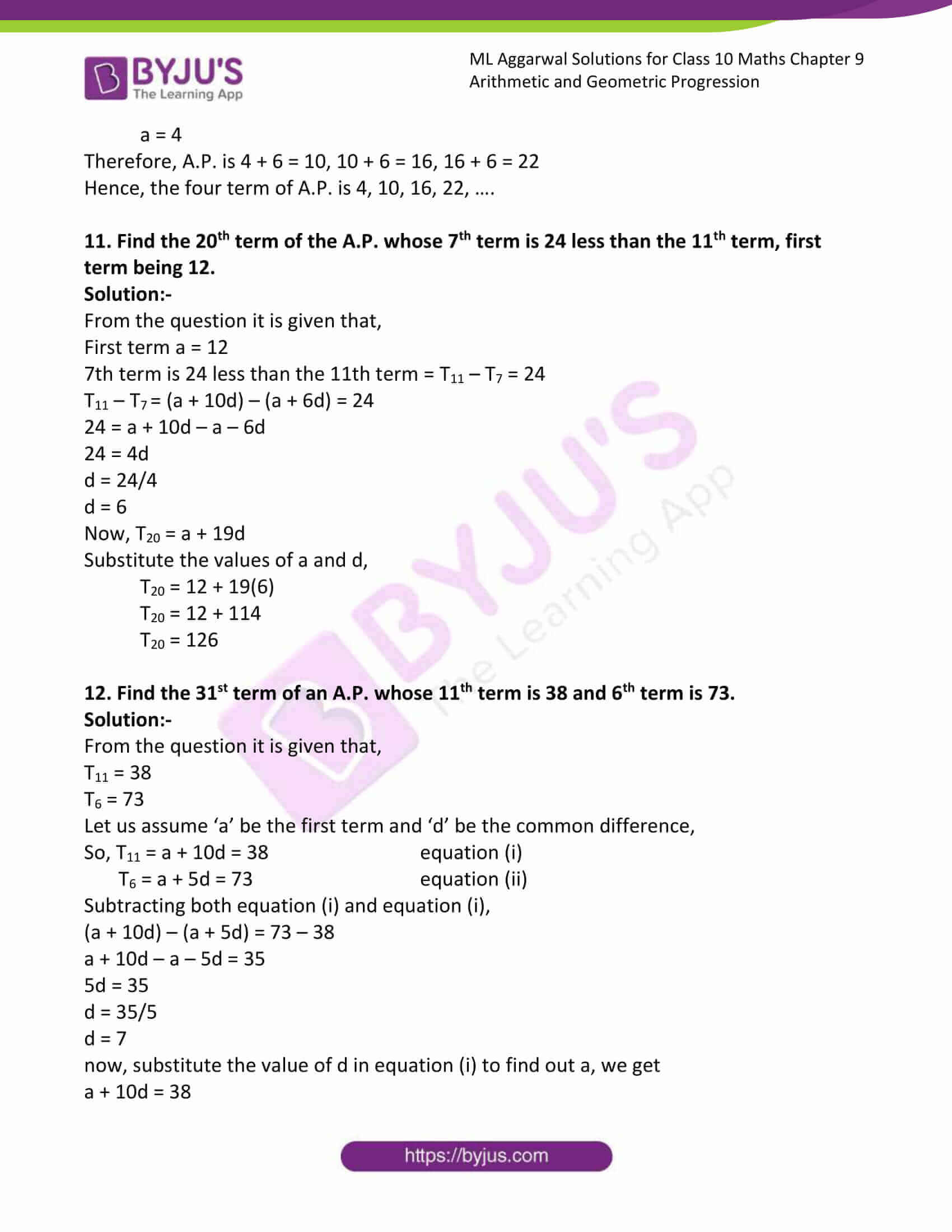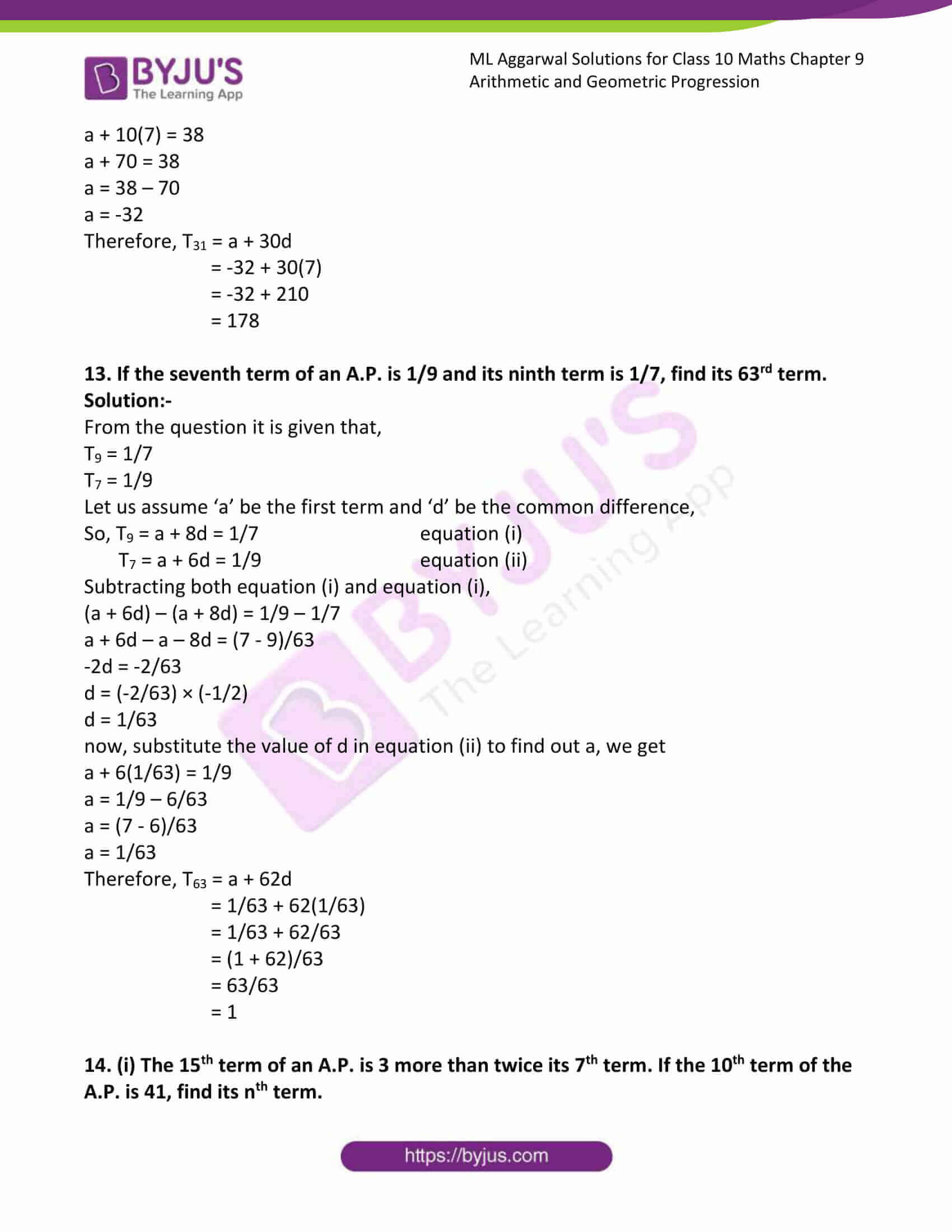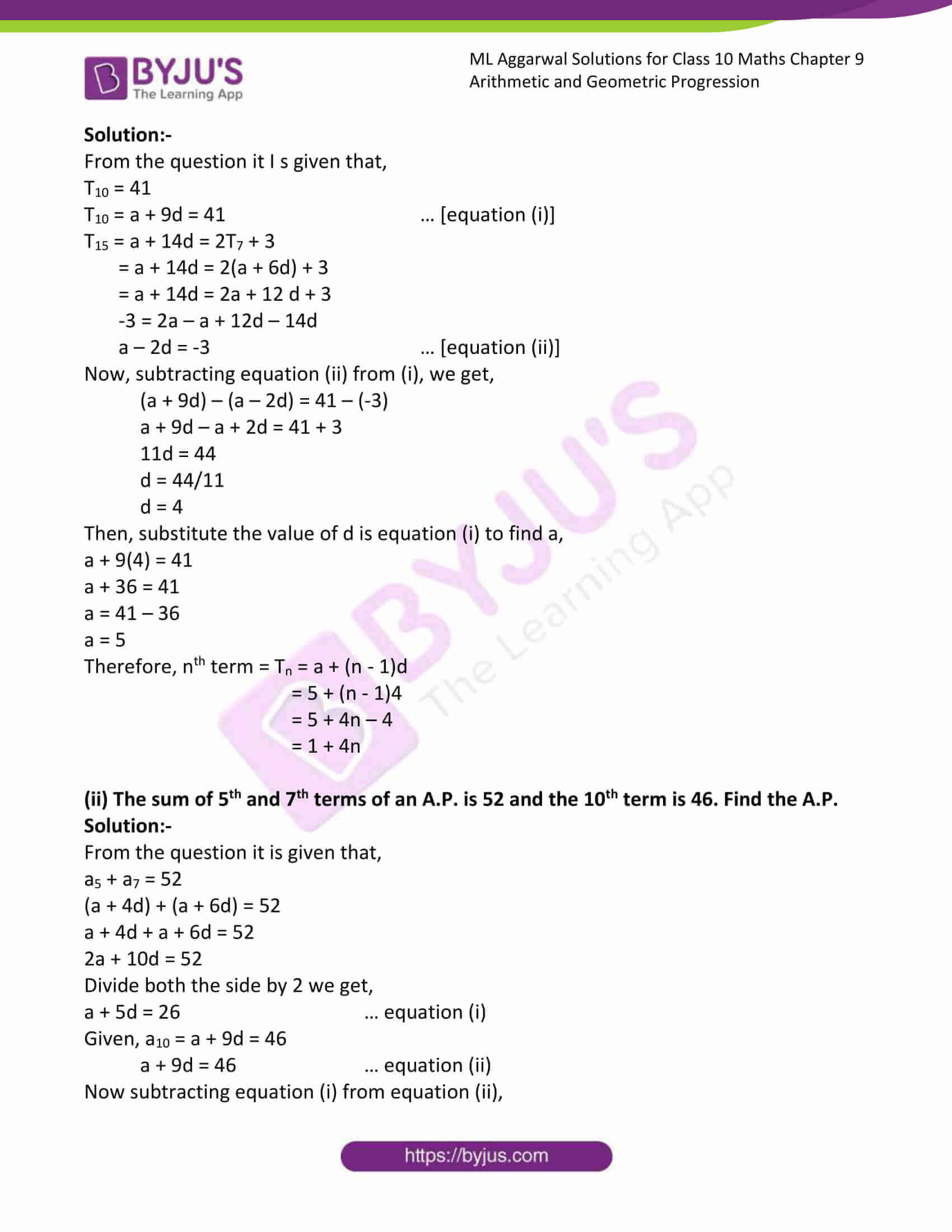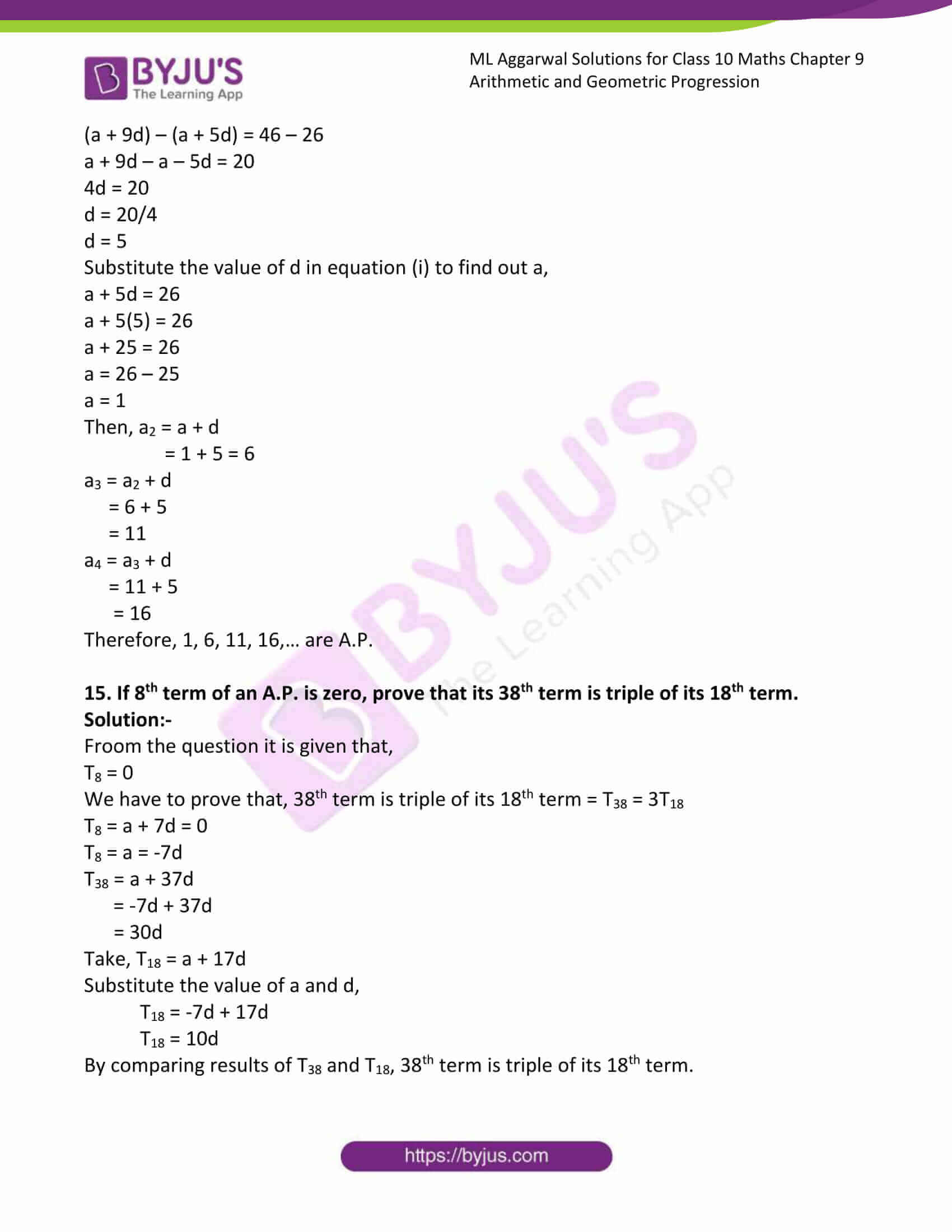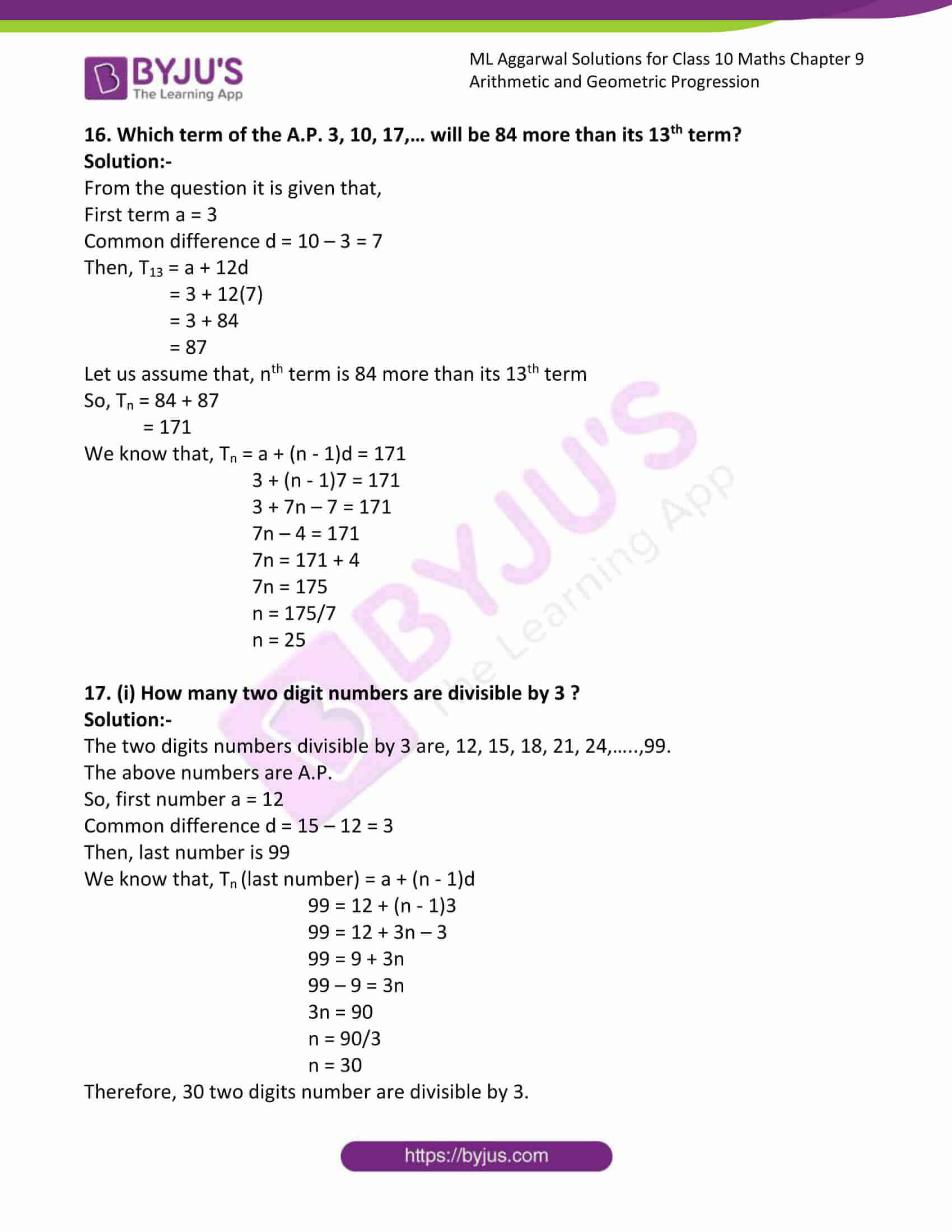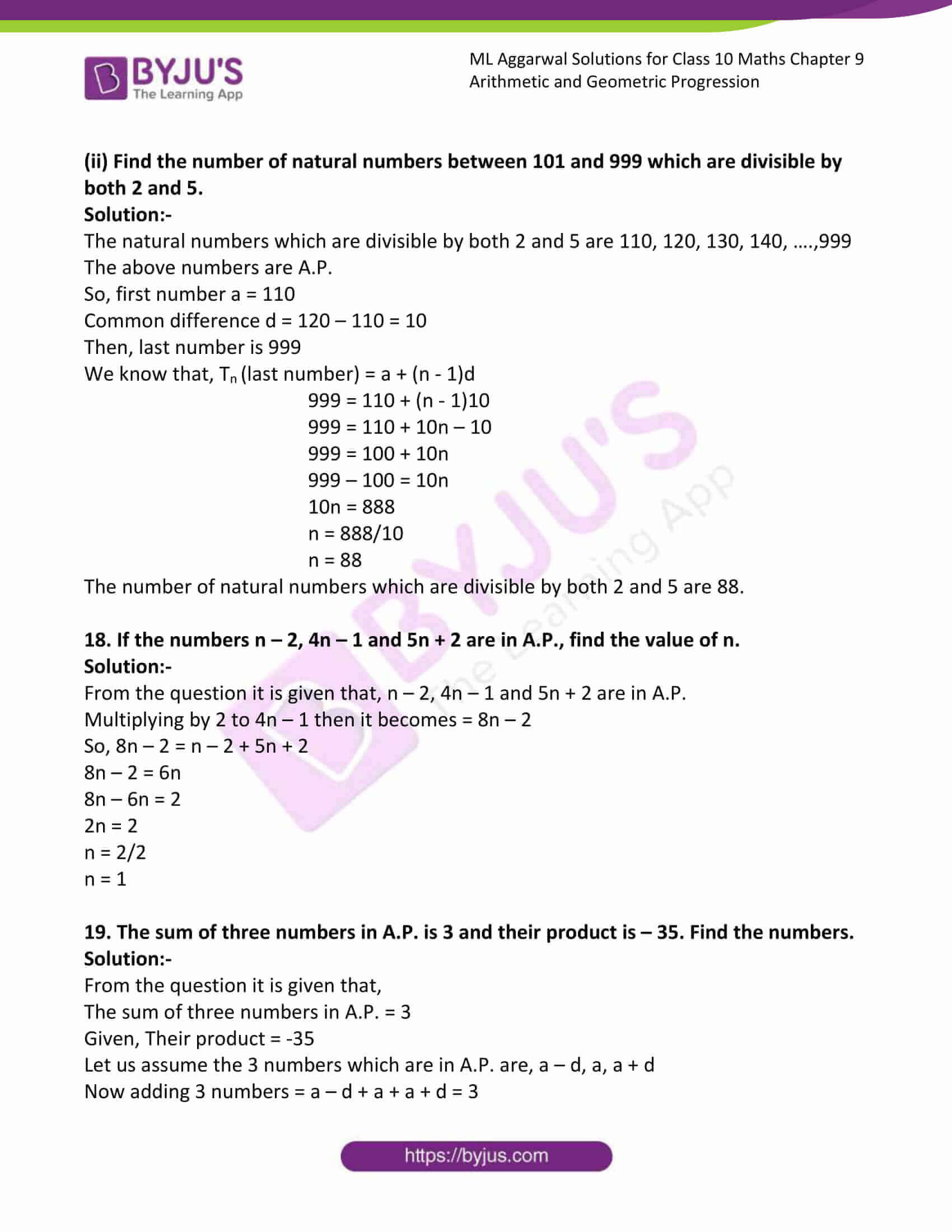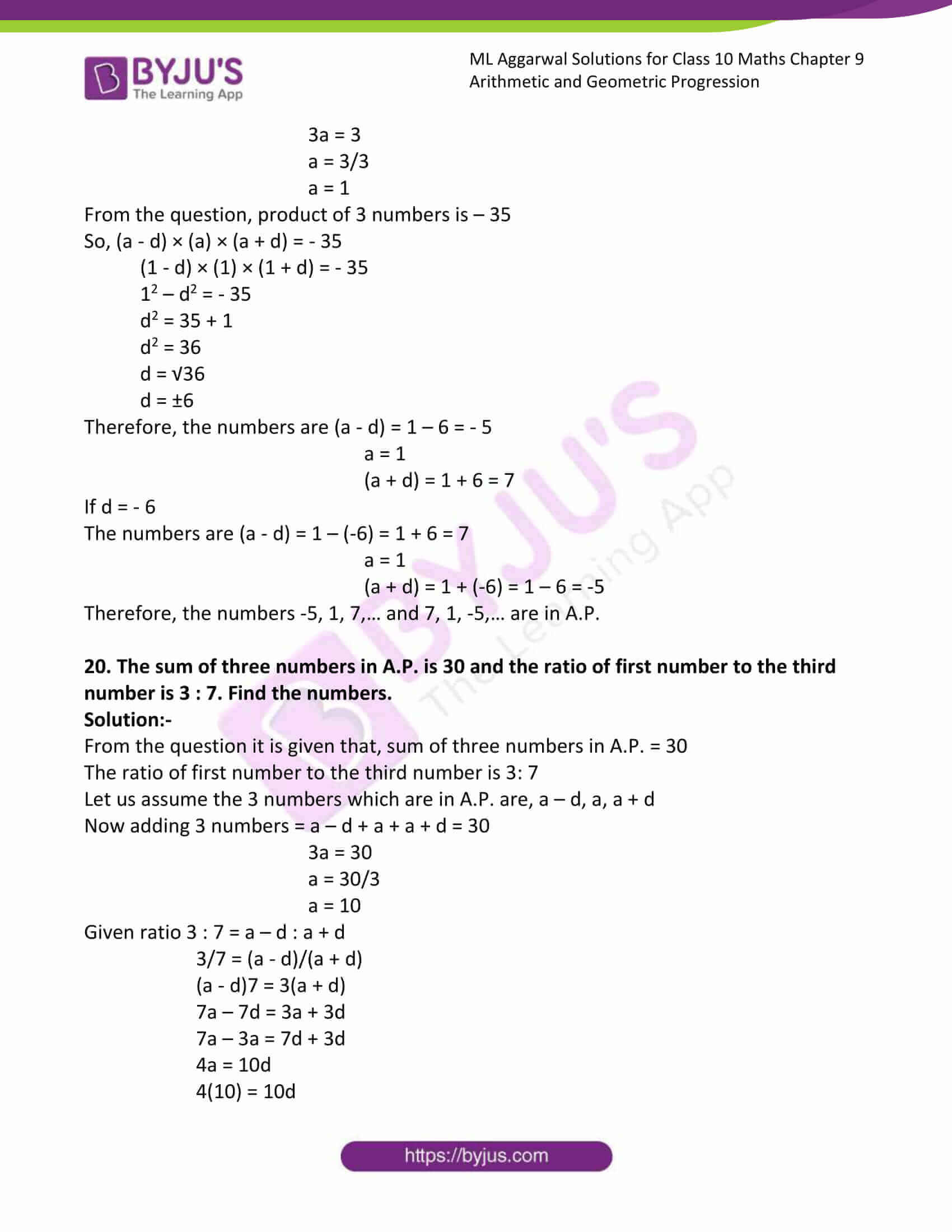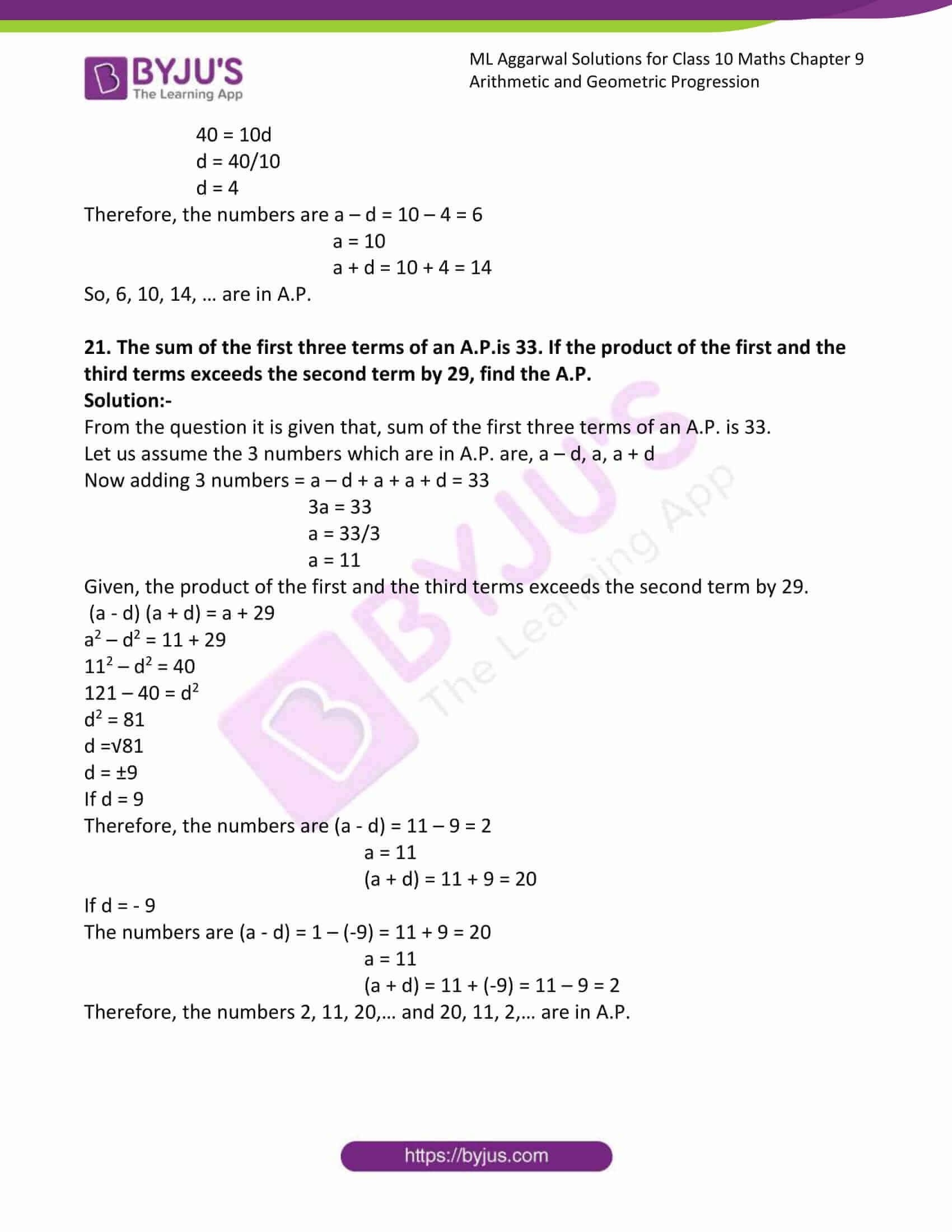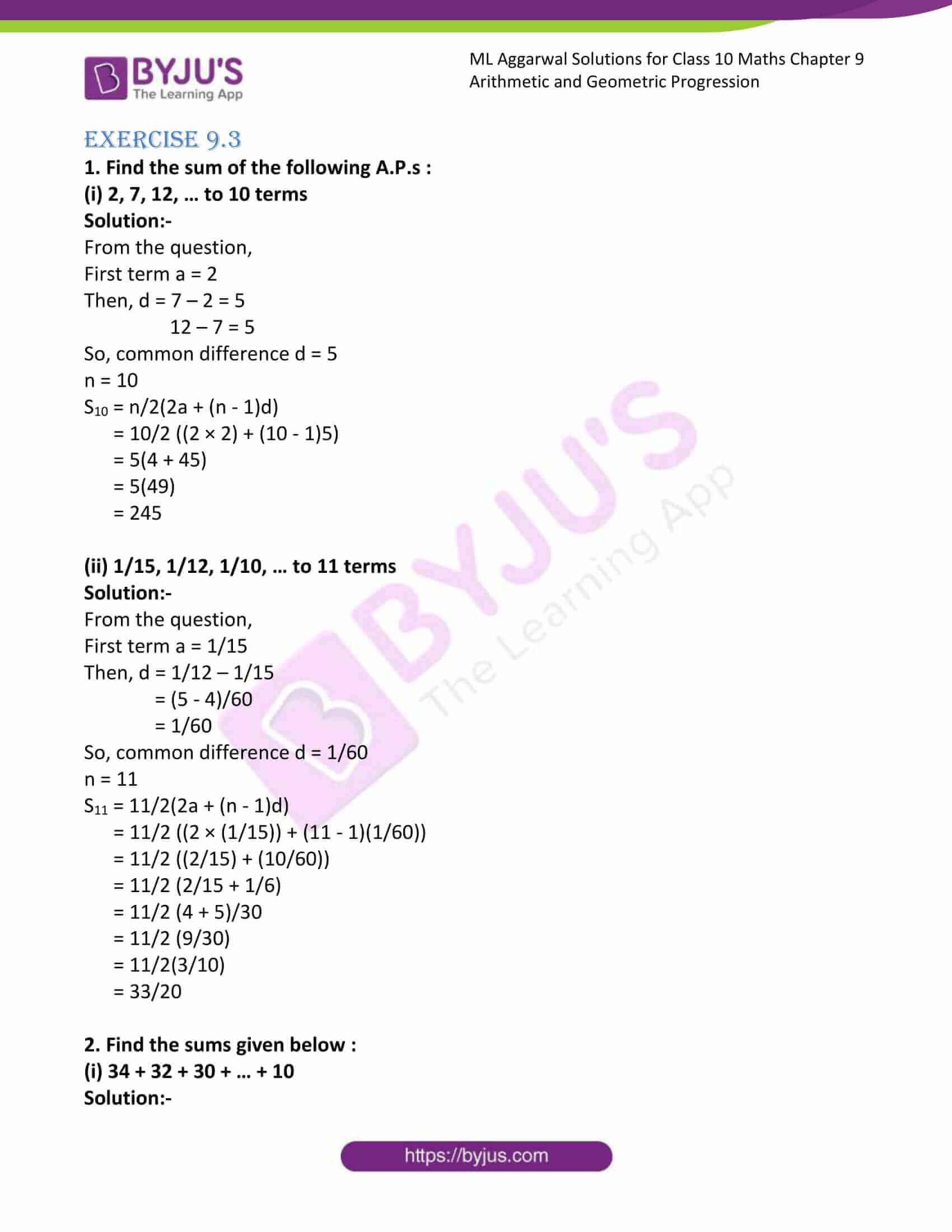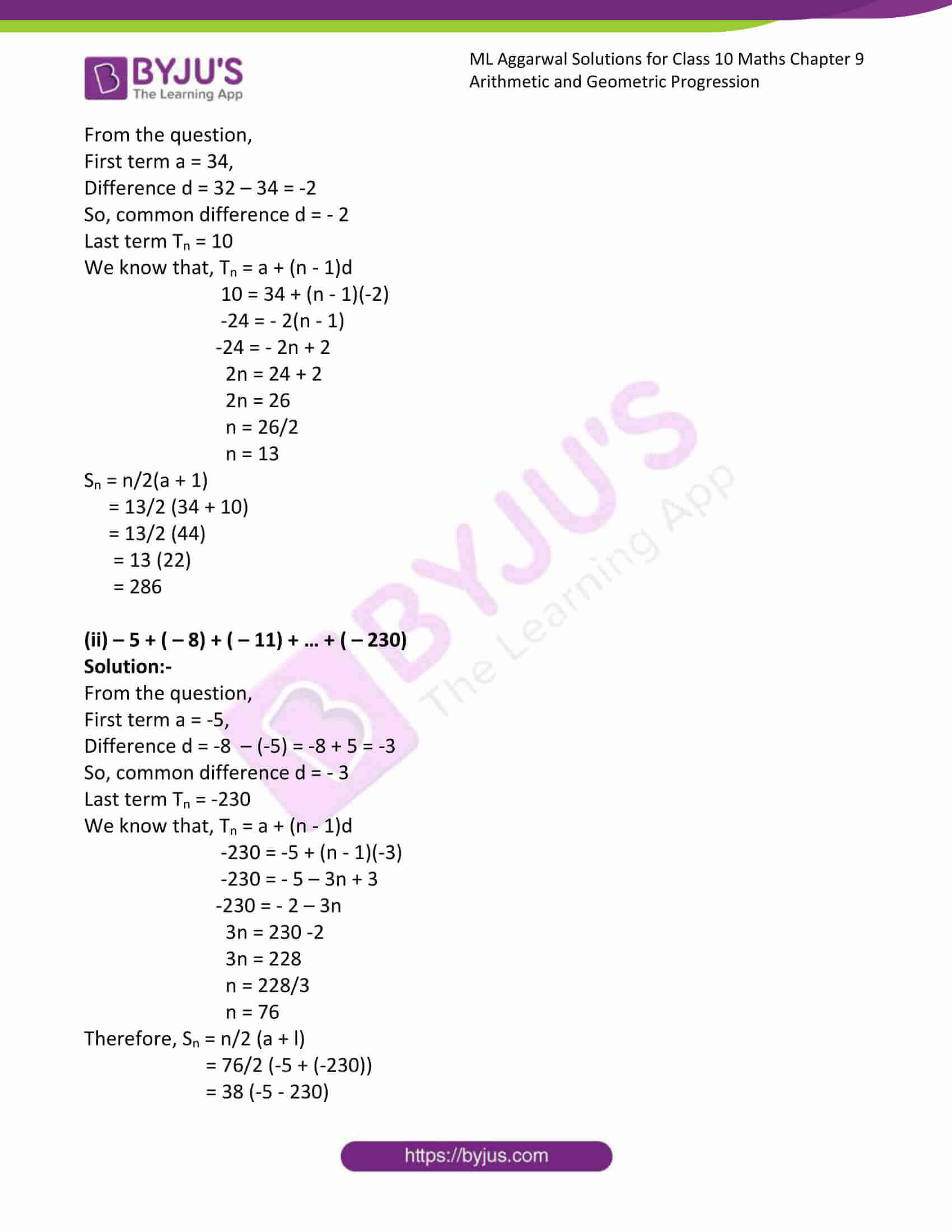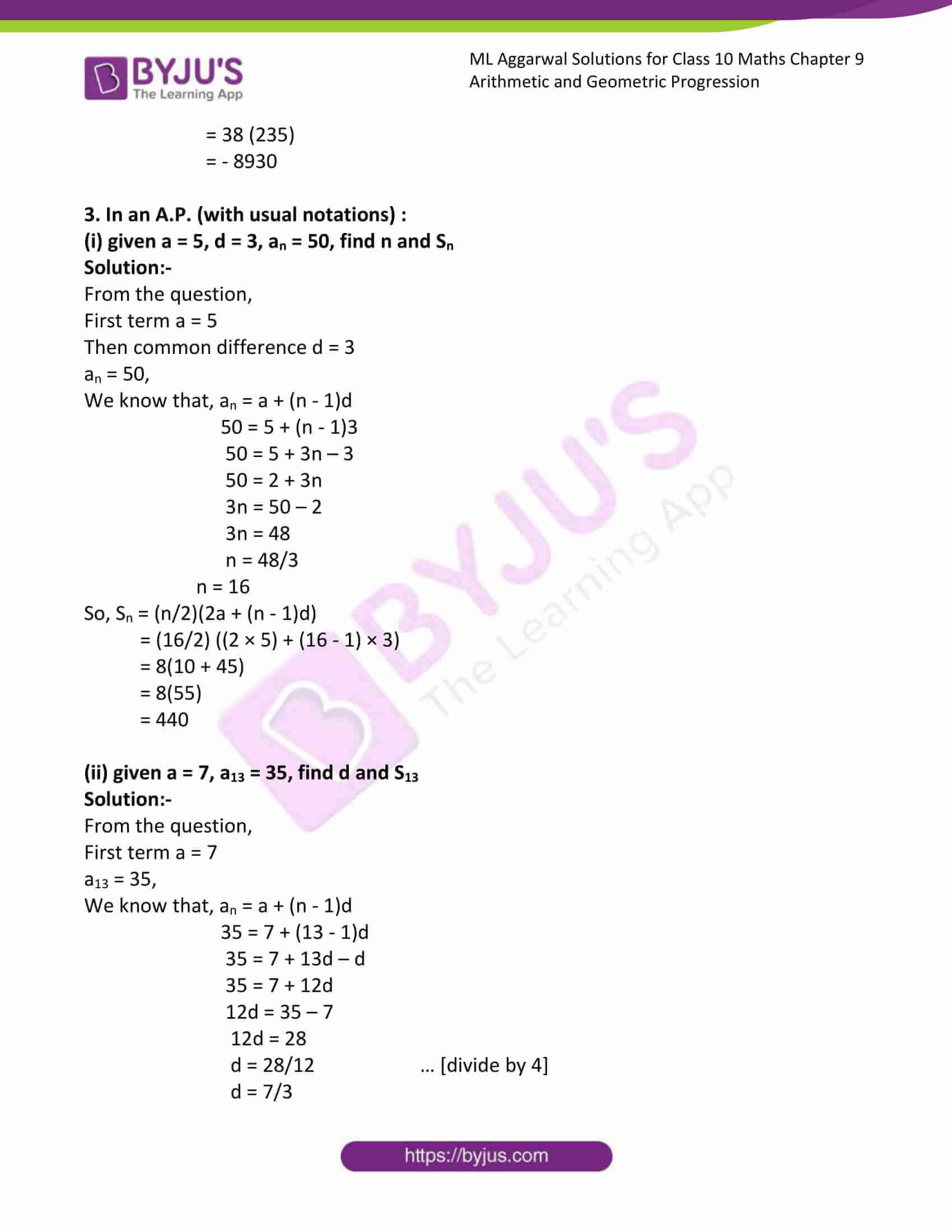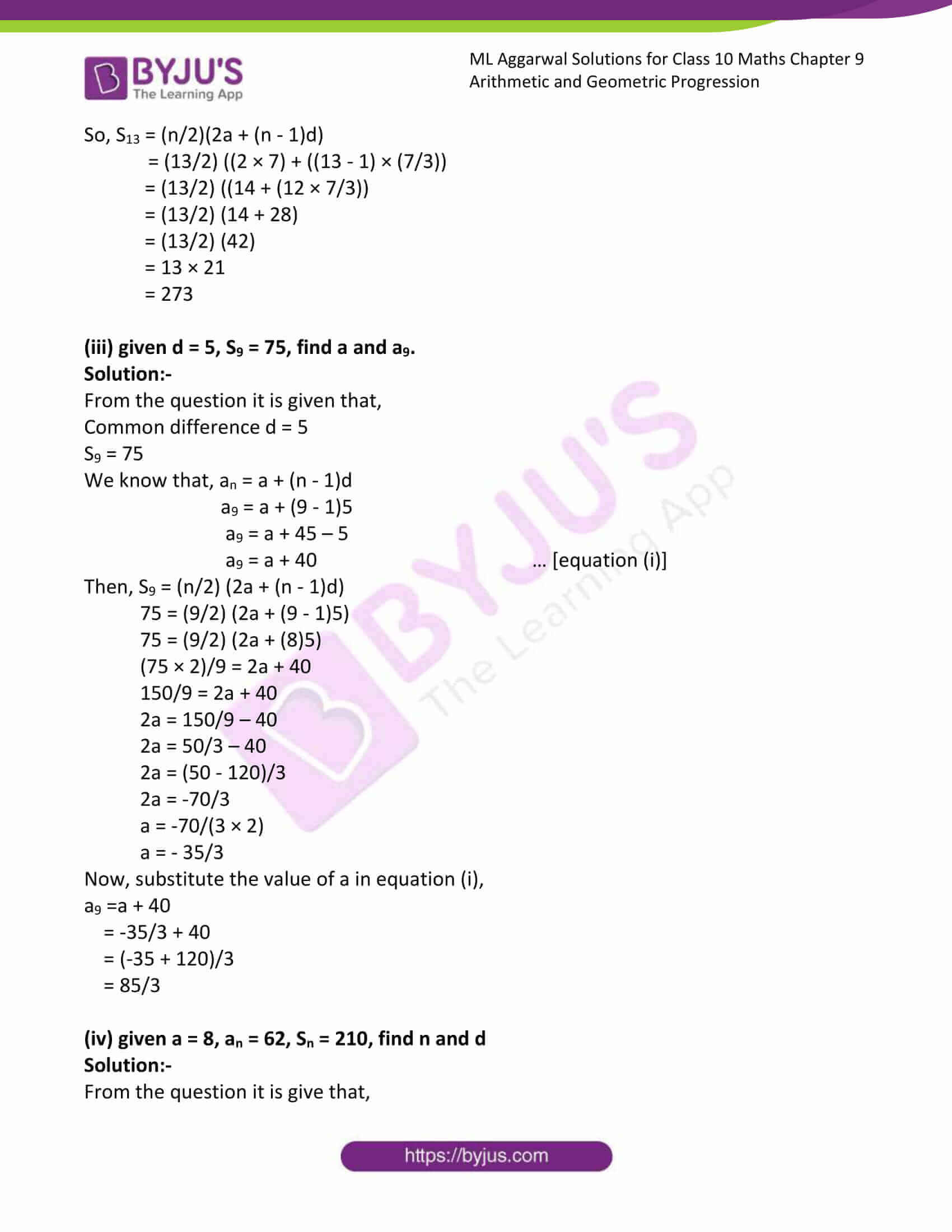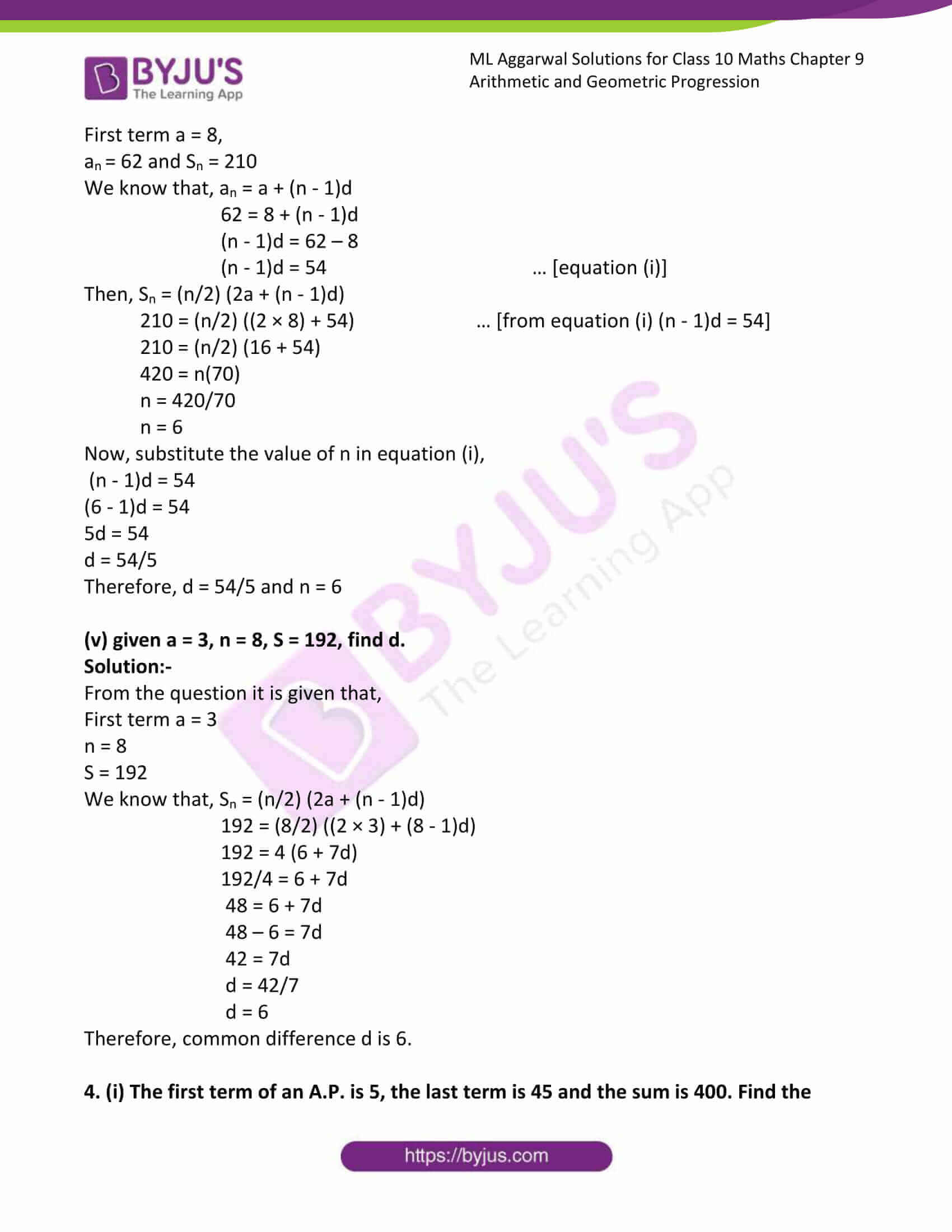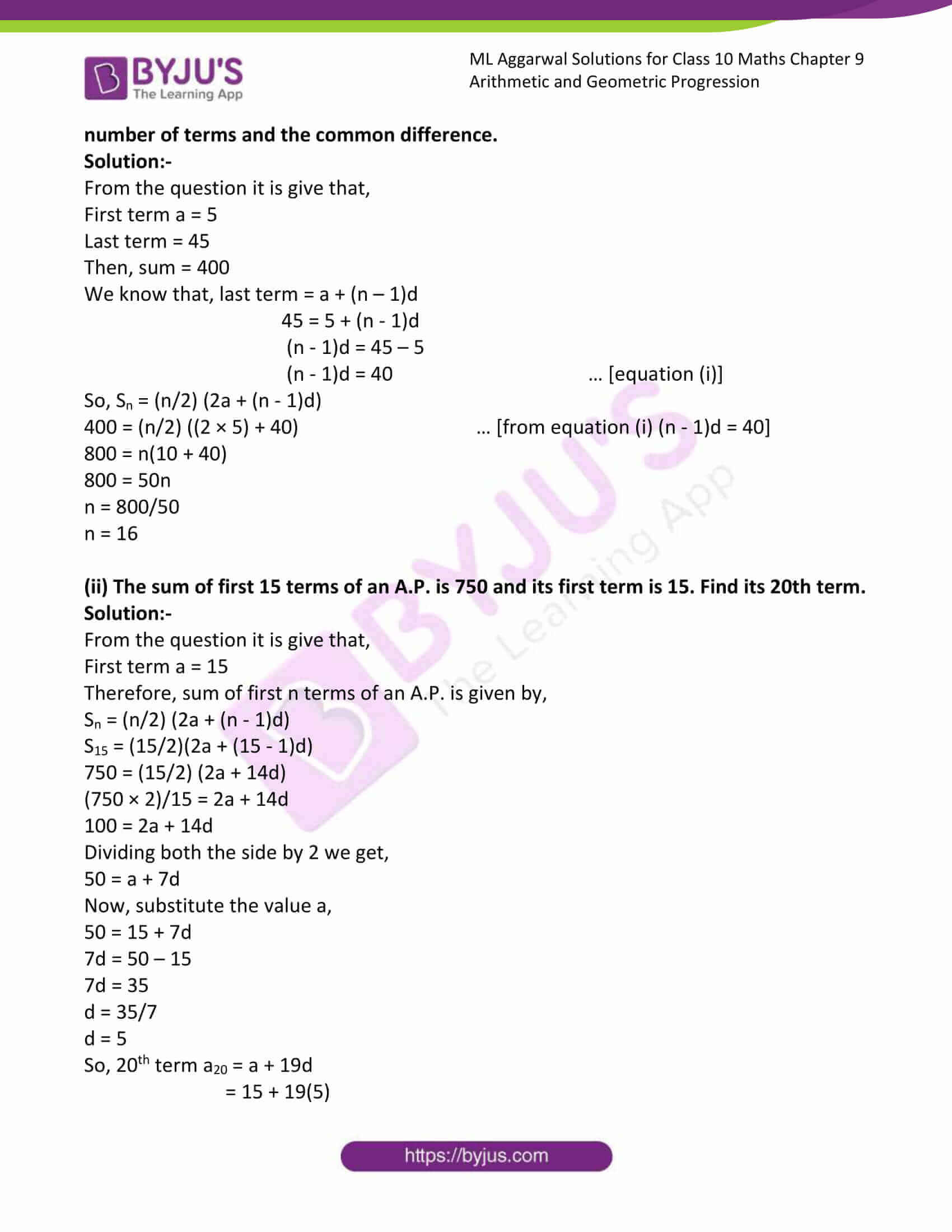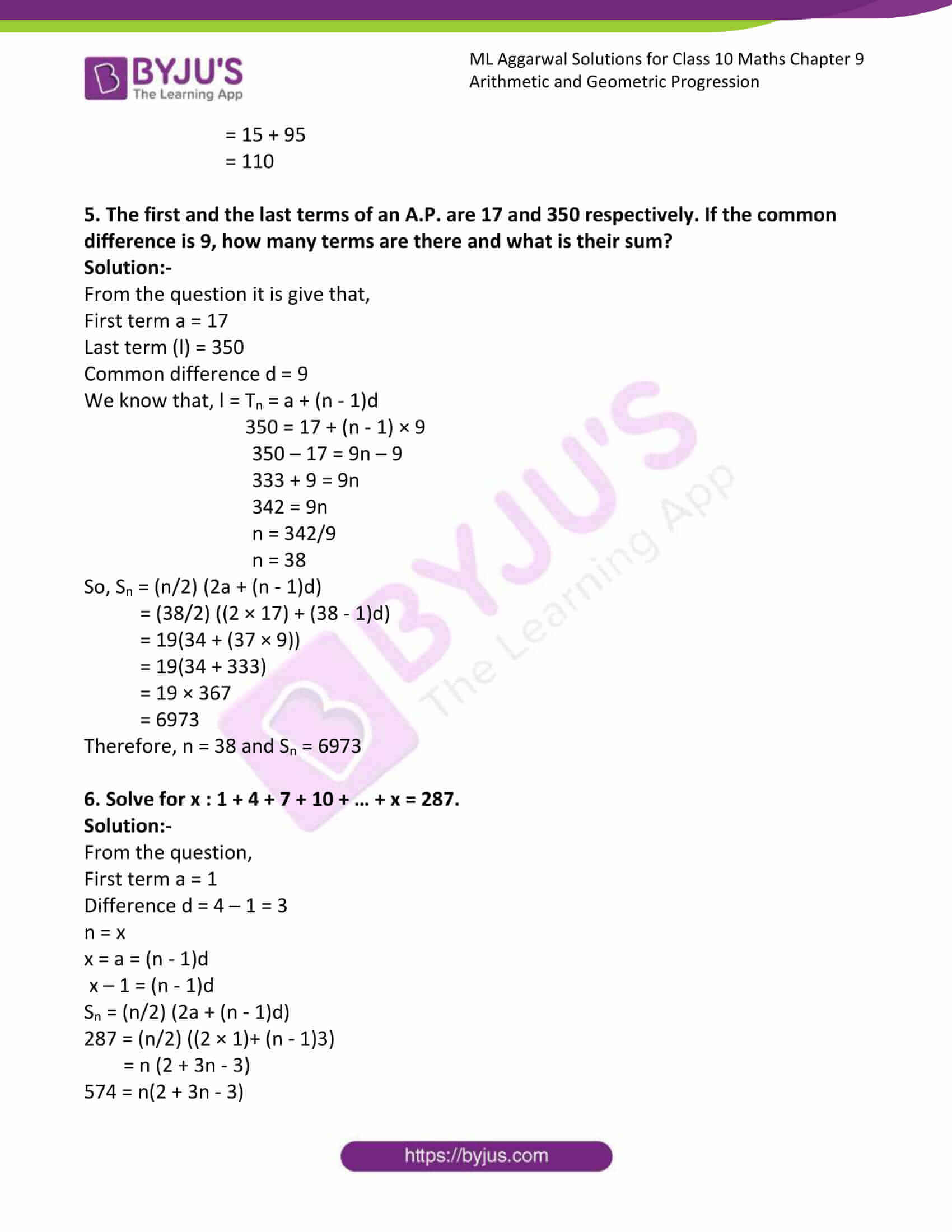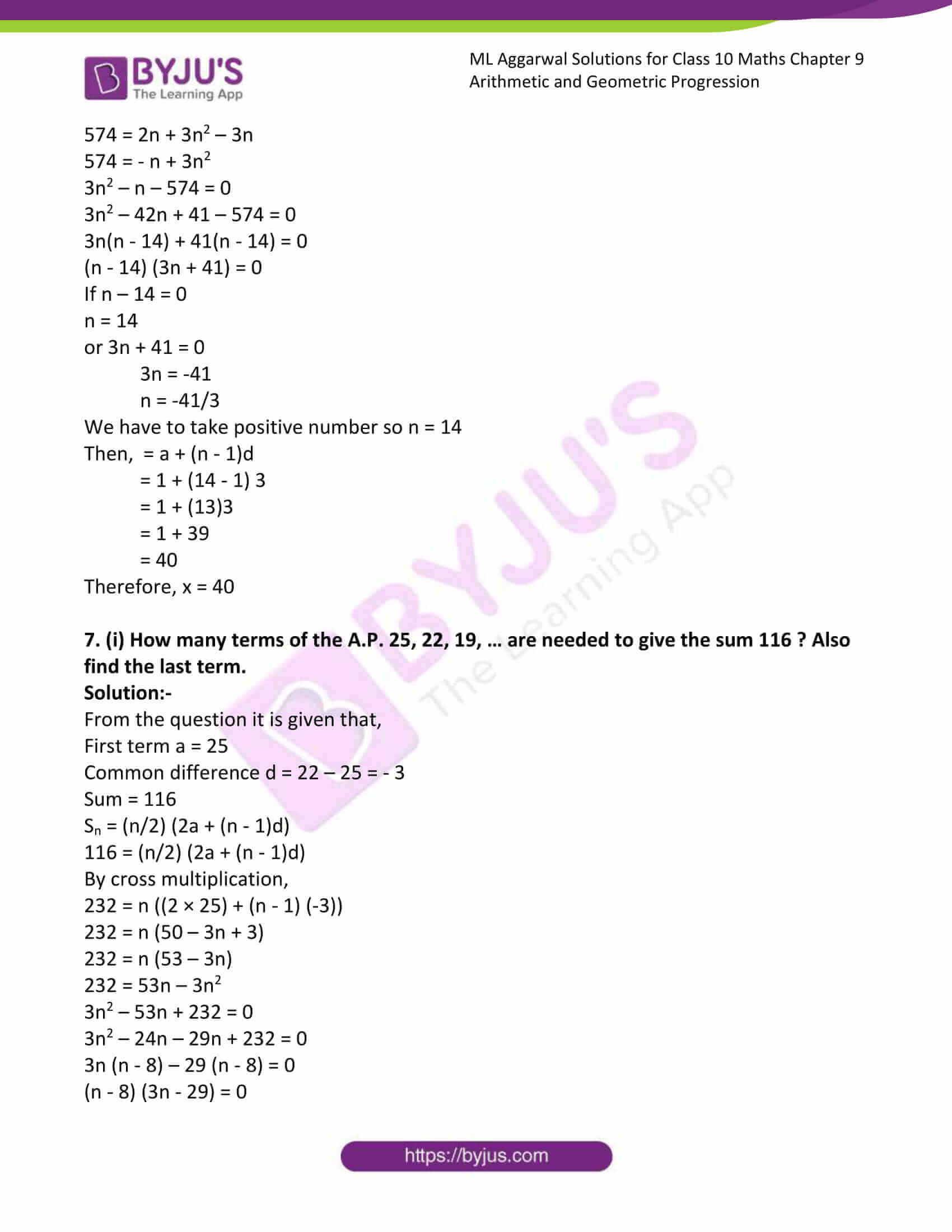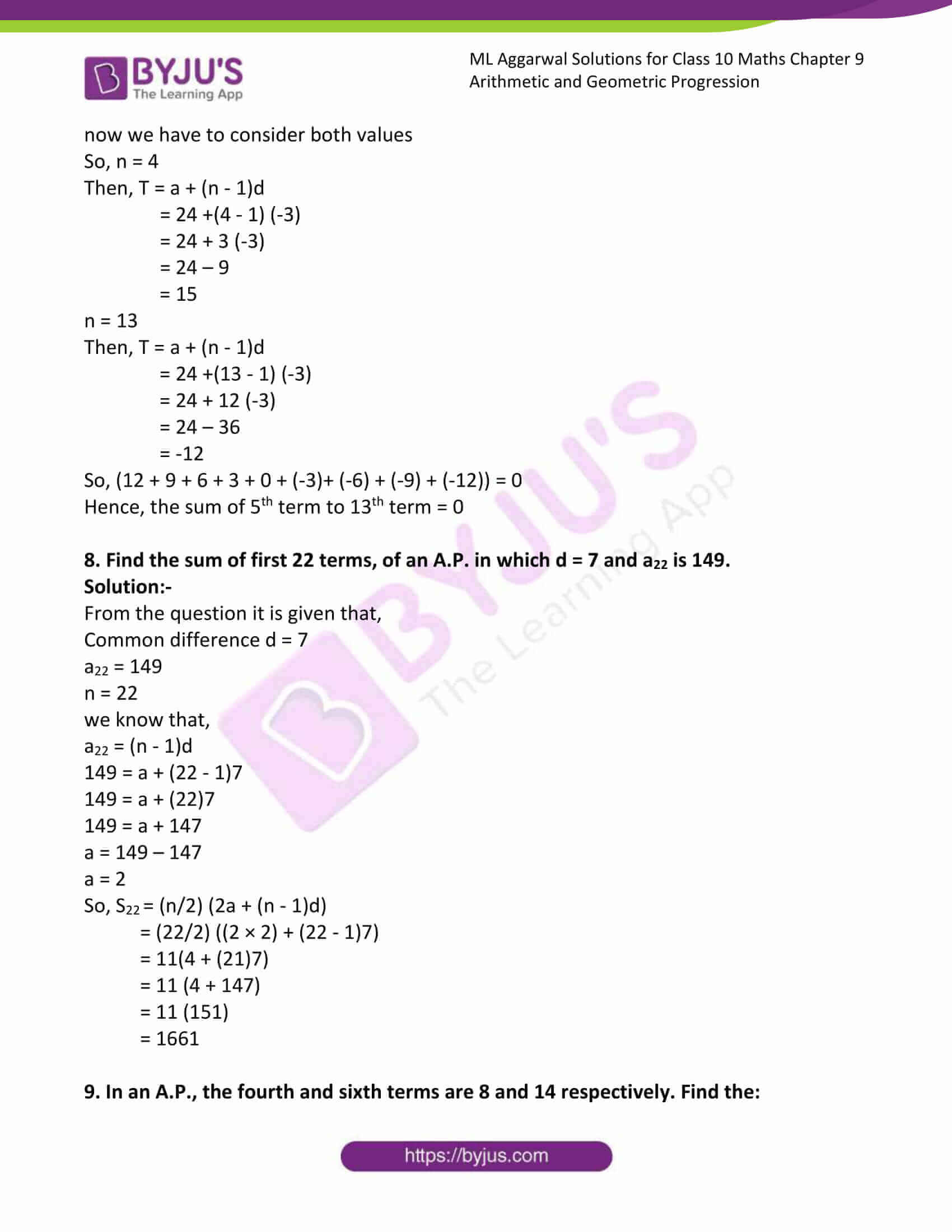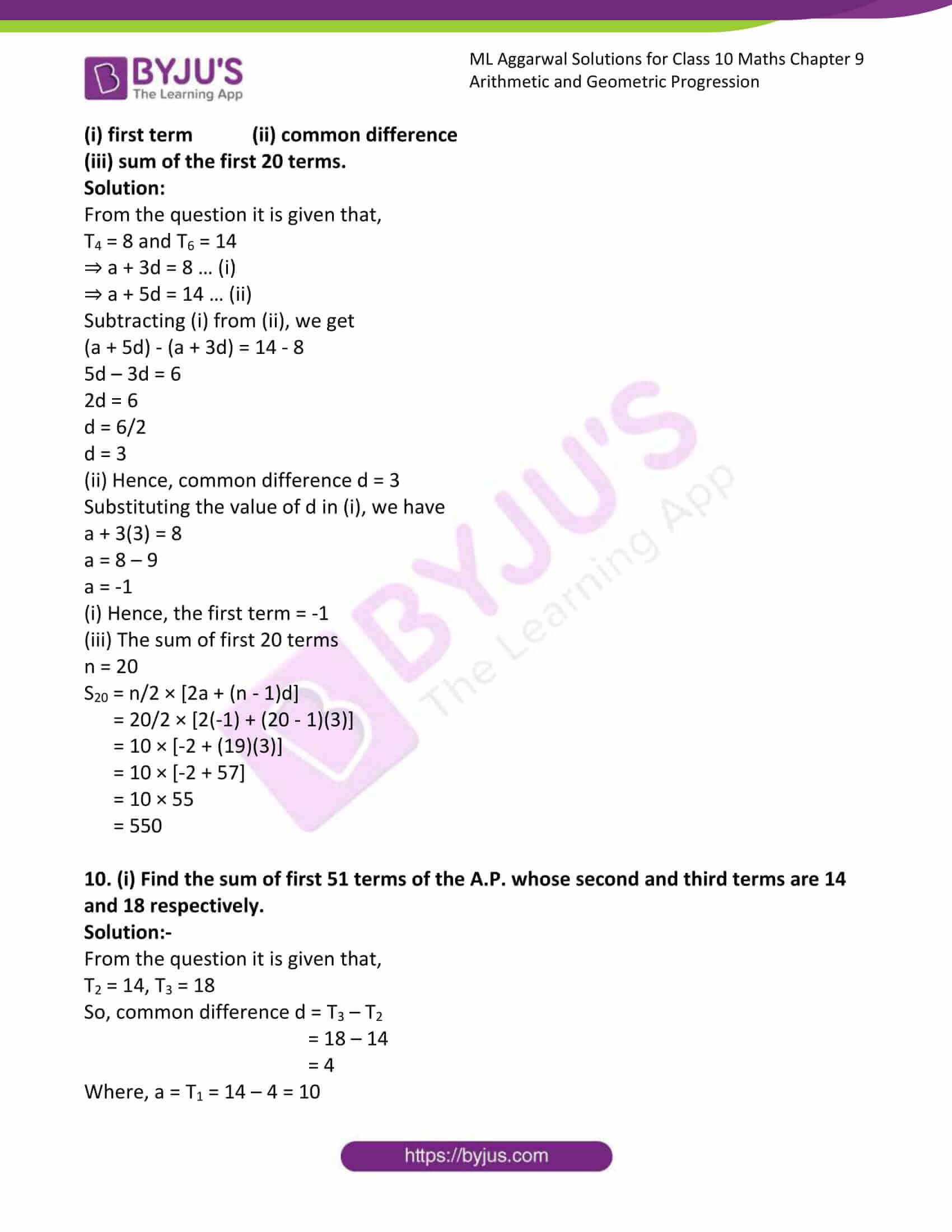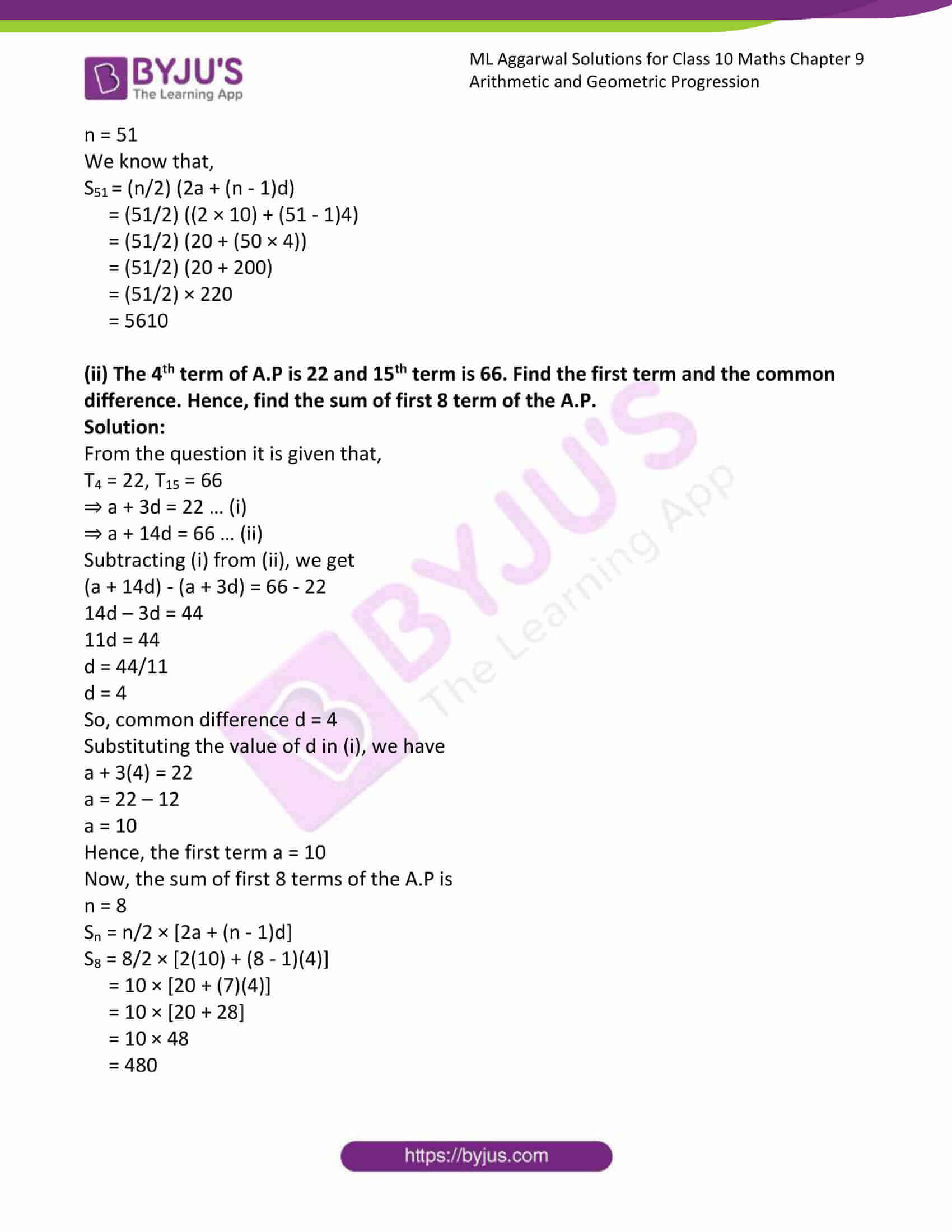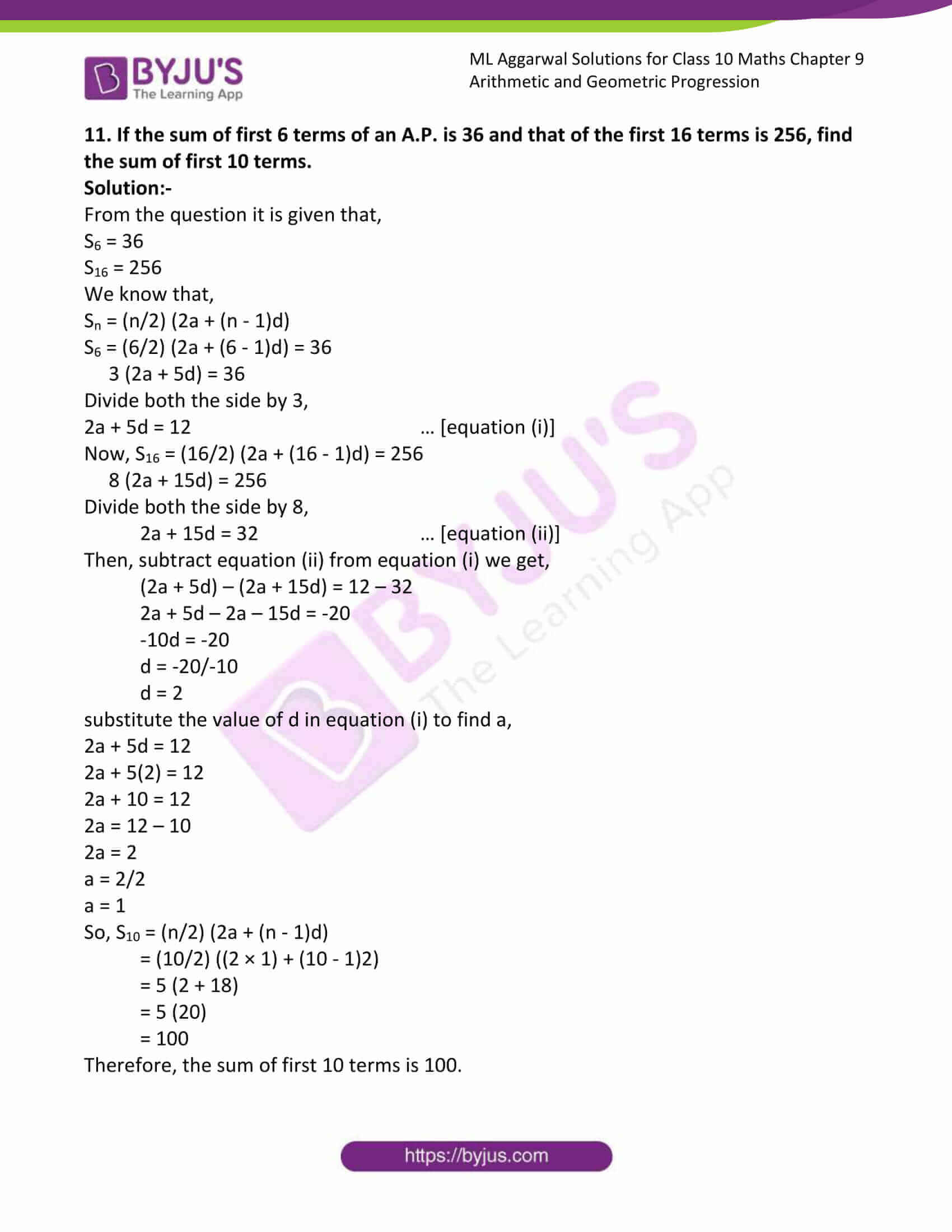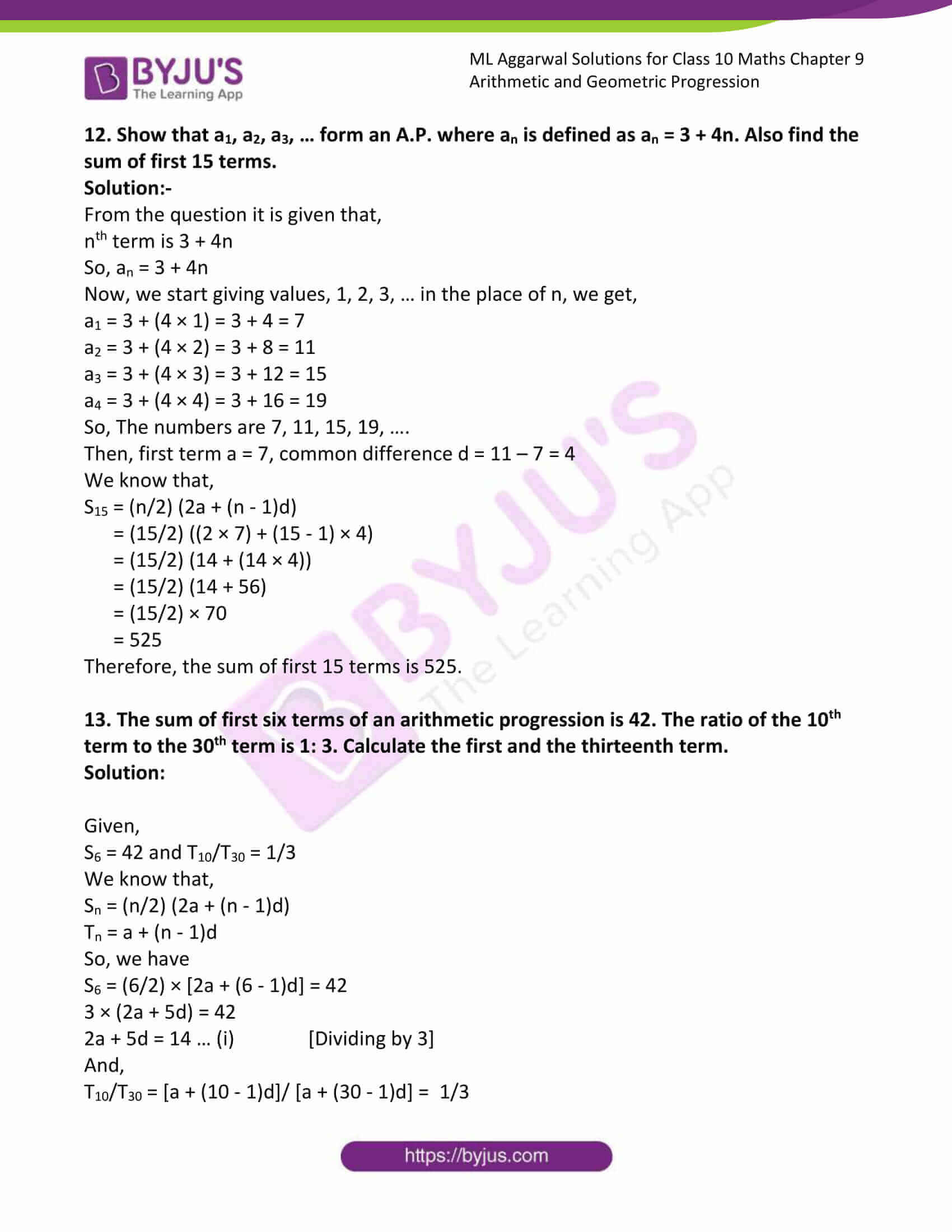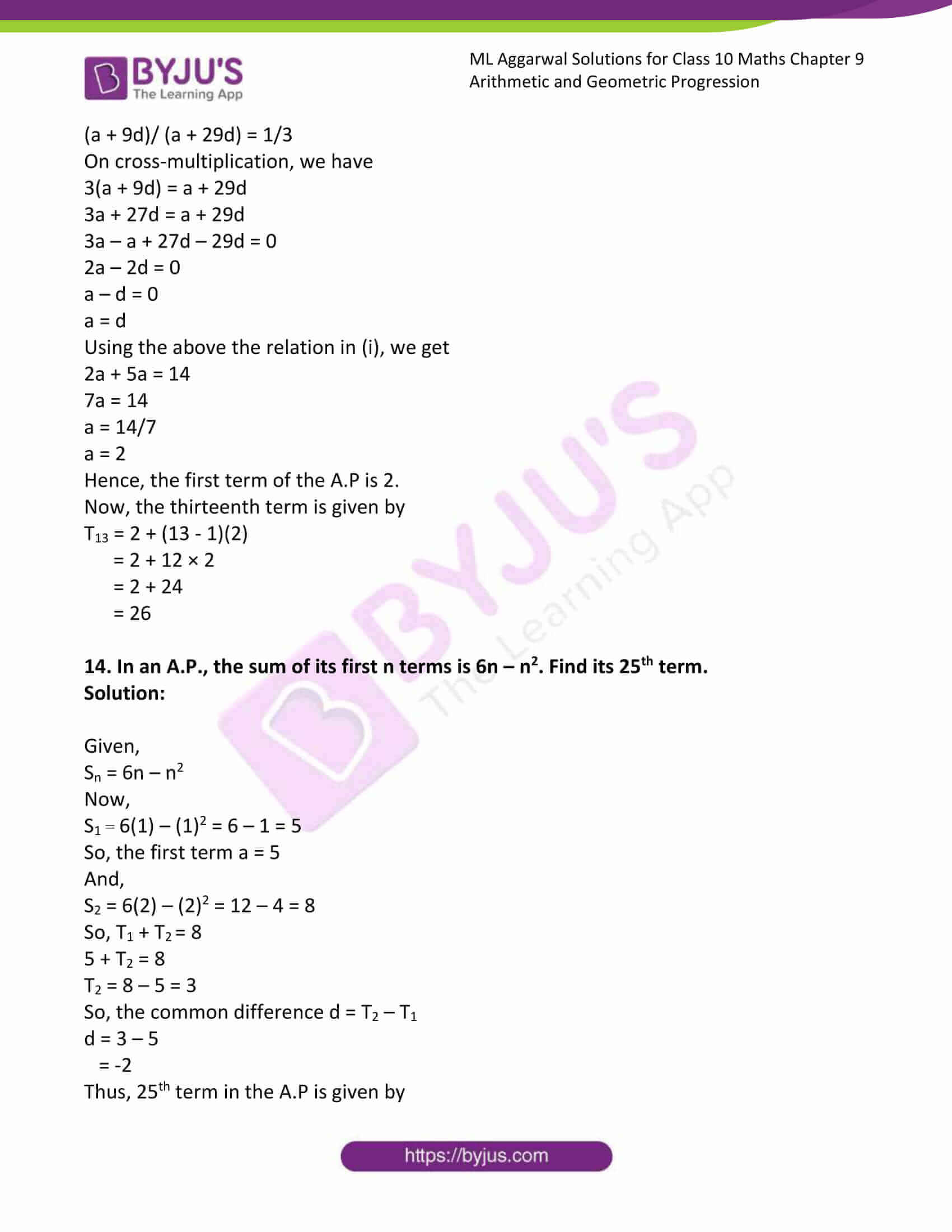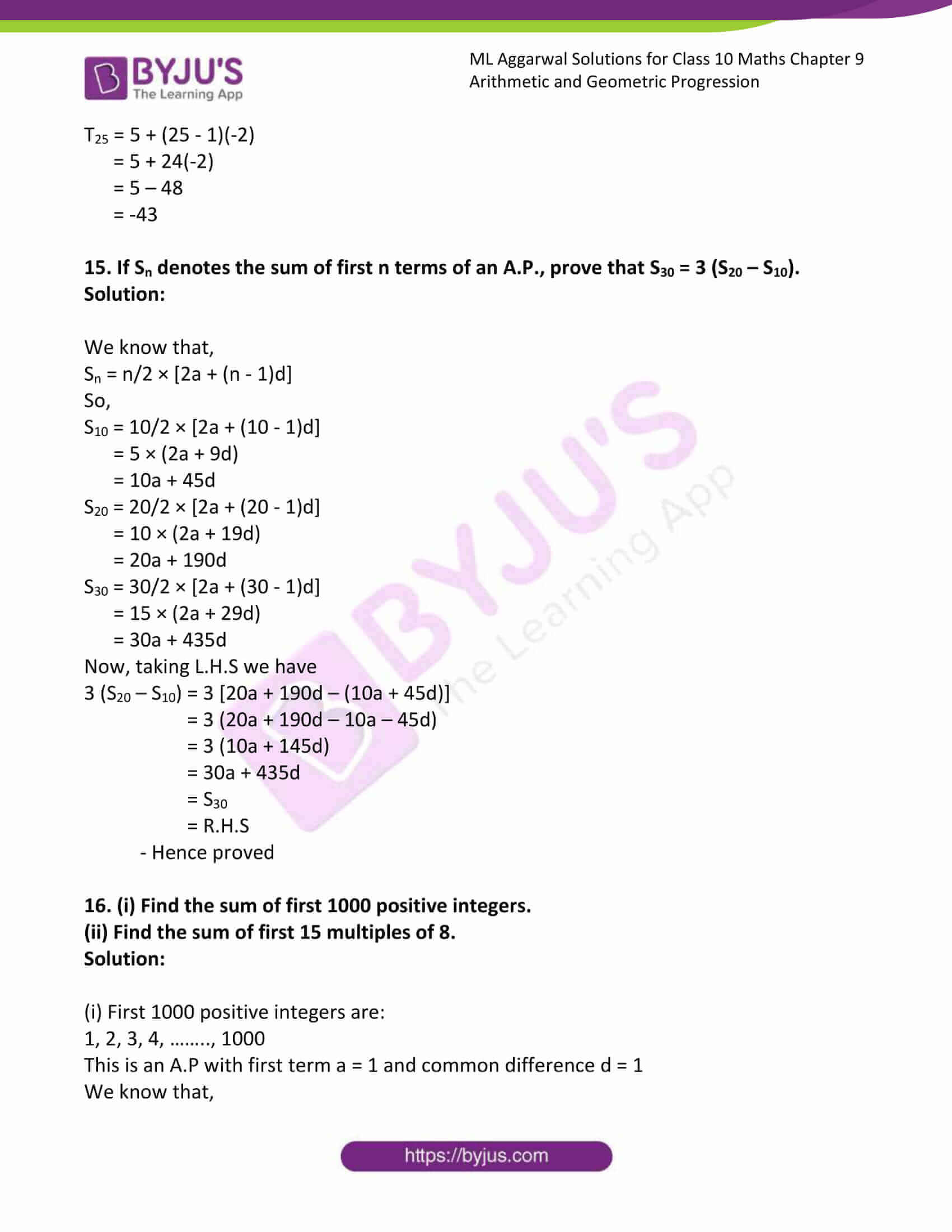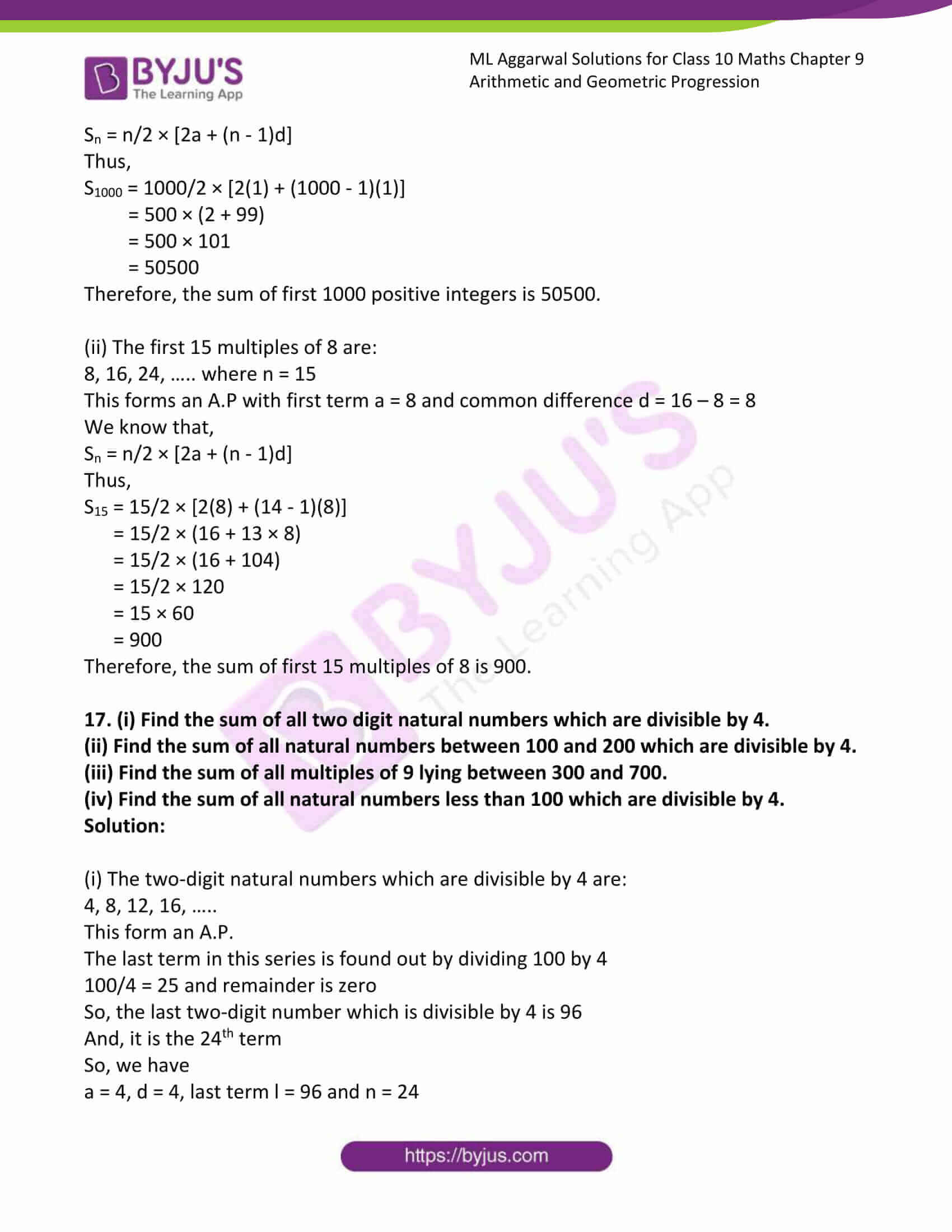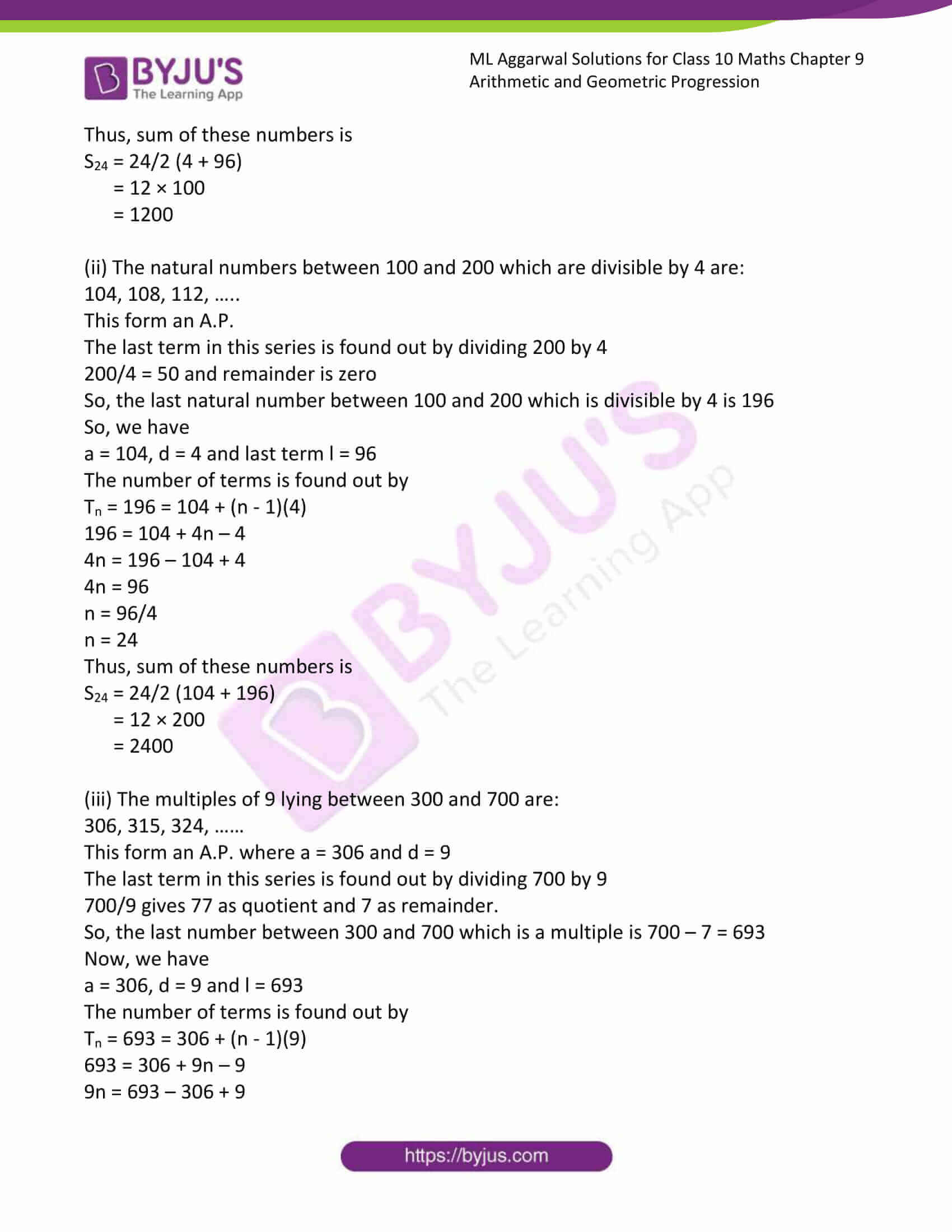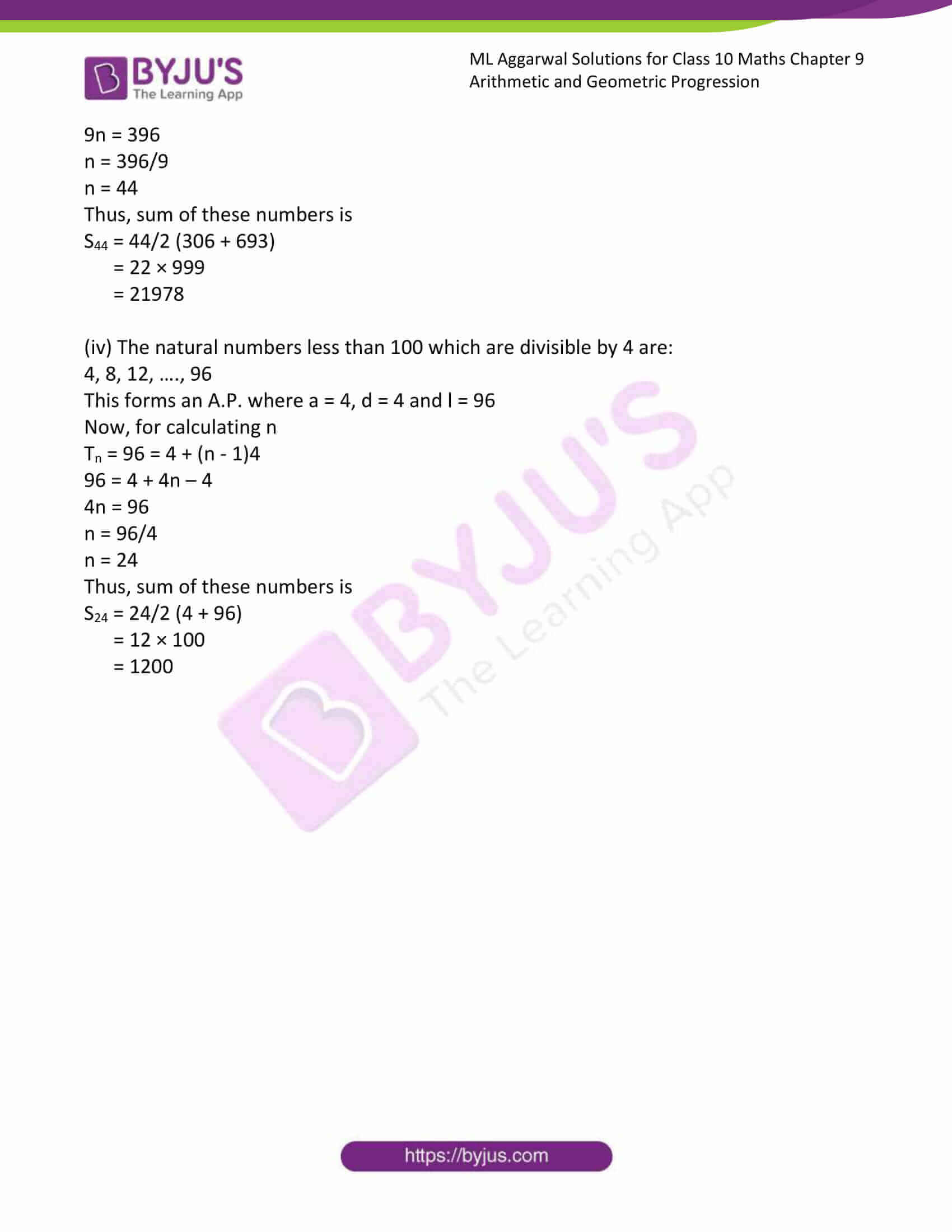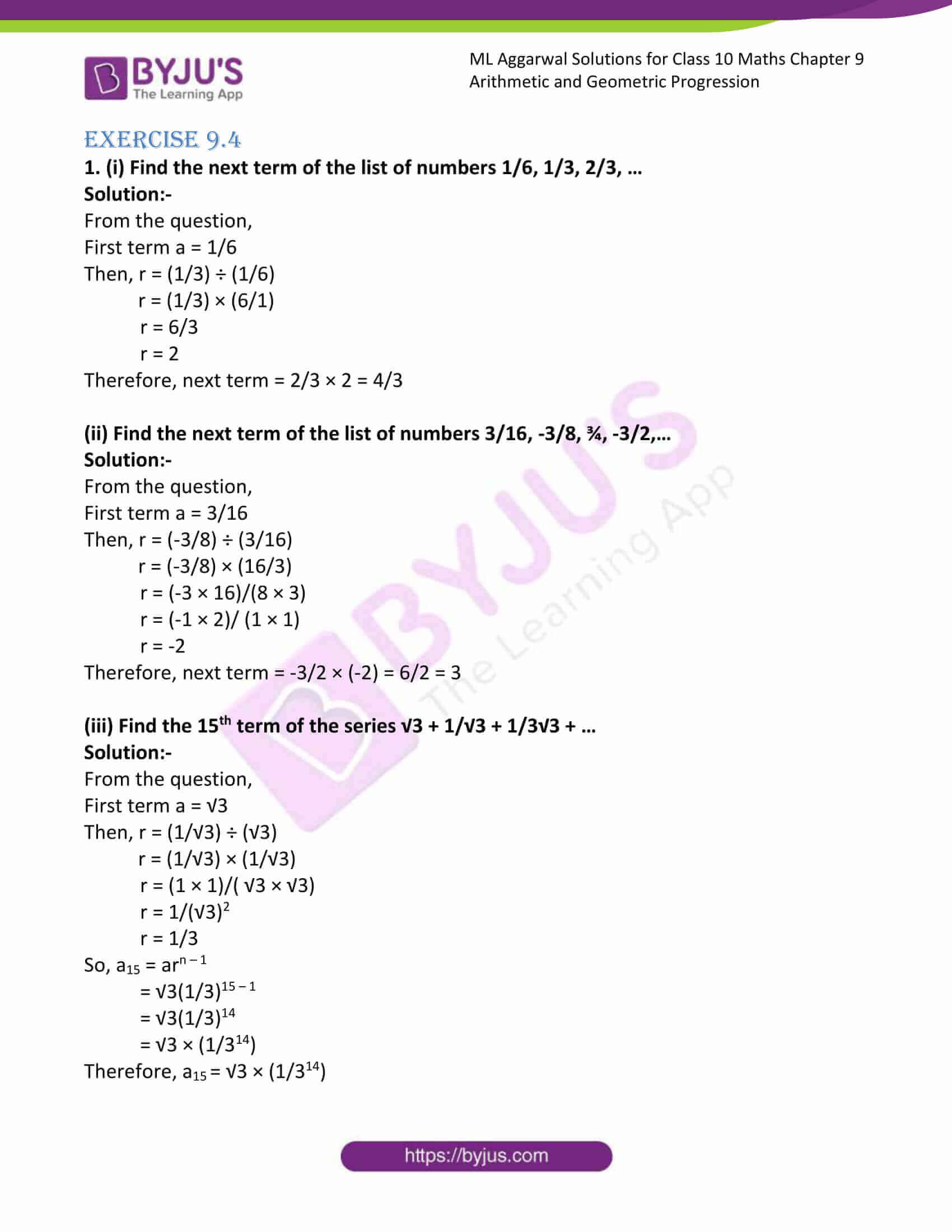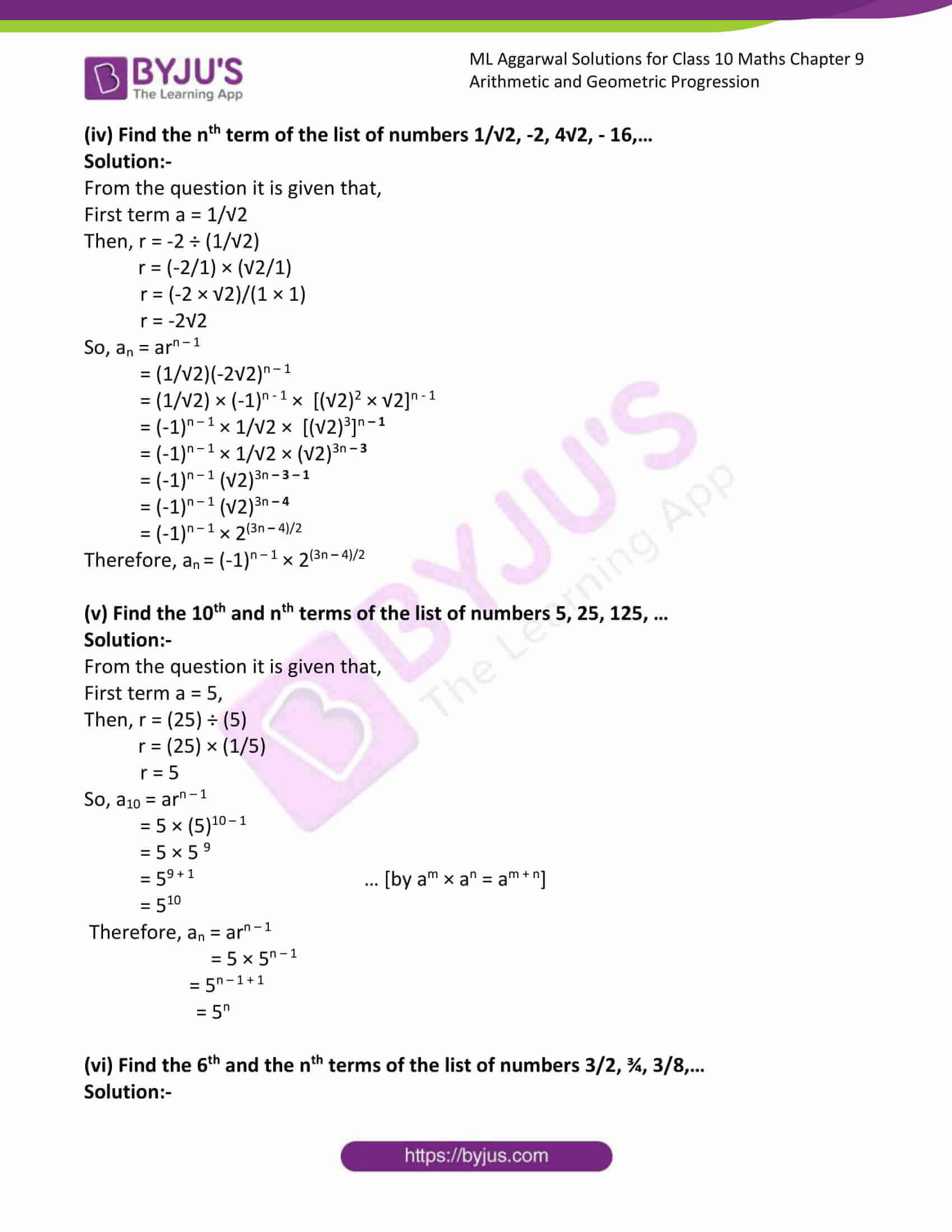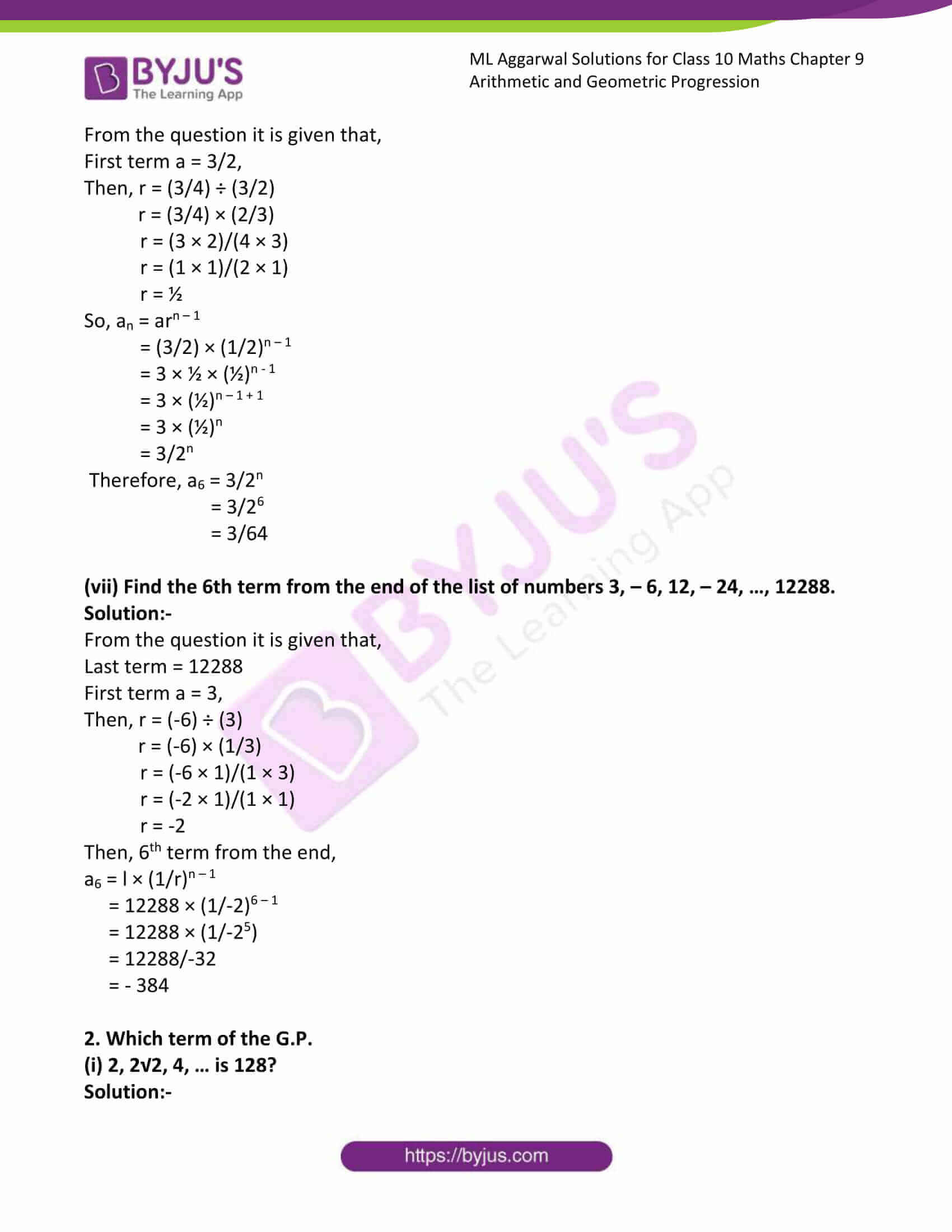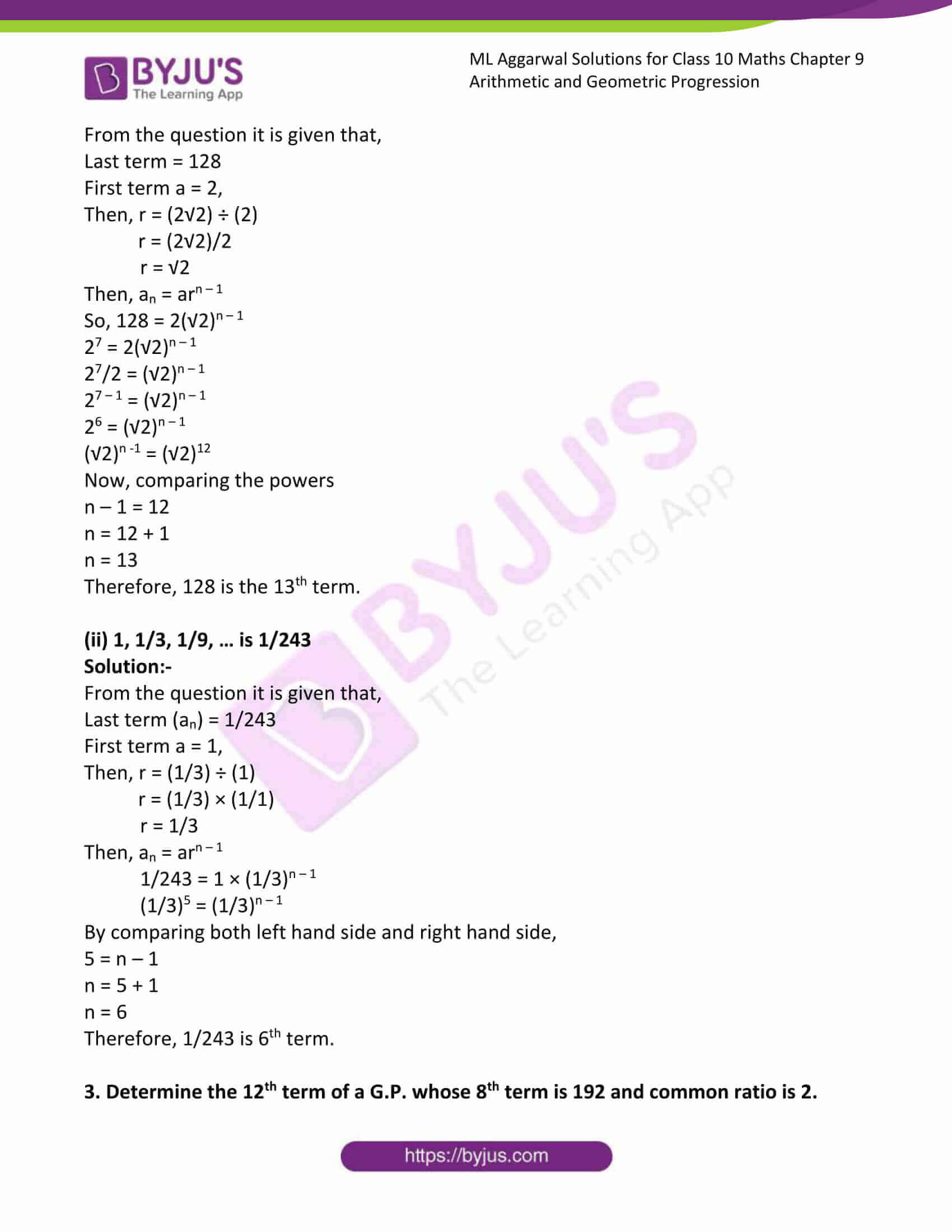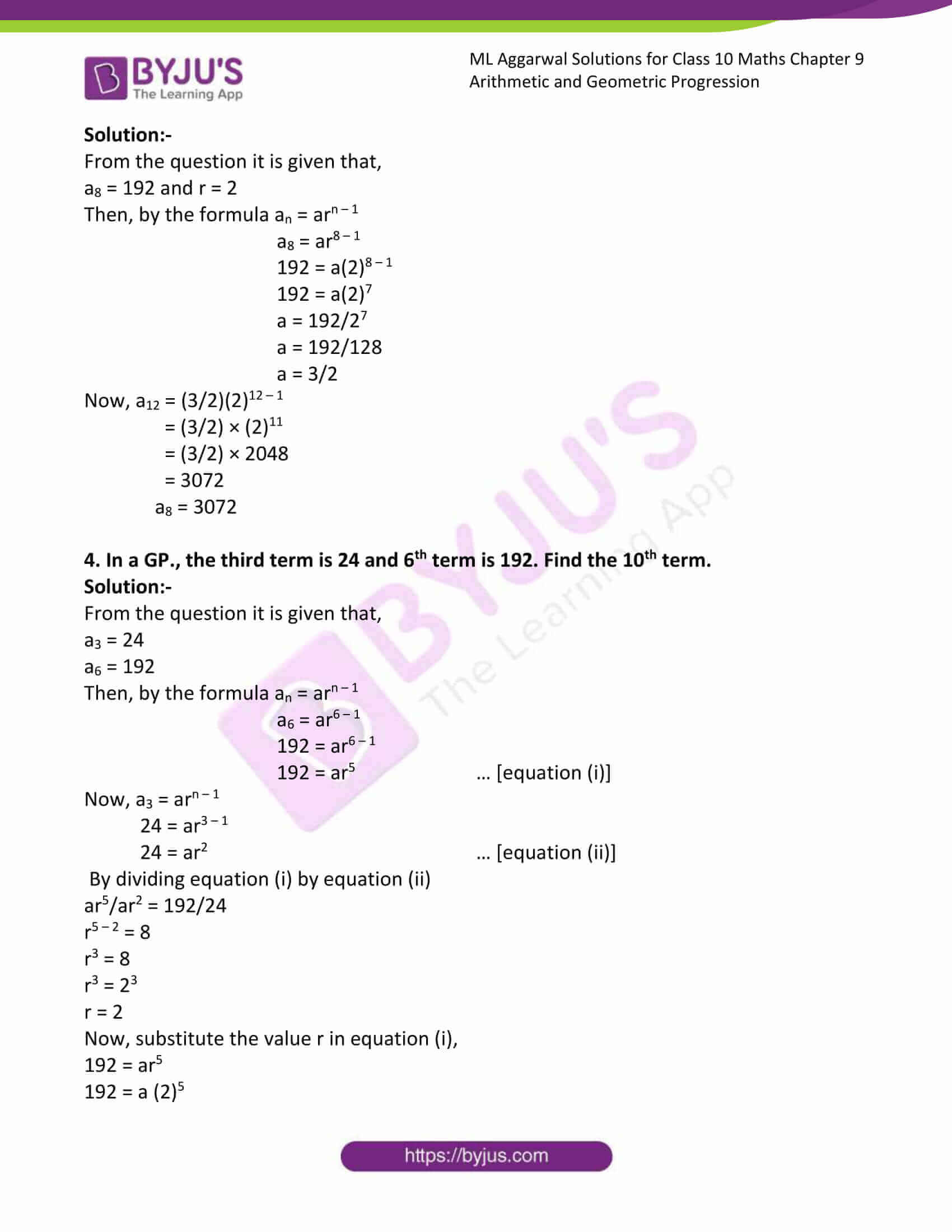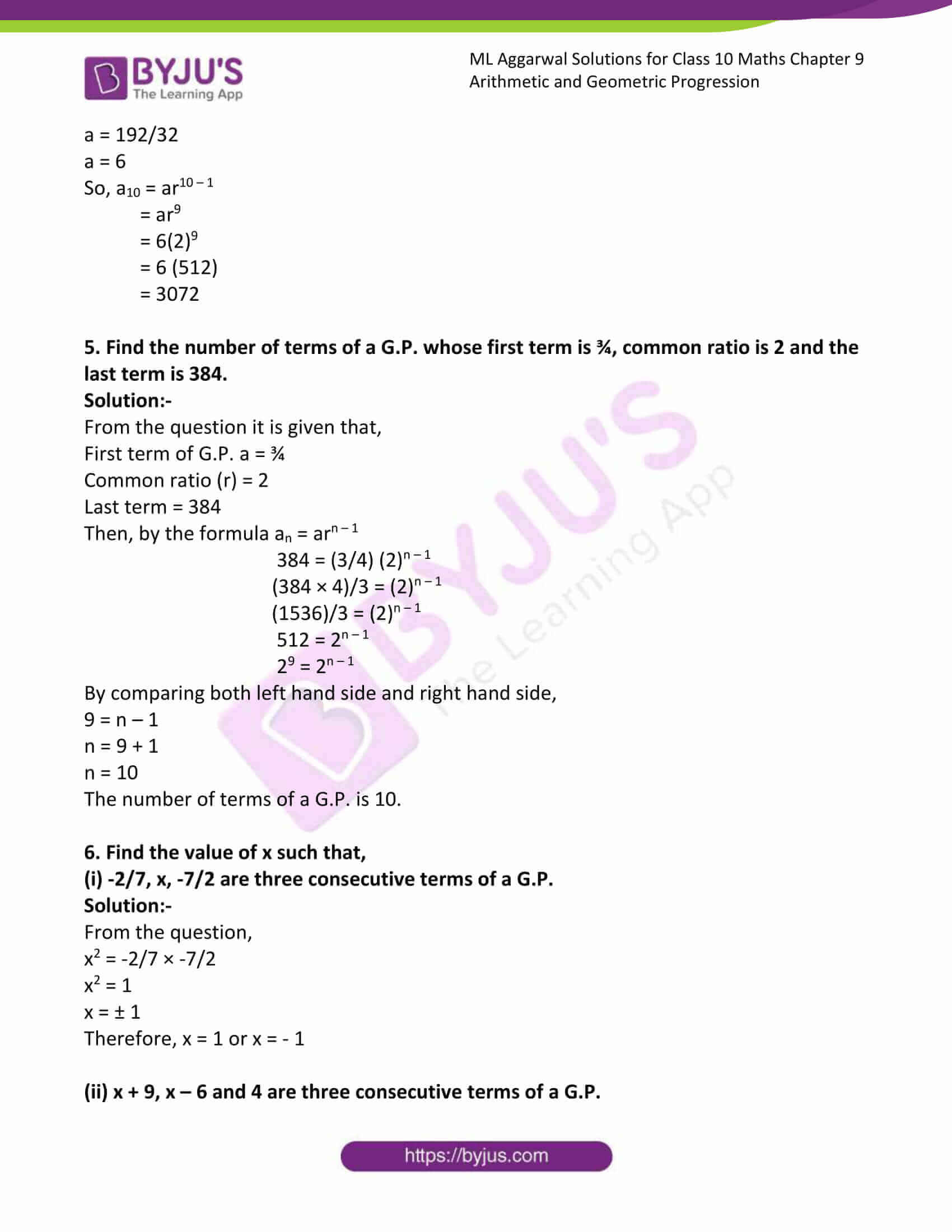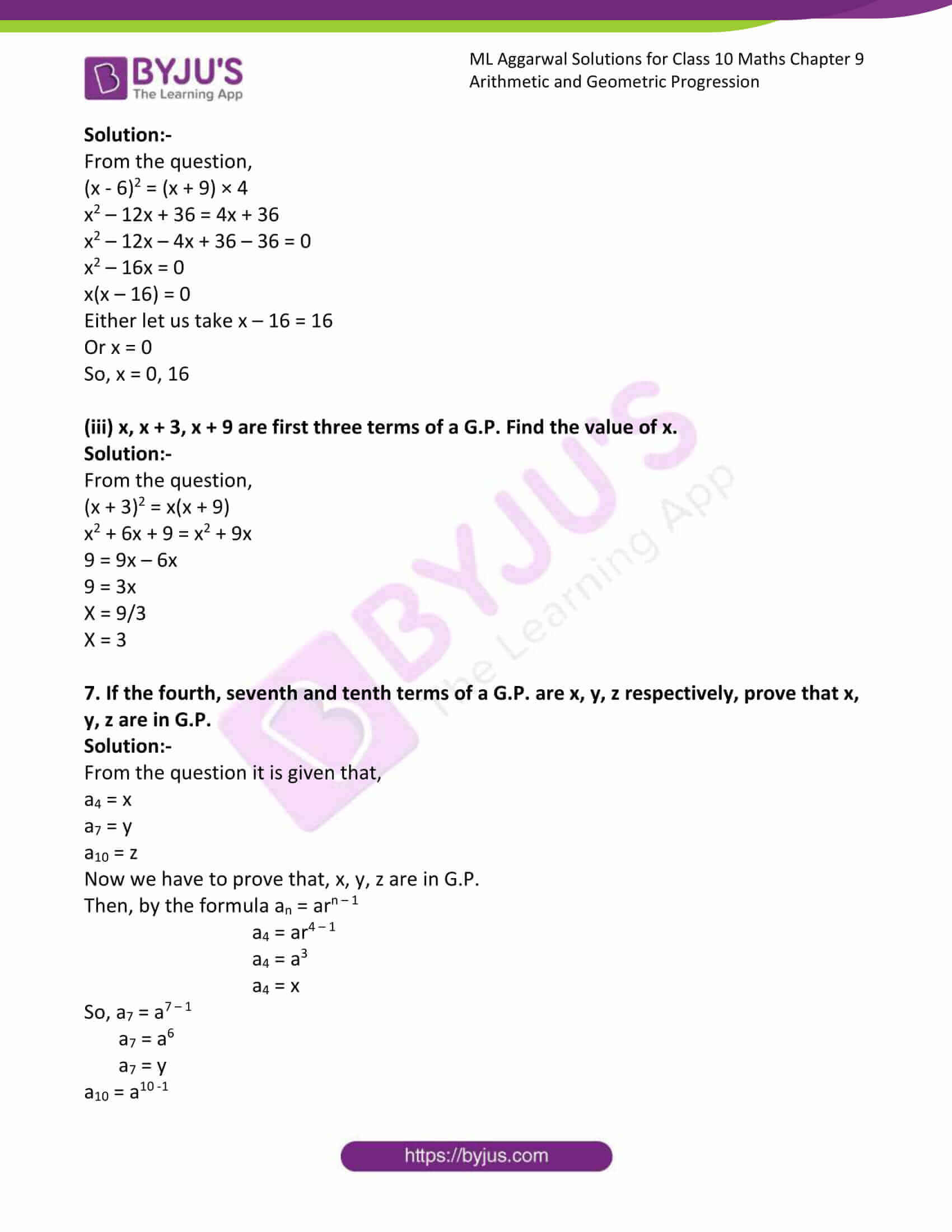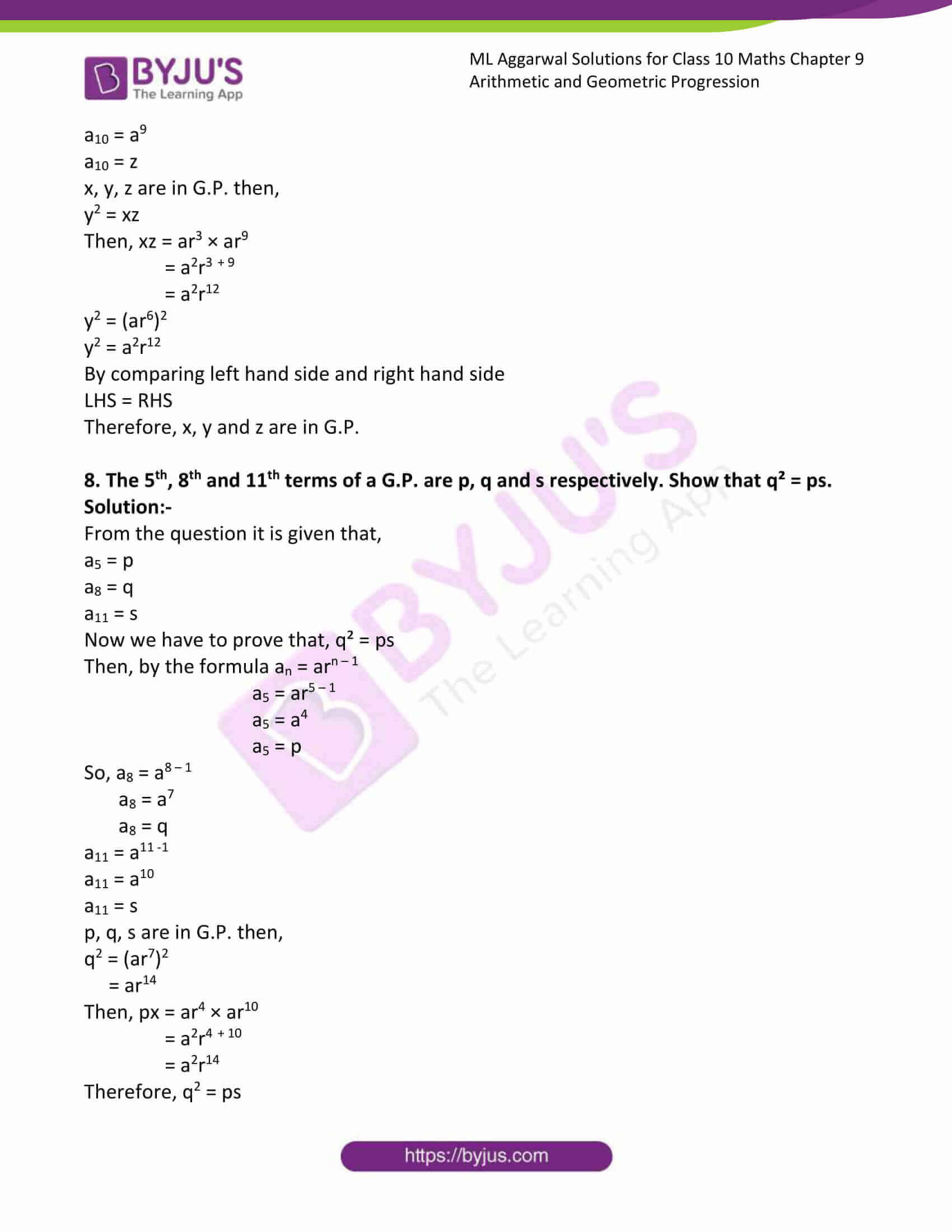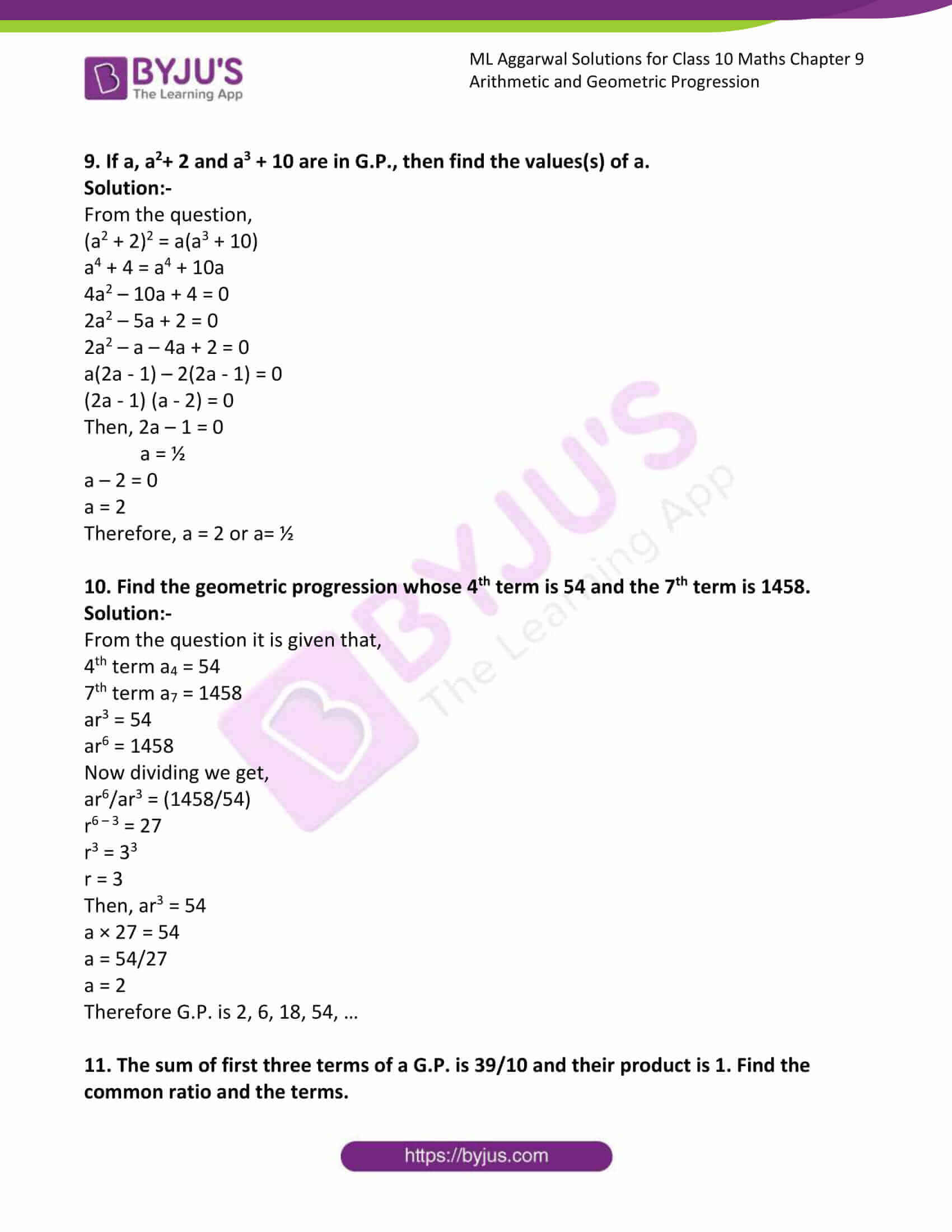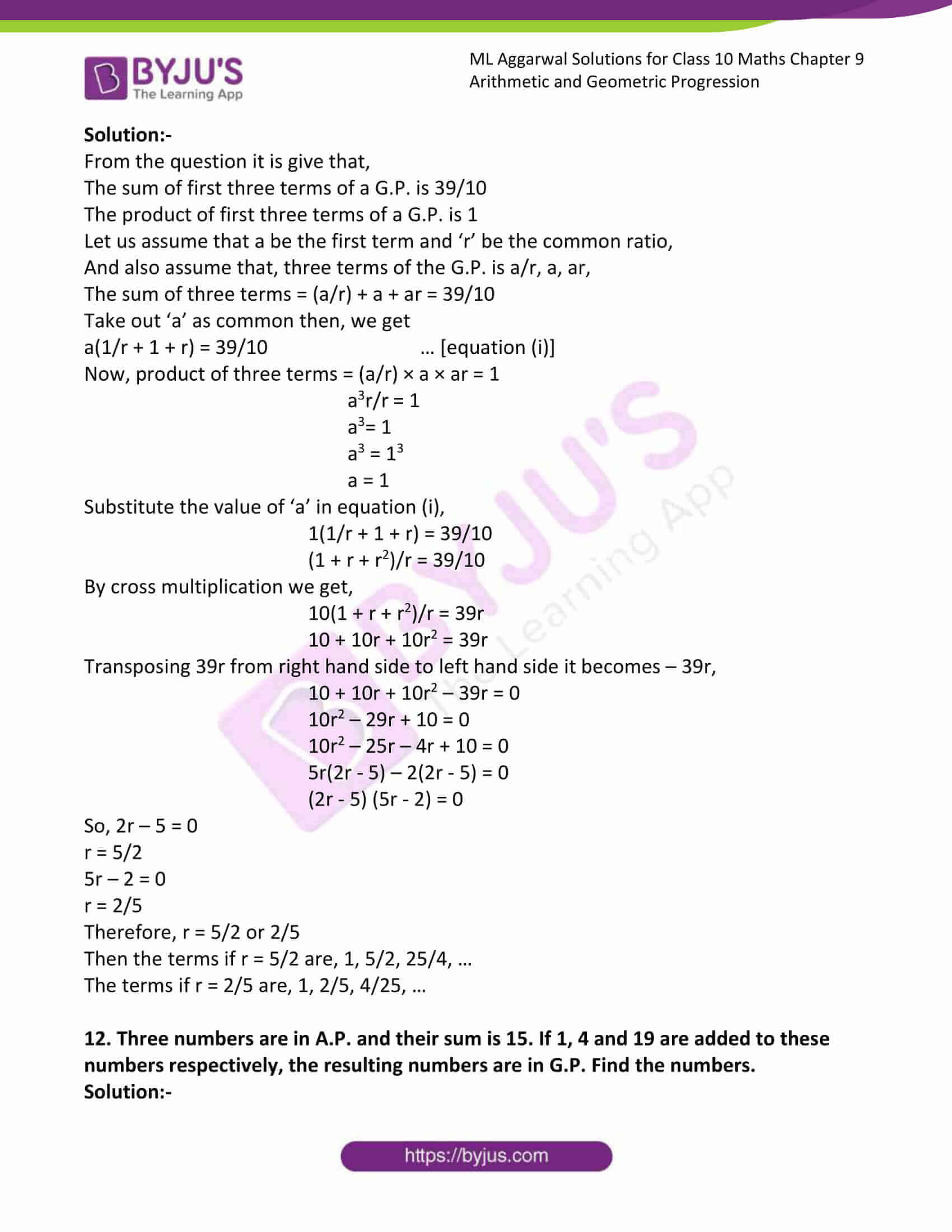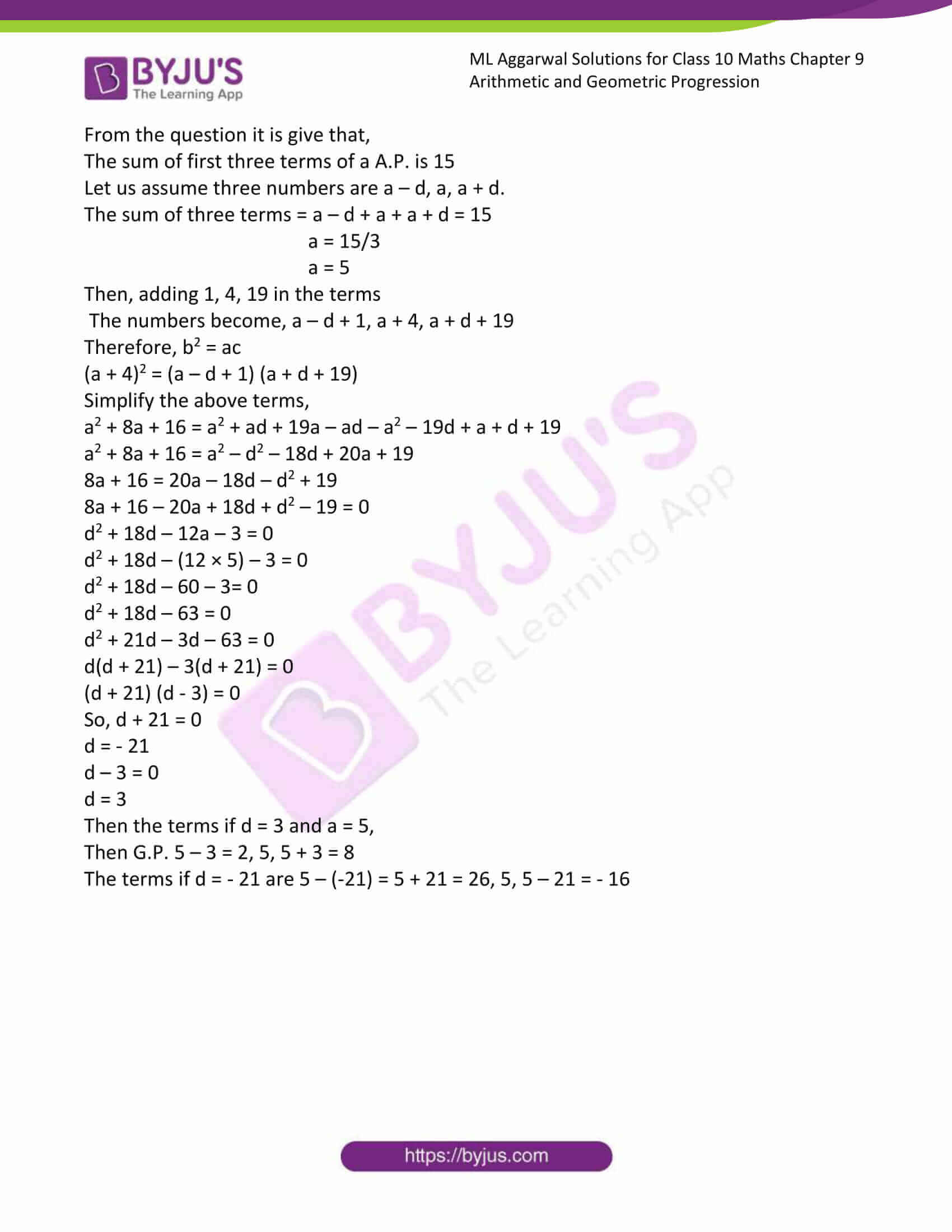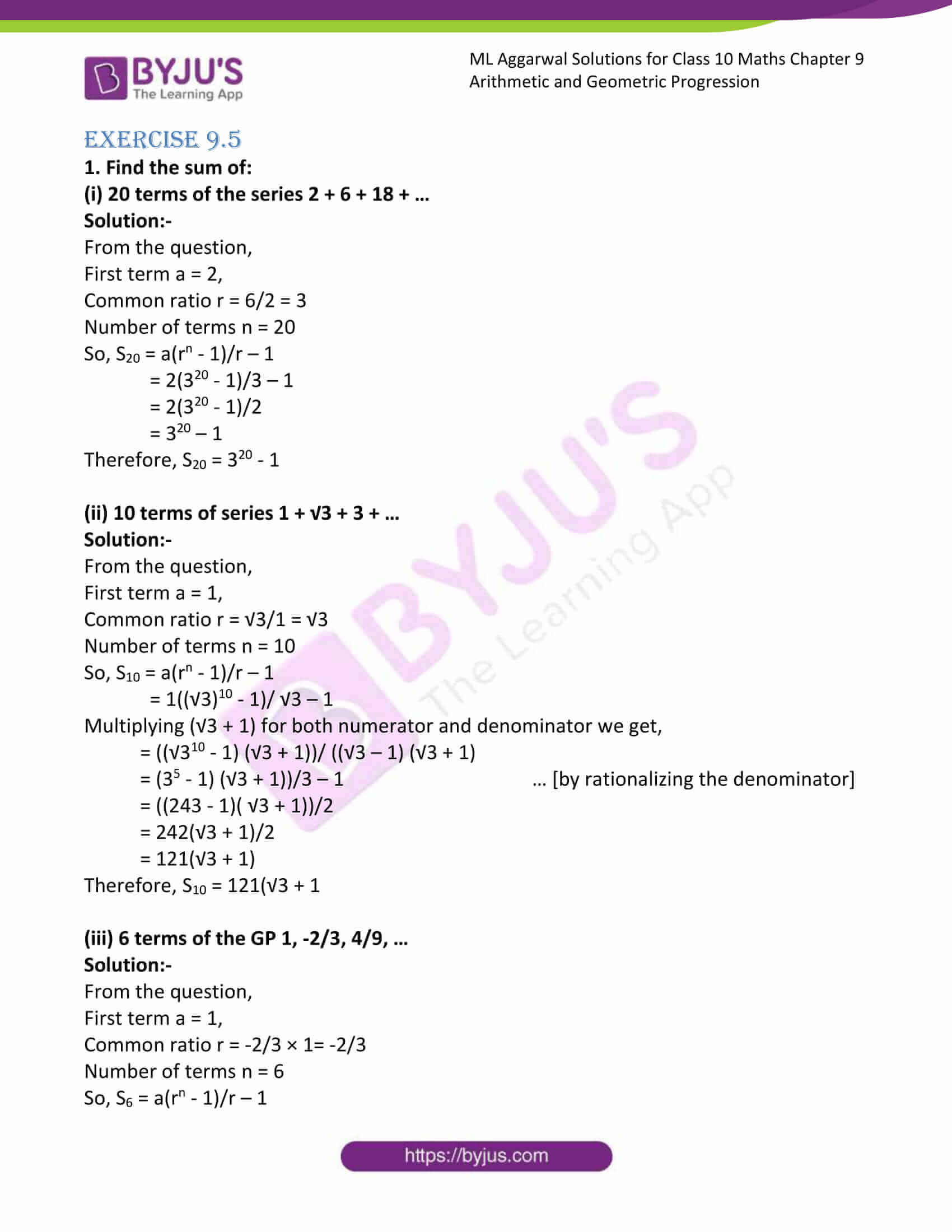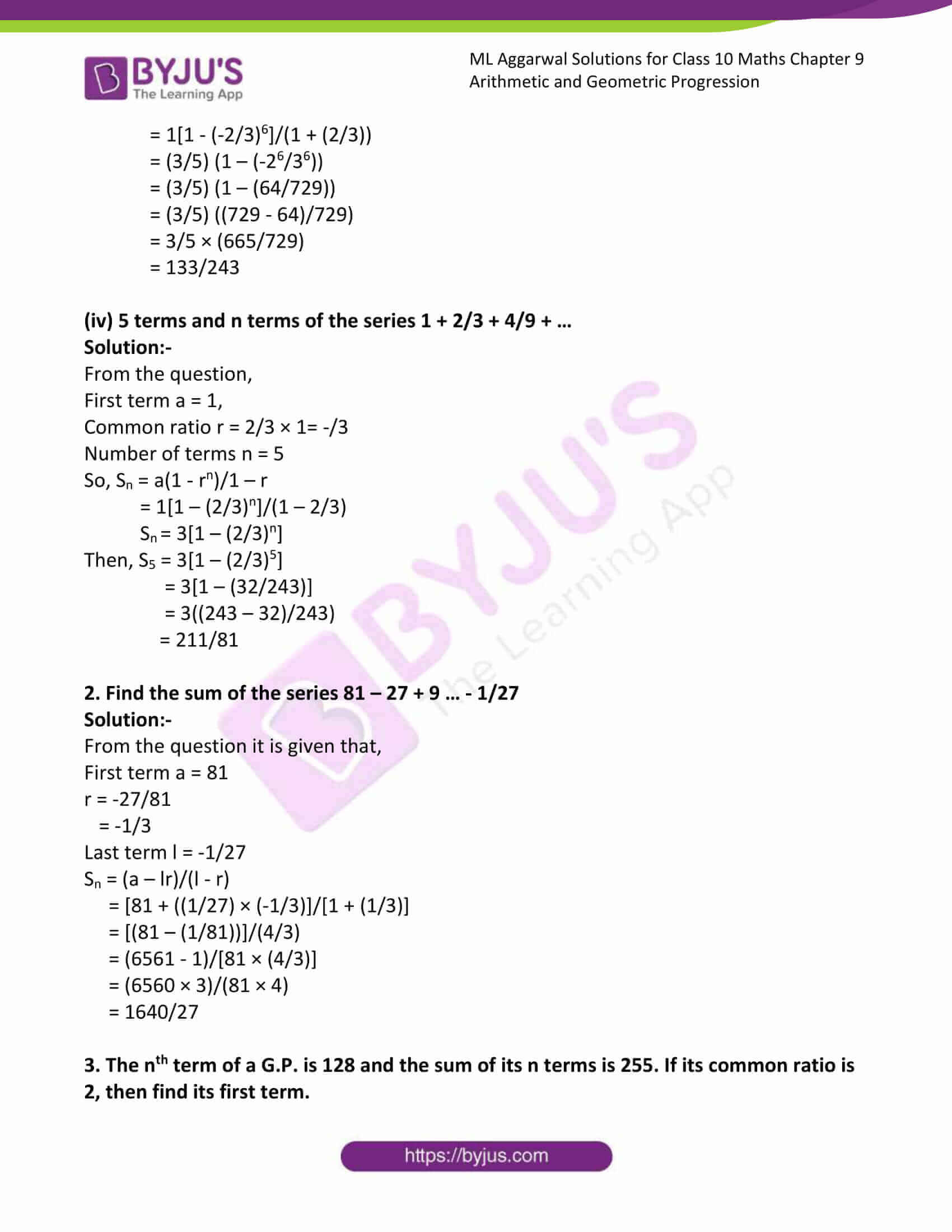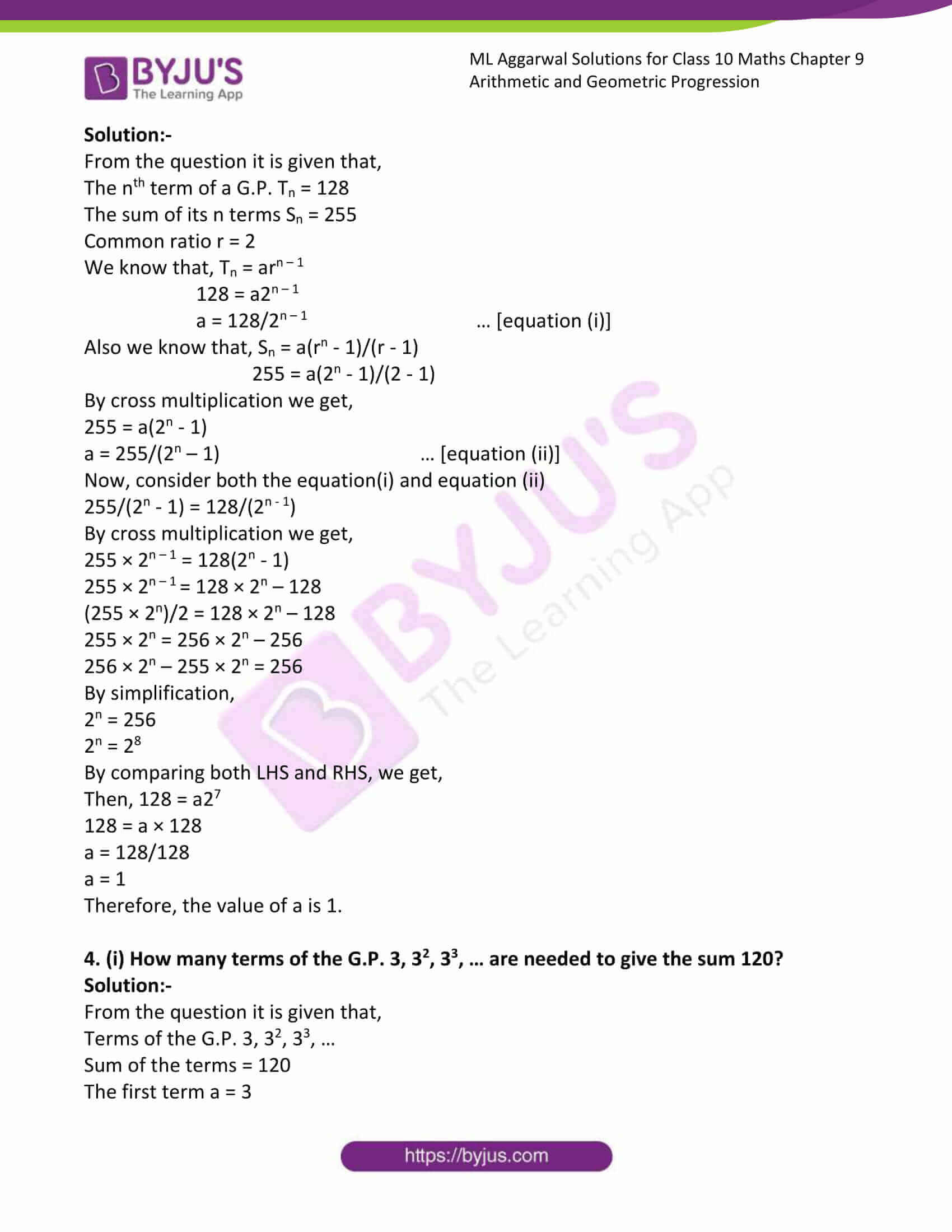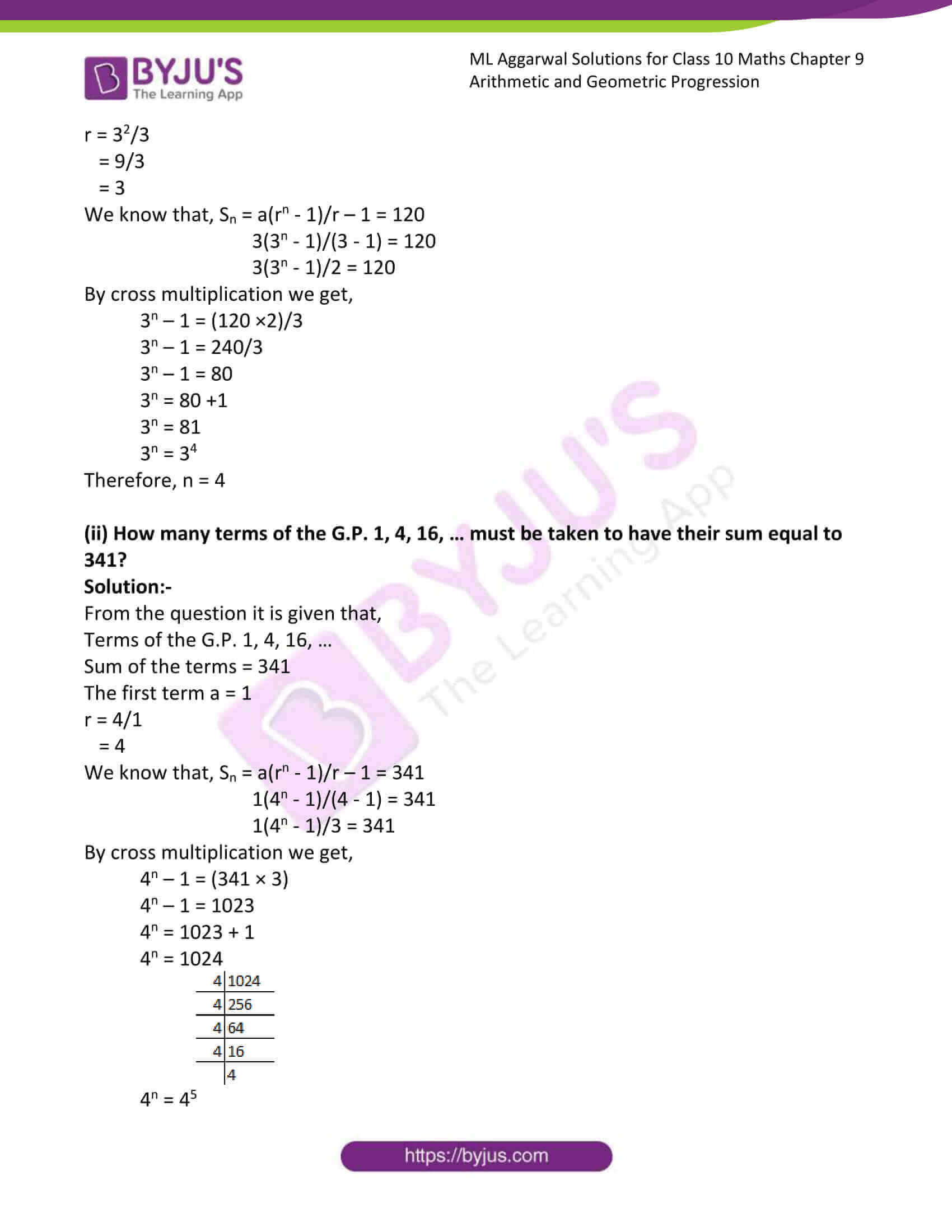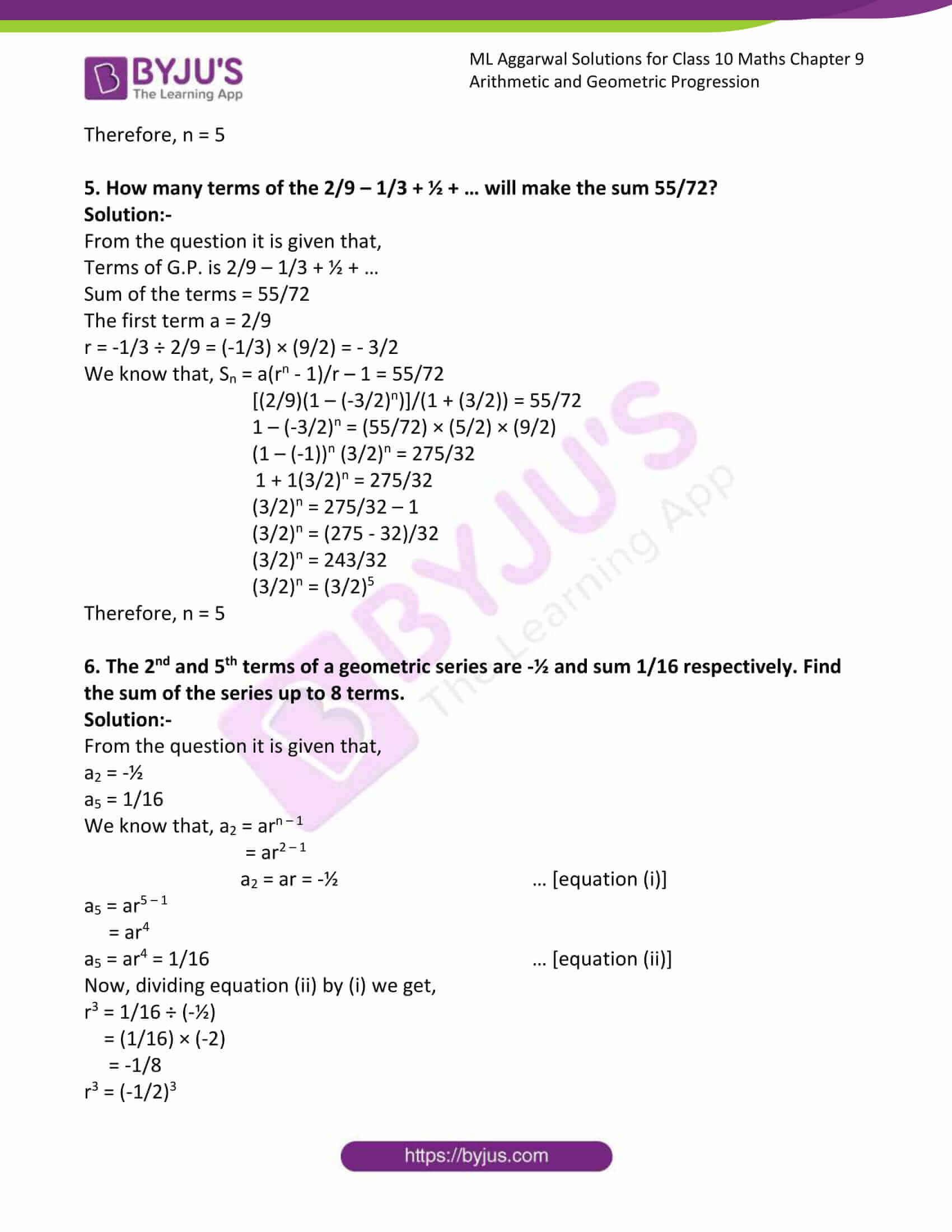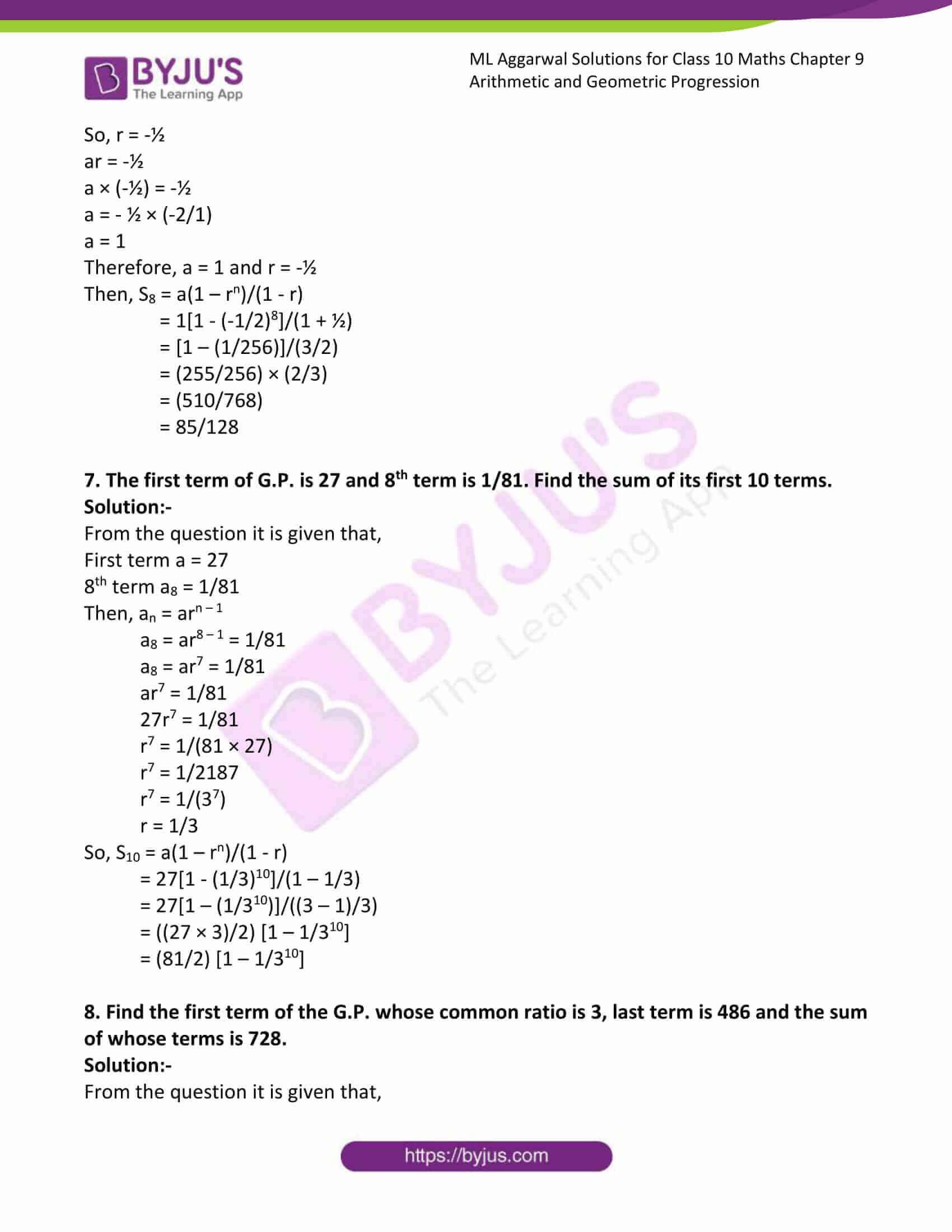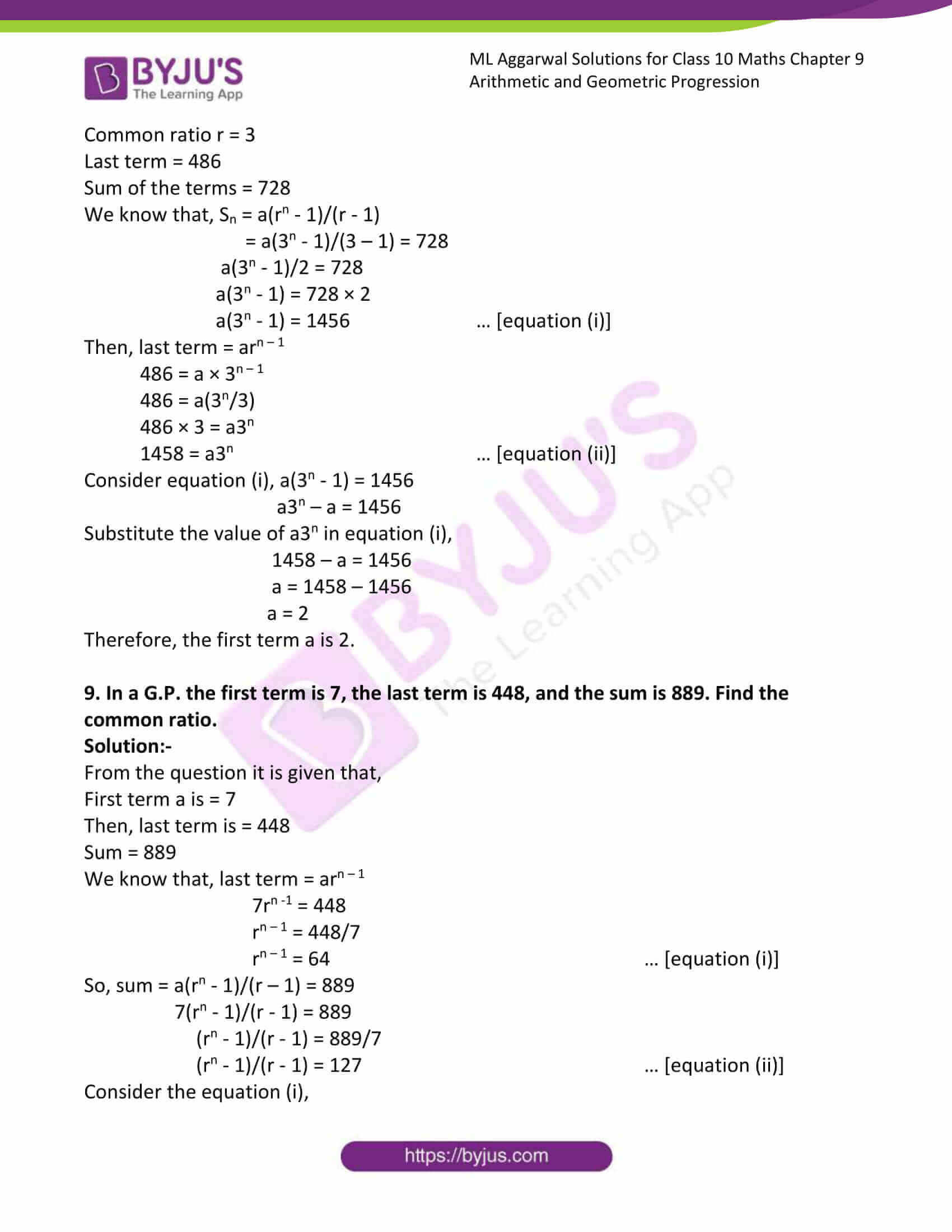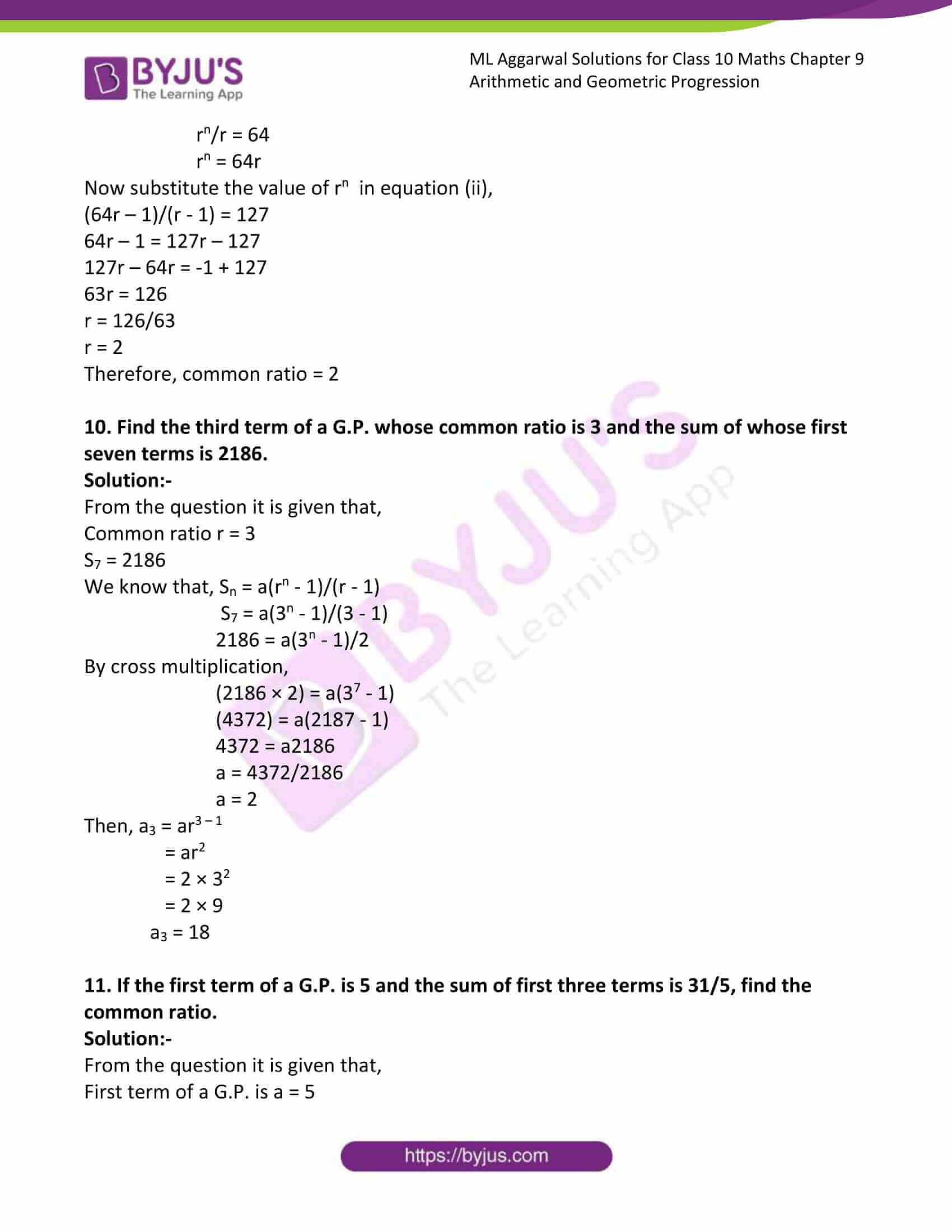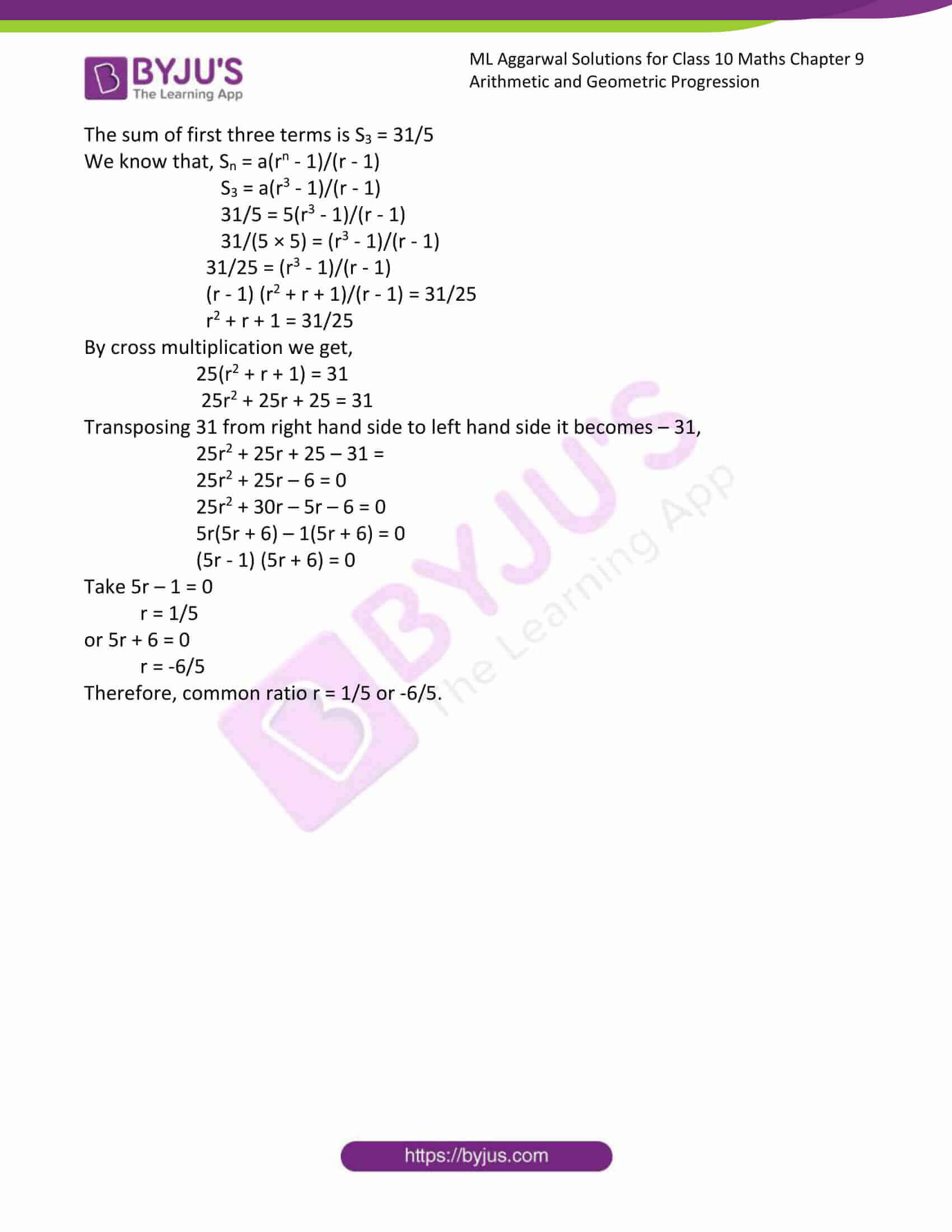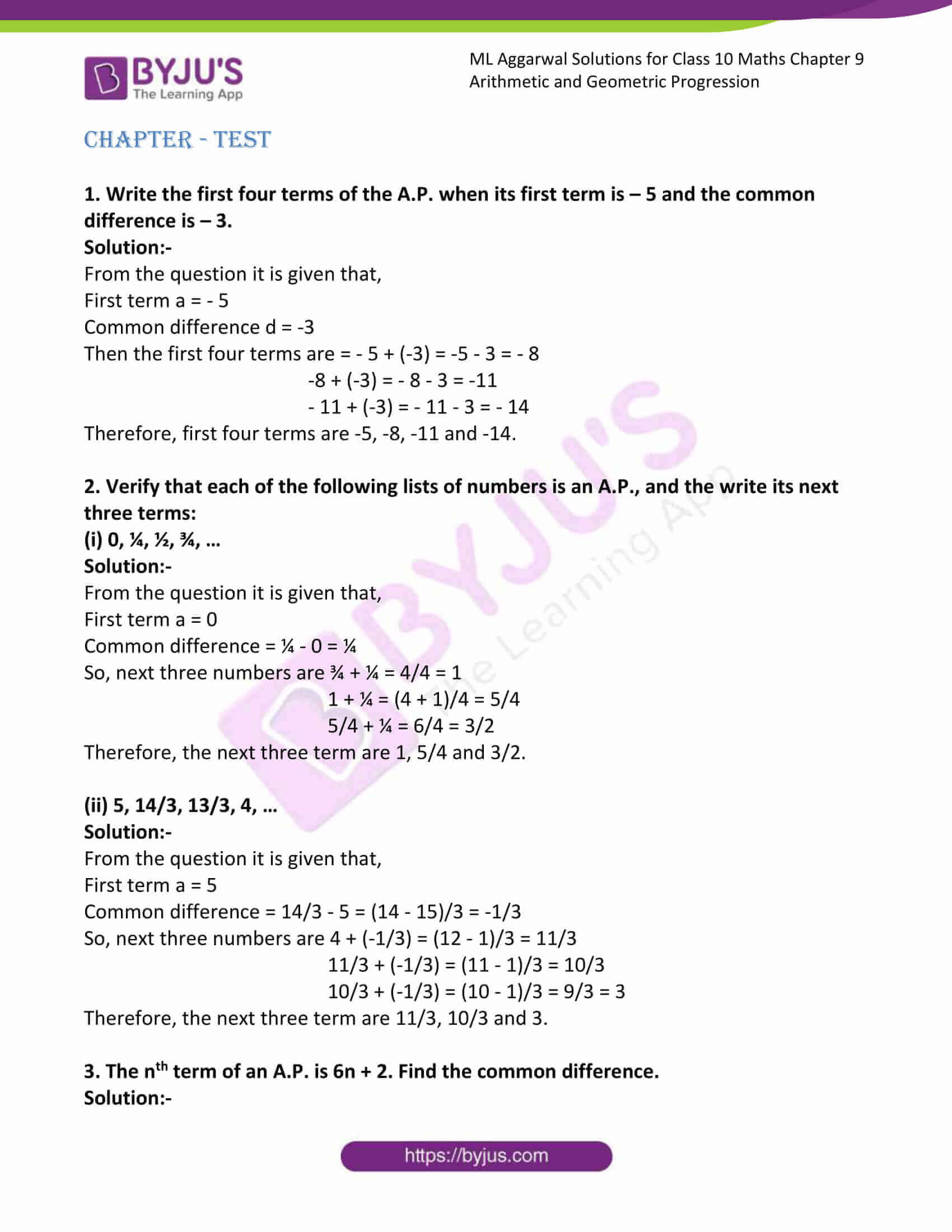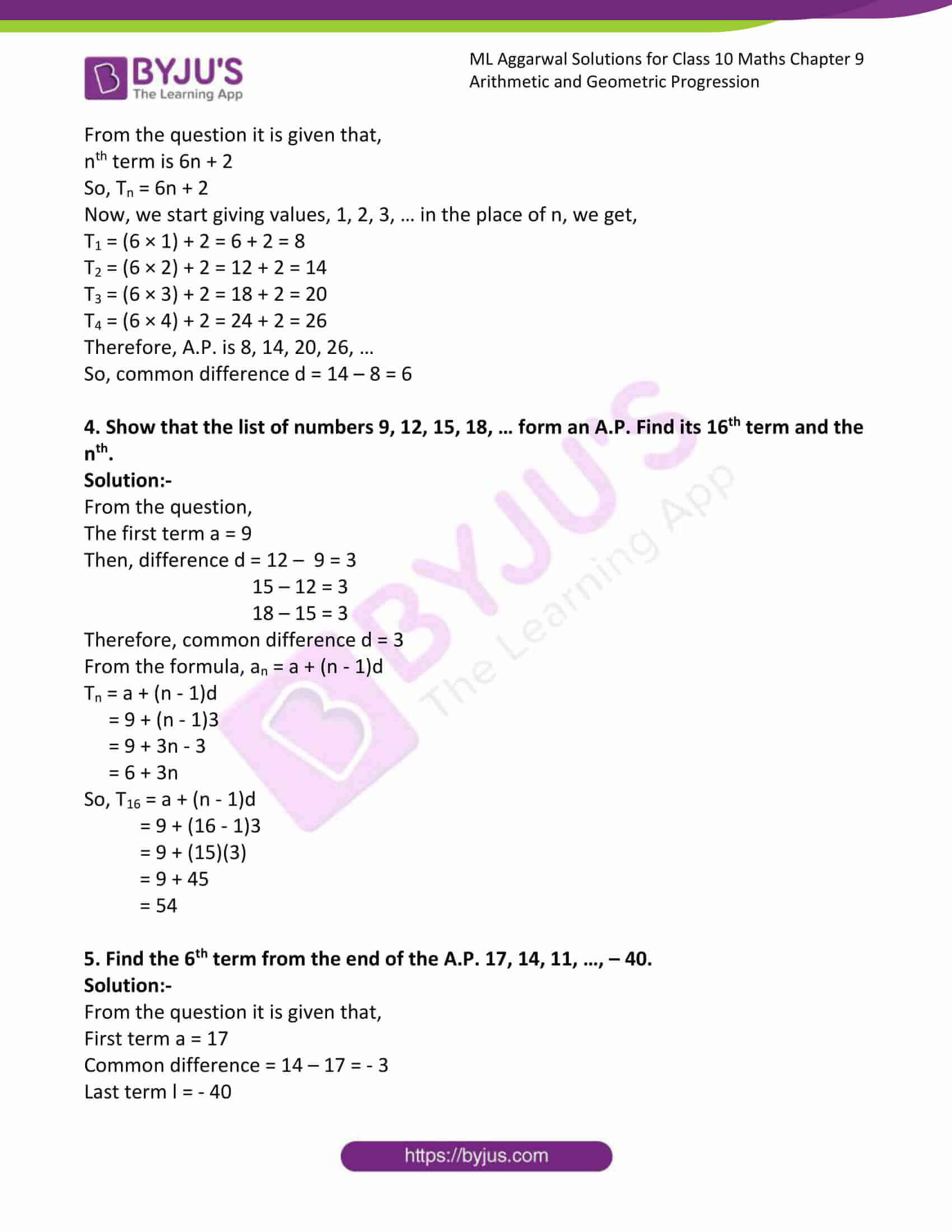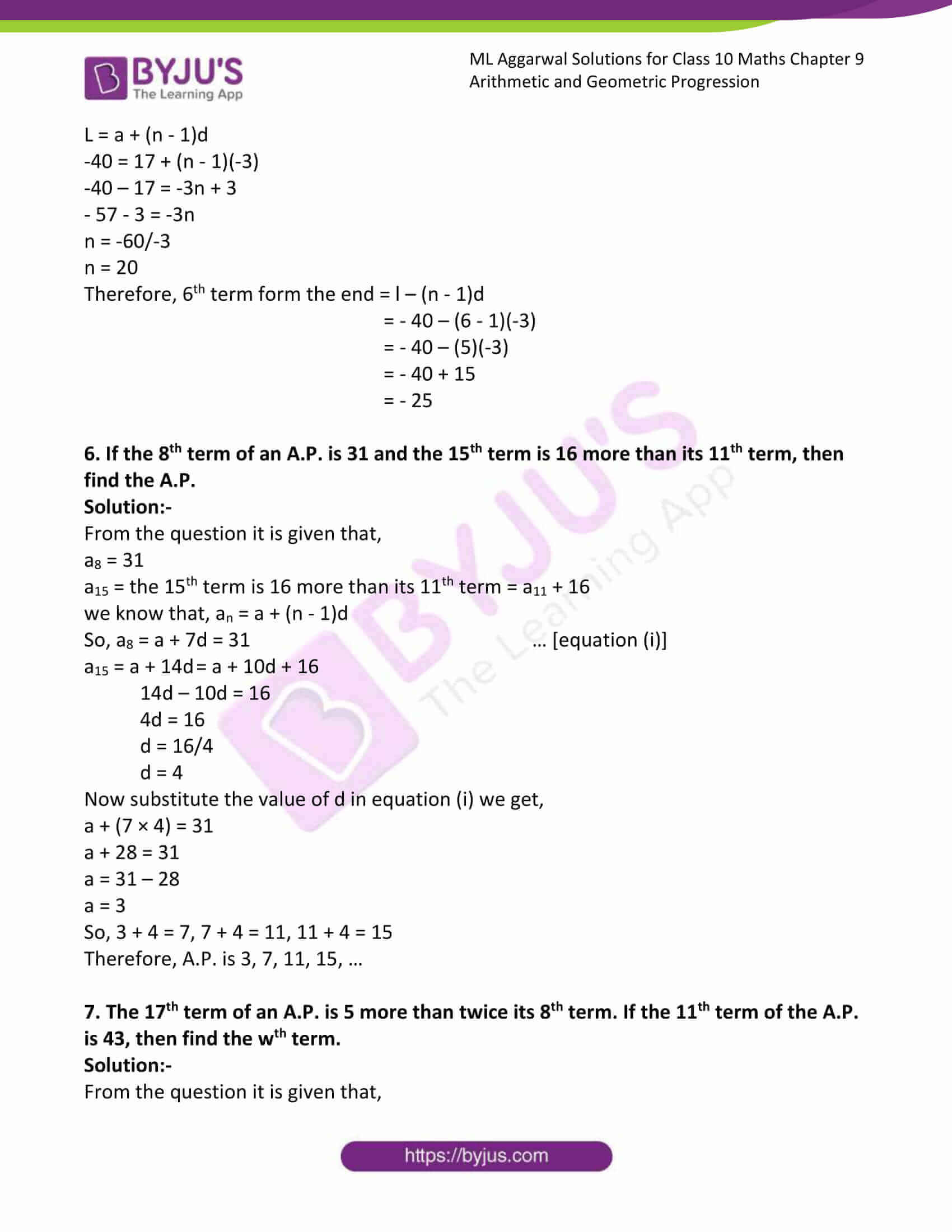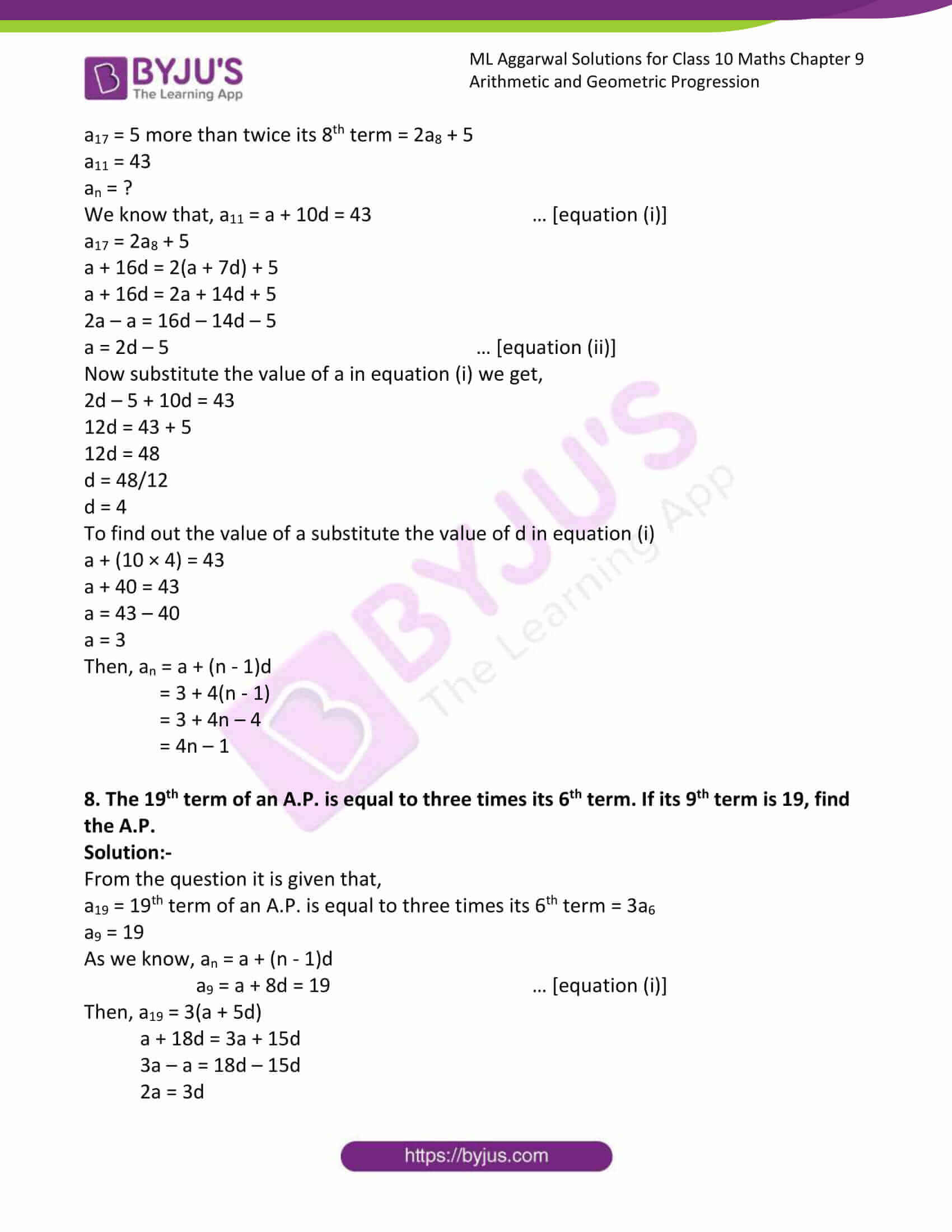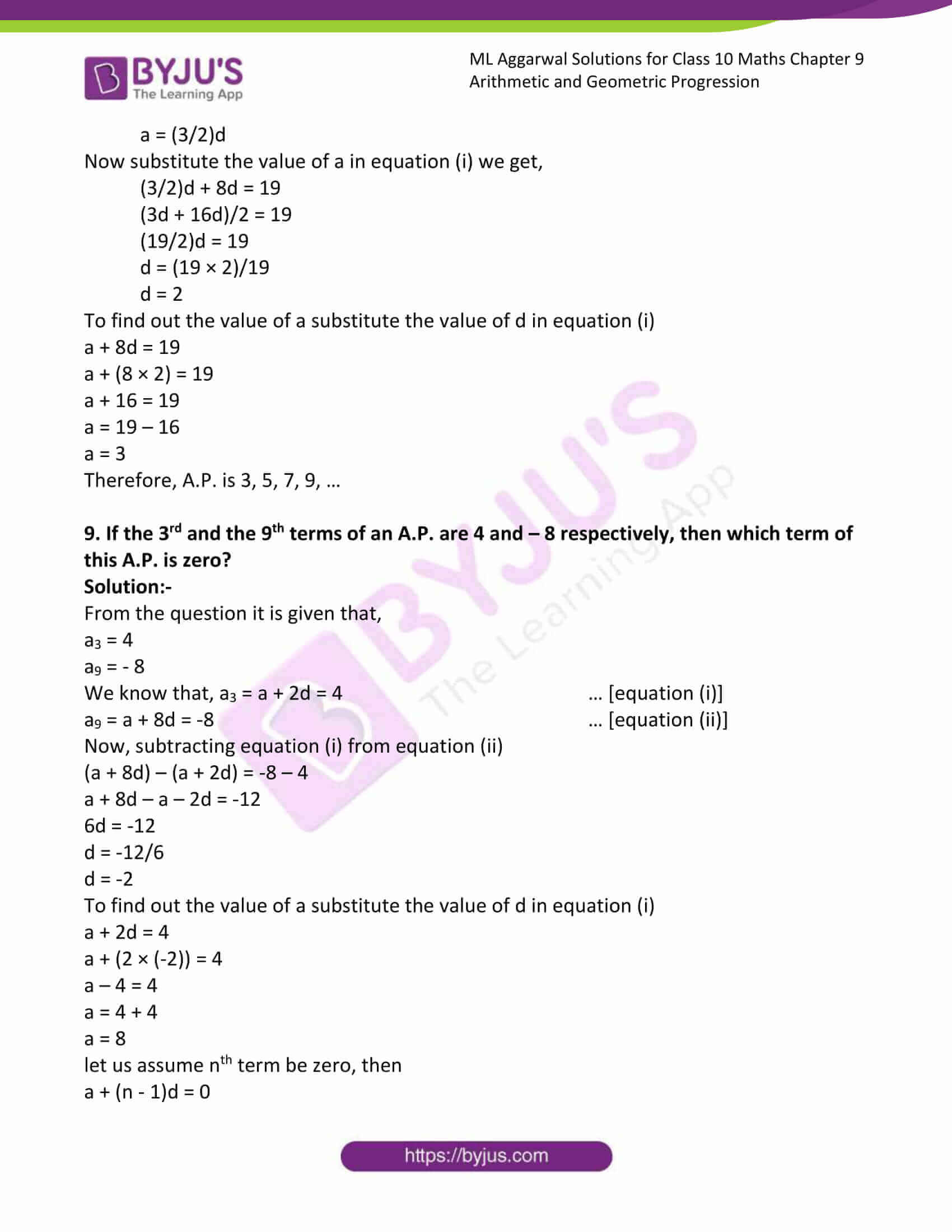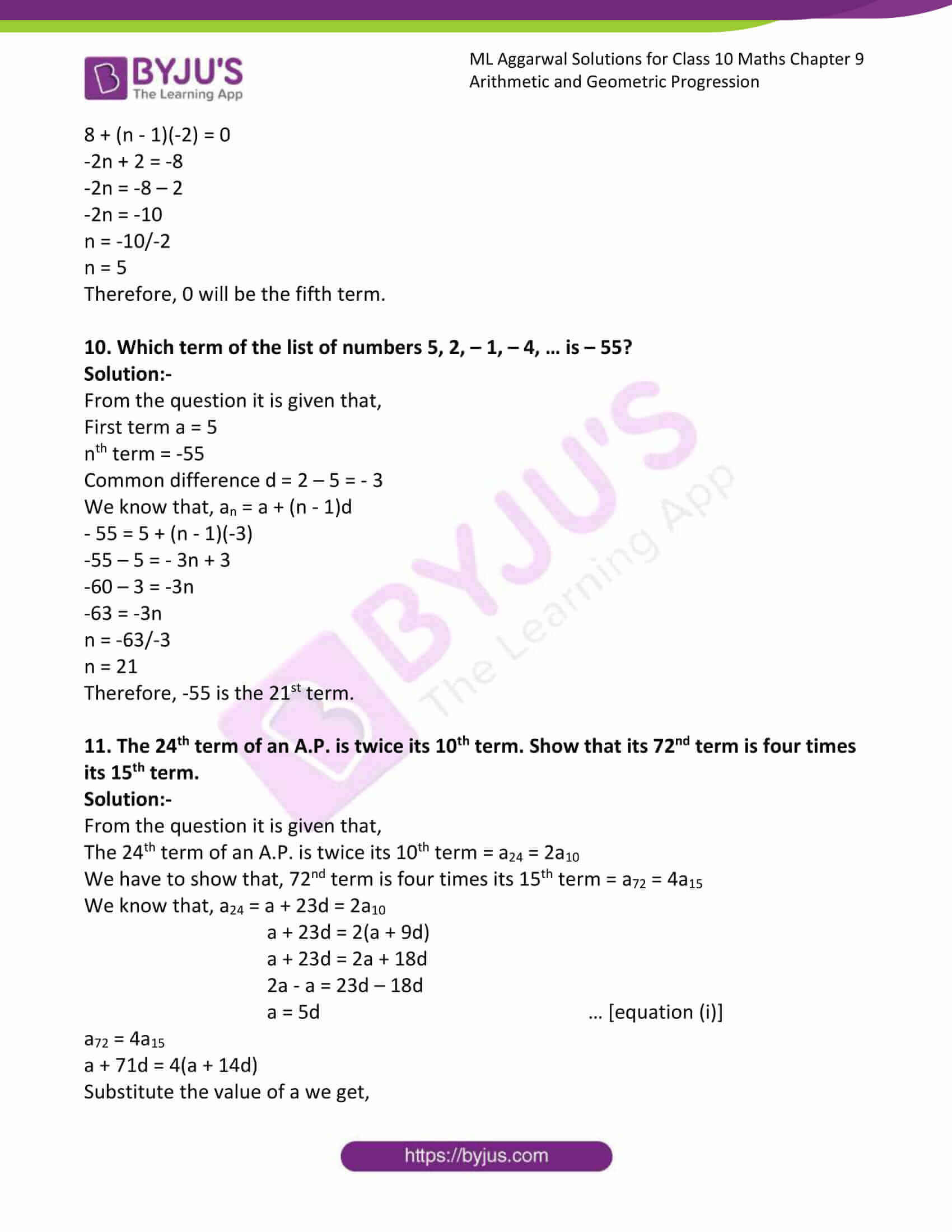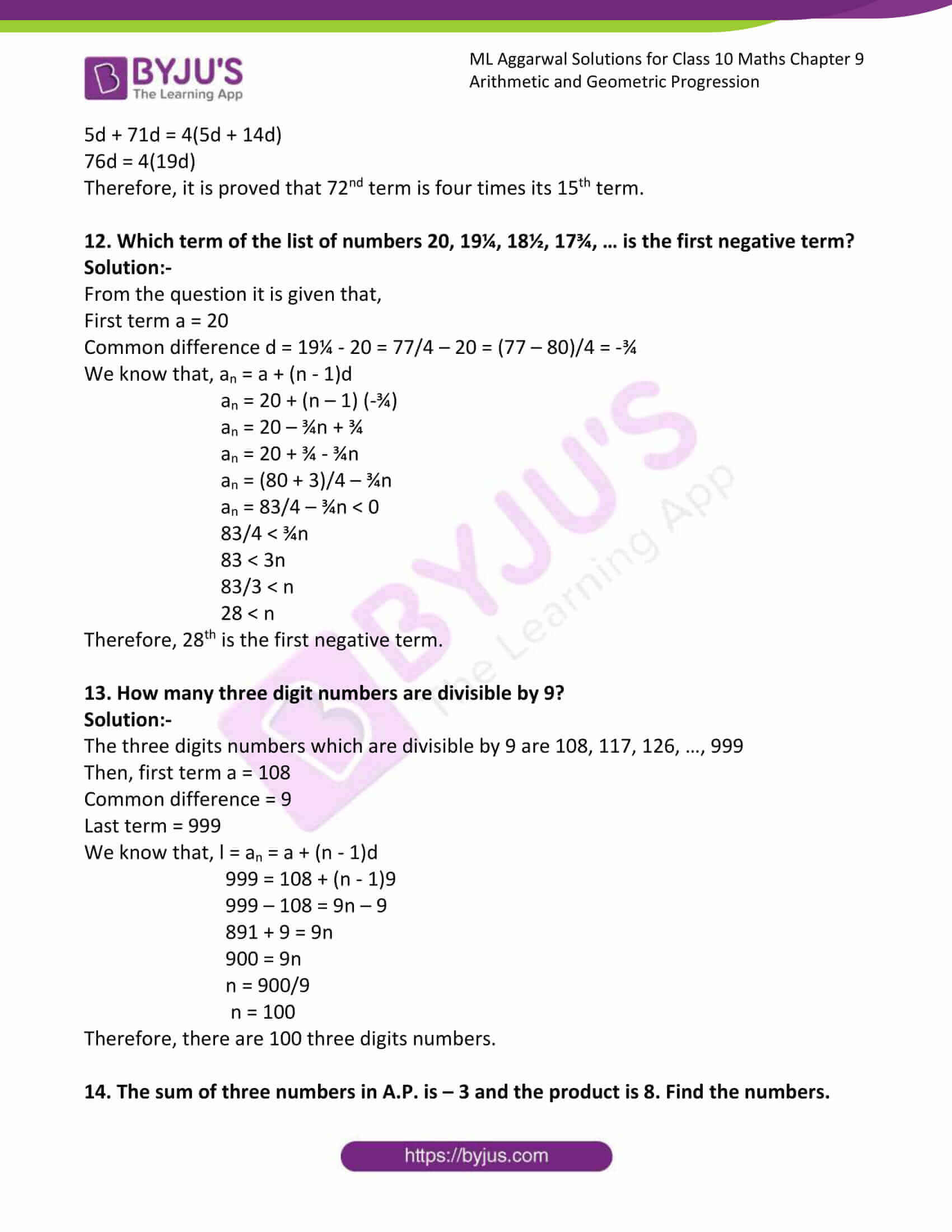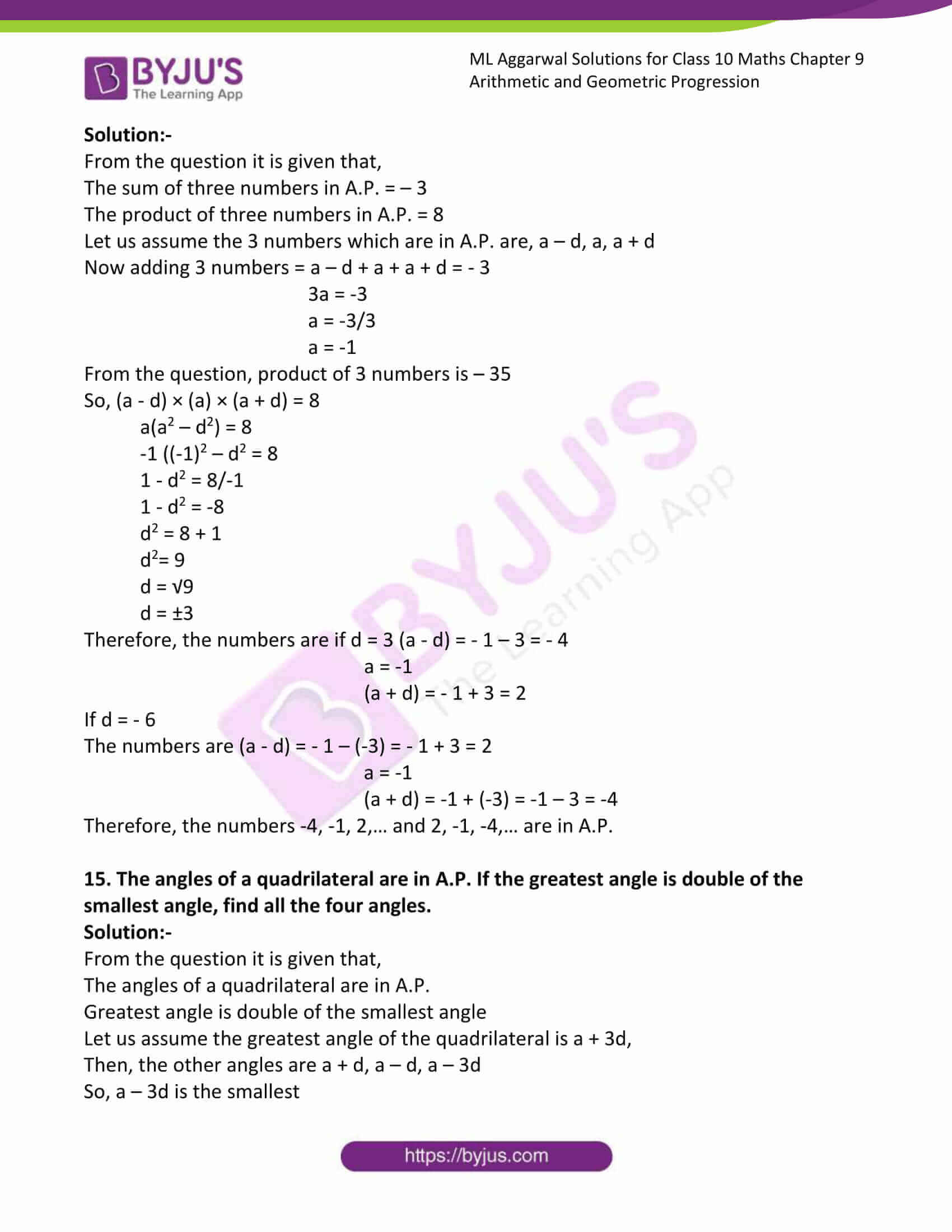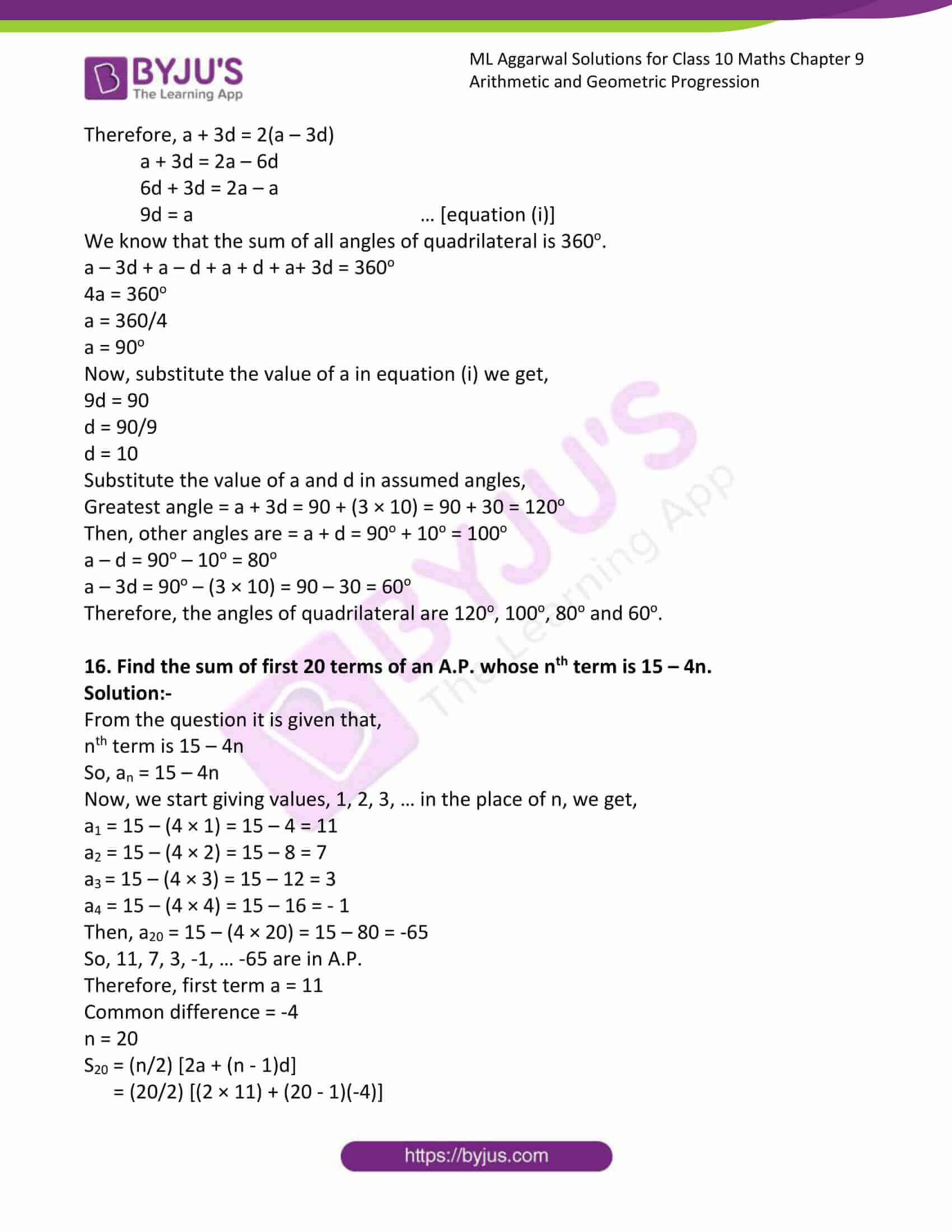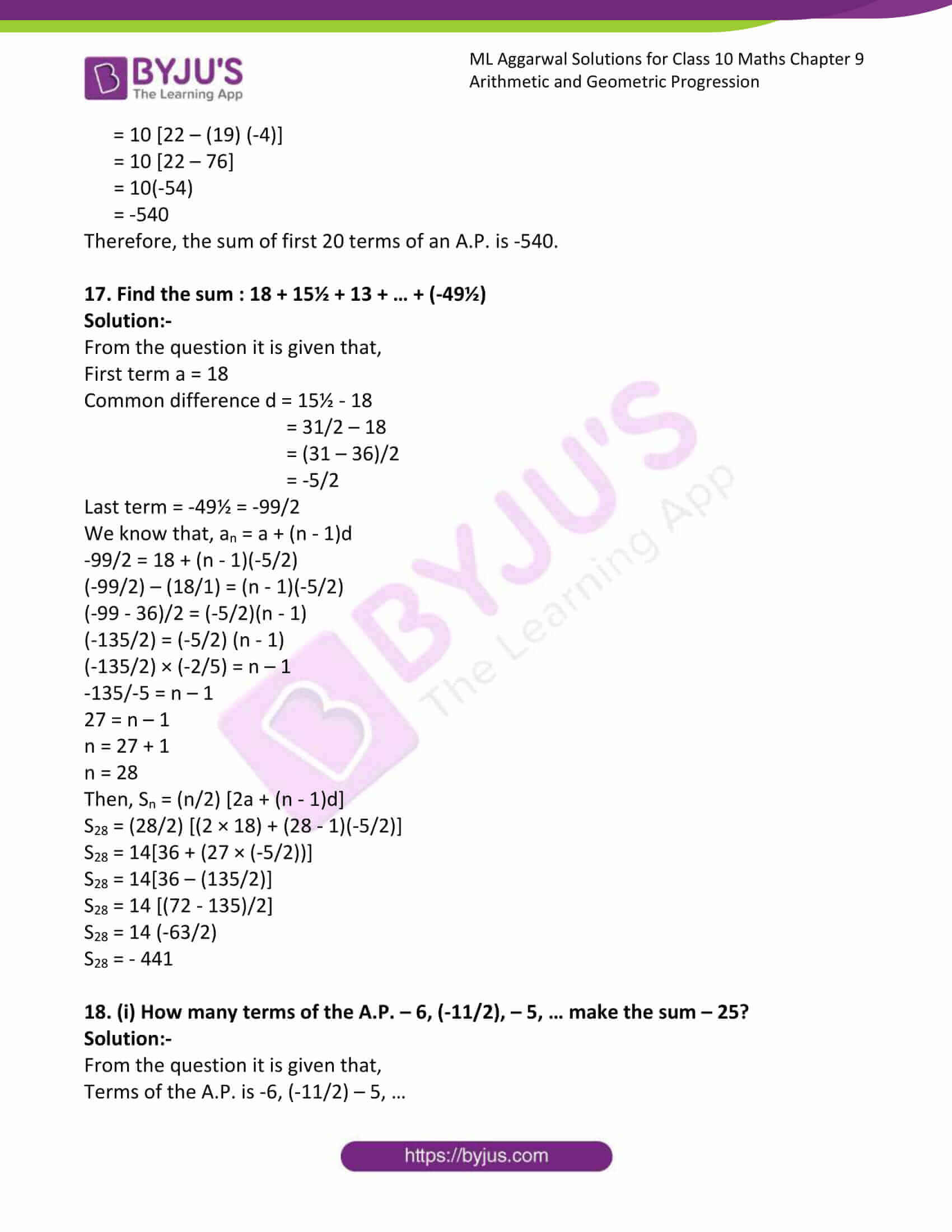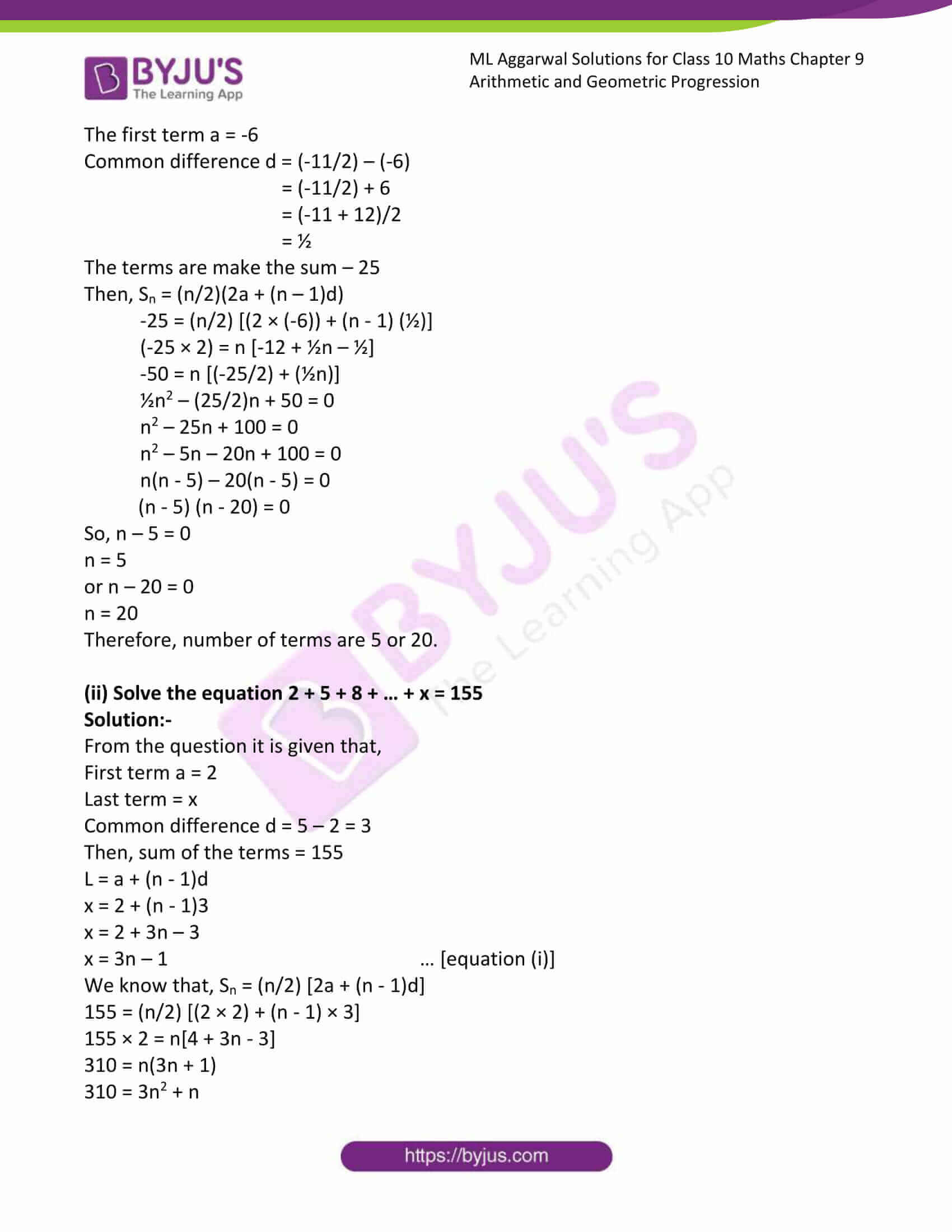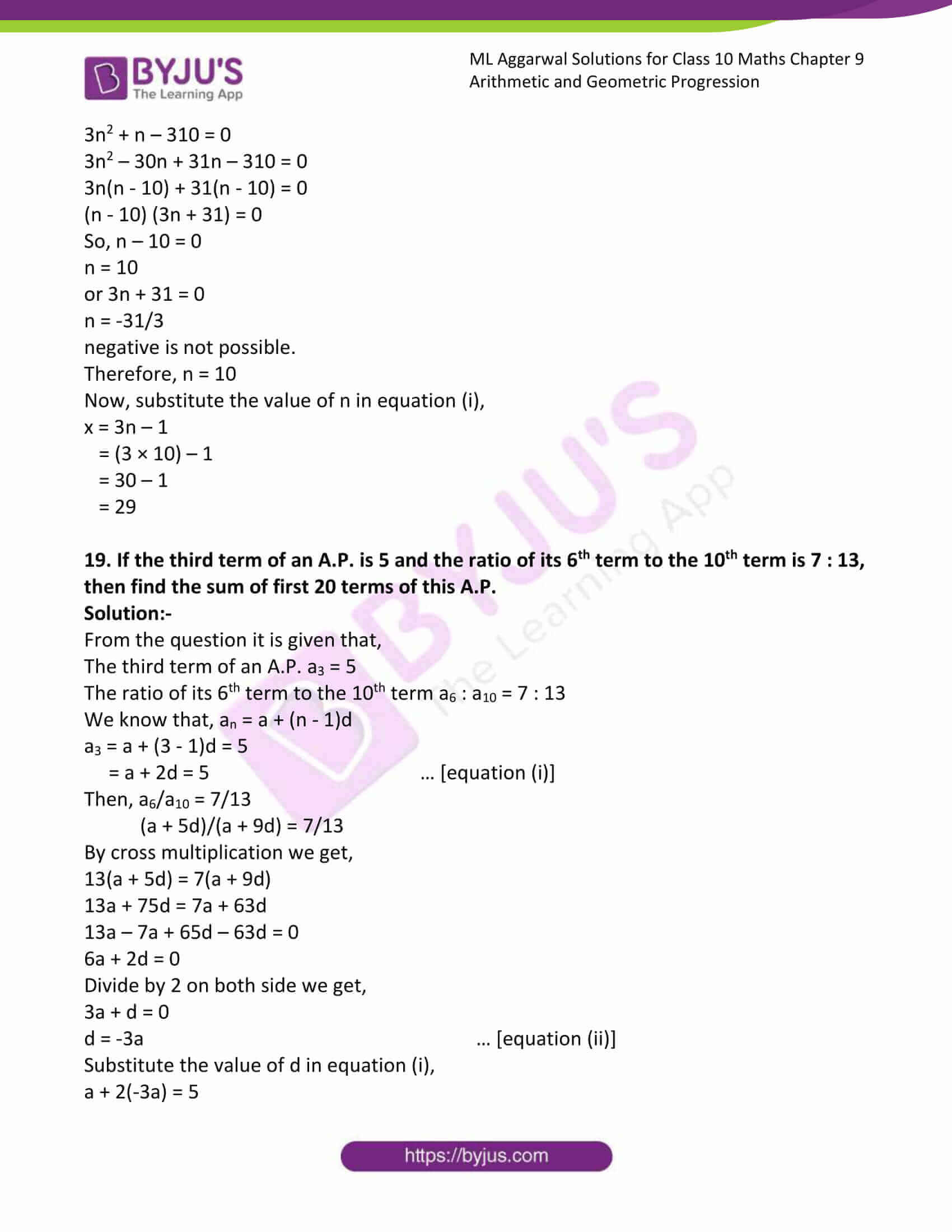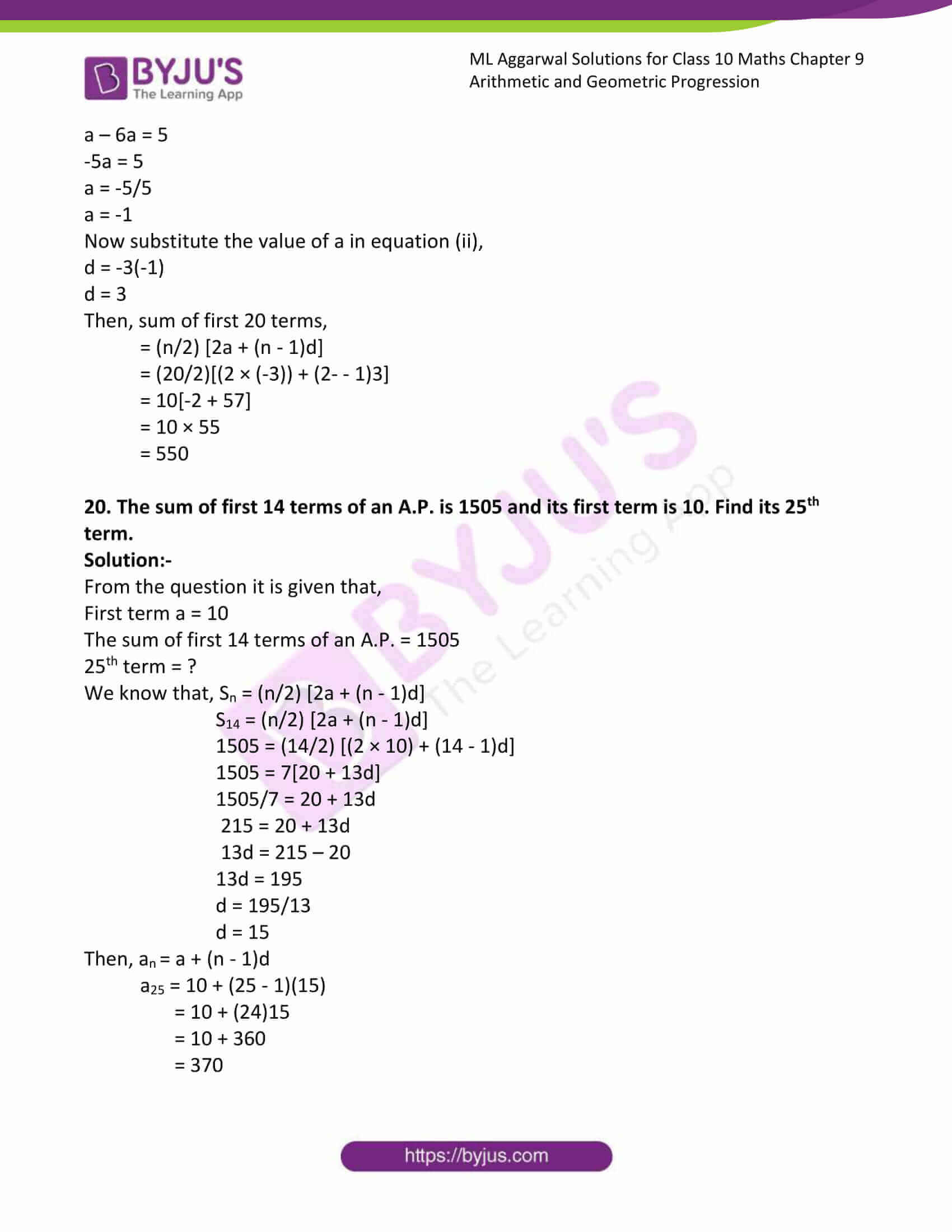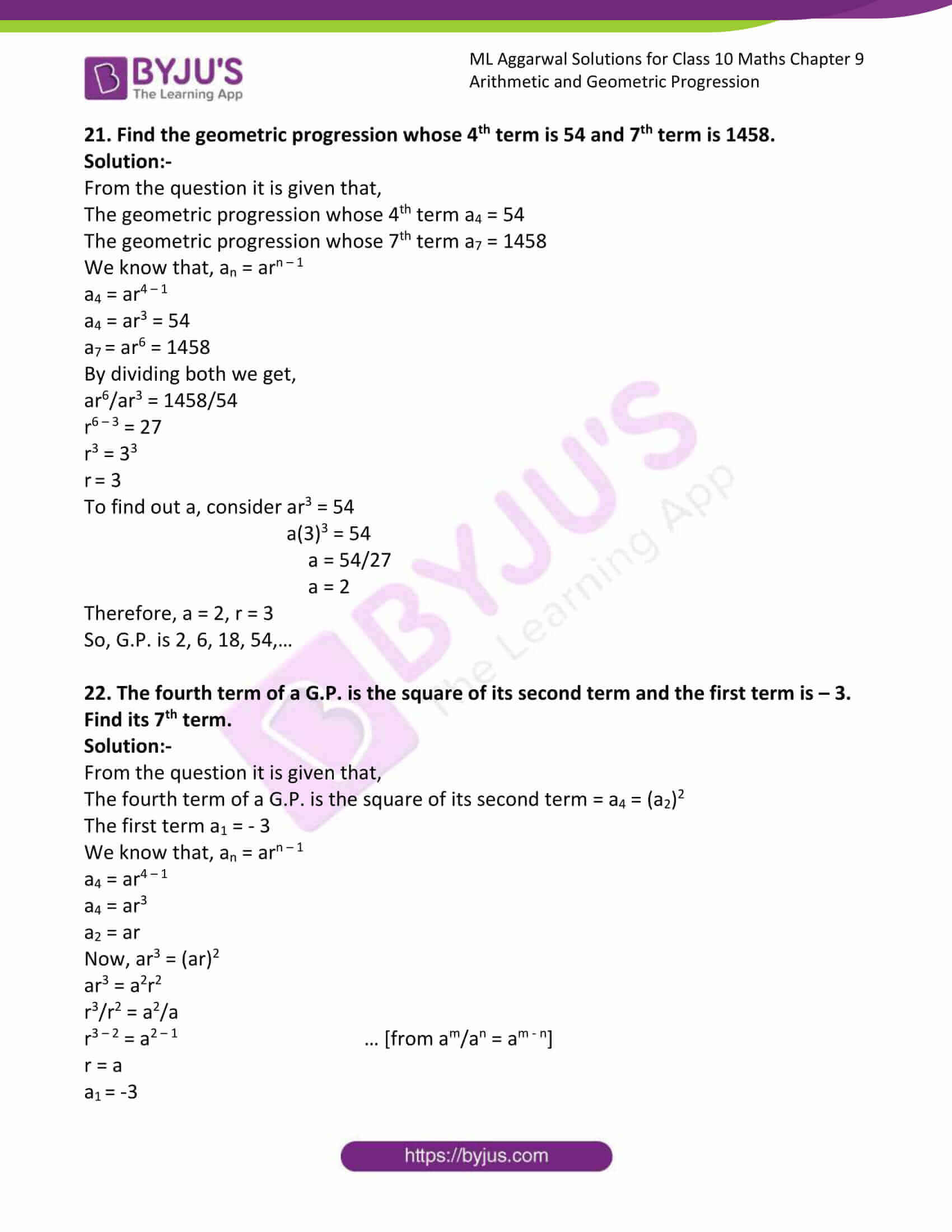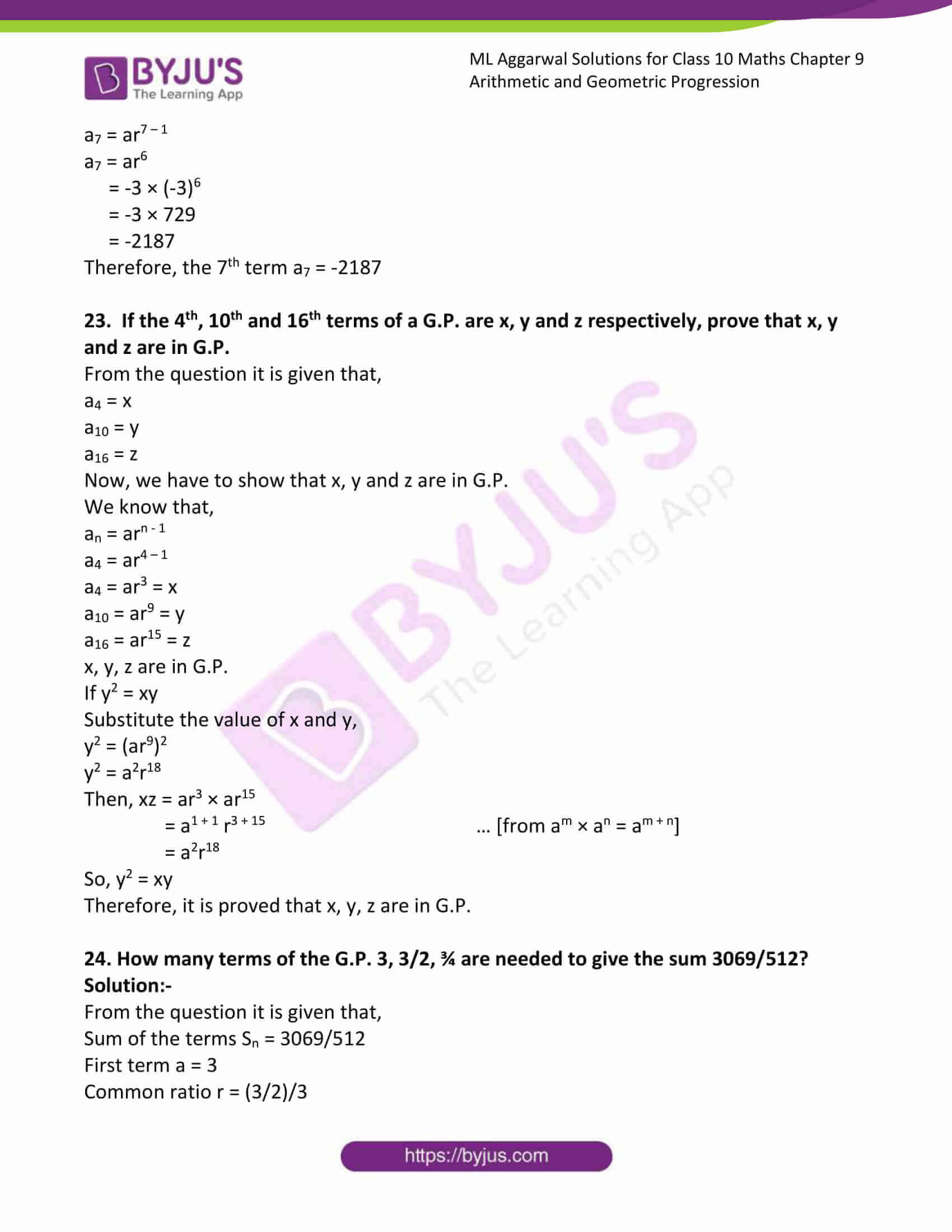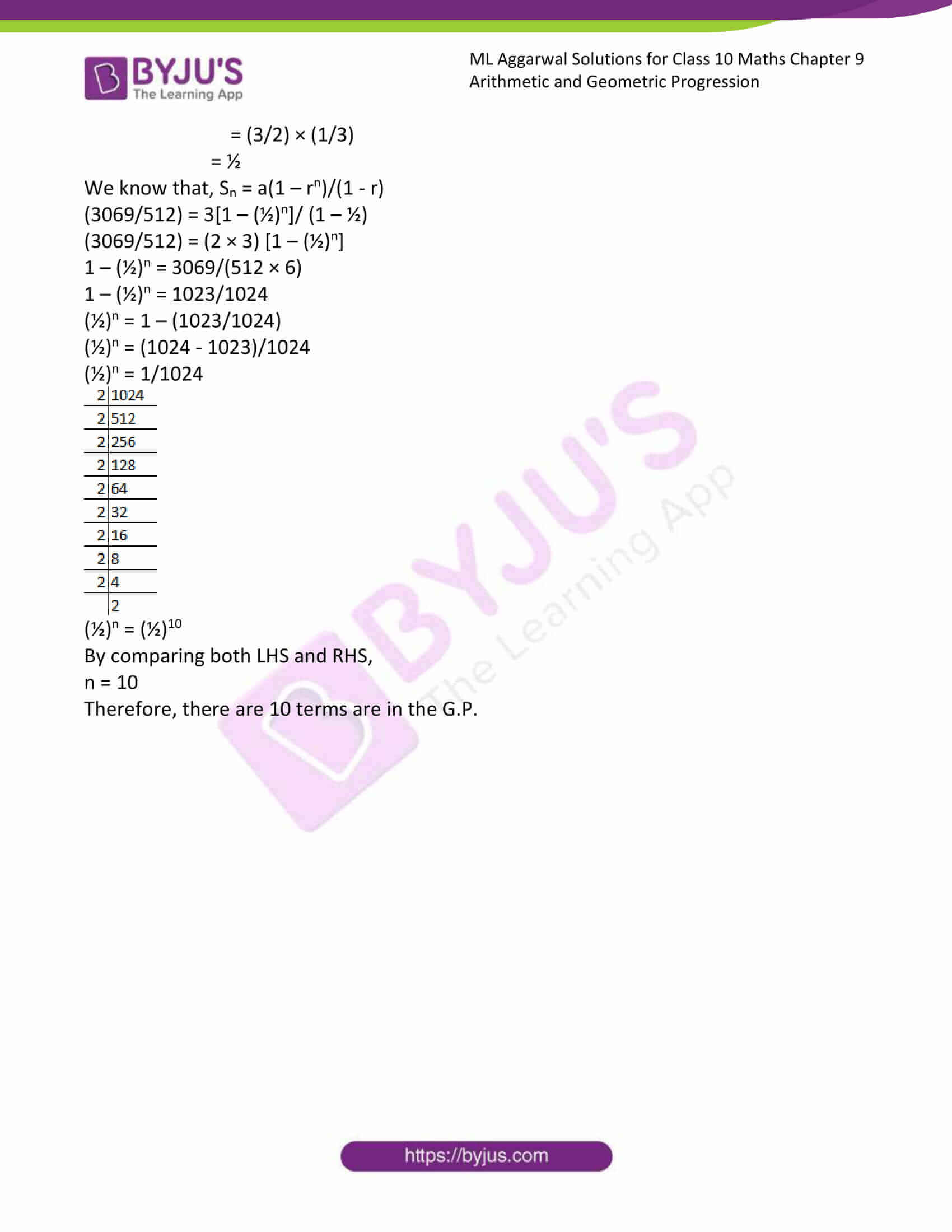### Access answers to ML Aggarwal Solutions for Class 10 Maths Chapter 9 Arithmetic and Geometric Progression

Exercise 9.1

1. For the following A.P.s, write the first term ‘a’ and the common difference ‘d’:

(i) 3, 1, – 1, – 3, …

Solution:-

From the question,

The first term a = 3

Then, difference d = 1 – 3 = – 2

– 1 – 1 = – 2

– 3 – (-1) = – 3 + 1 = -2

Therefore, common difference d = – 2

(ii) 1/3, 5/3, 9/3, 13/3, ….

Solution:-

From the question,

The first term a = 1/3

Then, difference d = 5/3 – 1/3 = (5 – 1)/3 = 4/3

9/3 – 5/3 = (9 – 5)/3 = 4/3

13/3 – 9/3 = (13 – 9)/3 = 4/3

Therefore, common difference d = 4/3

(iii) -3.2, -3, -2.8, -2.6, ….

Solution:-

From the question,

The first term a = -3.2

Then, difference d = -3 – (-3.2) = -3 + 3.2 = 0.2

-2.8 – (-3) = -2.8 + 3 = 0.2

-2.6 – (-2.8) = -2.6 + 2.8 = 0.2

Therefore, common difference d = 0.2

2. Write first four terms of the A.P., when the first term a and the common difference d are given as follows:

(i) a = 10, d = 10

Solution:-

From the question it is given that,

First term a = 10

Common difference d = 10

Then the first four terms are = 10 + 10 = 20

20 + 10 = 30

30 + 10 = 40

Therefore, first four terms are 10, 20, 30 and 40.

(ii) a = -2, d = 0

Solution:-

From the question it is given that,

First term a = -2

Common difference d = 0

Then the first four terms are = -2 + 0 = -2

-2 + 0 = -2

-2 + 0 = -2

Therefore, first four terms are -2, -2, -2 and -2.

(iii) a = 4, d = -3

Solution:-

From the question it is given that,

First term a = 4

Common difference d = -3

Then the first four terms are = 4 + (-3) = 4 – 3 = 1

1 + (-3) = 1 – 3 = – 2

-2 + (-3) = -2 – 3 = – 5

Therefore, first four terms are 4, 1, -2 and -5.

(iv) a = ½, d = -1/6

Solution:-

From the question it is given that,

First term a = ½

Common difference d = -1/6

Then the first four terms are = ½ + (-1/6) = ½ – 1/6 = (3 – 1)/6 = 2/6 = 1/3

1/3 + (-1/6) = 1/3 – 1/6 = (2 – 1)/6 = 1/6

1/6 + (-1/6) = 1/6 – 1/6 = 0

Therefore, first four terms are ½, 1/3, 1/6 and 0.

3. Which of the following lists of numbers form an A.P.? If they form an A.P., find the common difference d and write the next three terms:

(i) 4, 10, 16, 22, …

Solution:-

From the question it is given that,

First term a = 4

Then, difference d = 10 – 4 = 6

16 – 10 = 6

22 – 16 = 6

Therefore, common difference d = 6

Hence, the numbers are form A.P.

(ii) -2, 2, -2, 2, …

Solution:-

From the question it is given that,

First term a = -2

Then, difference d = -2 – 2 = – 4

-2 – 2 = -4

2 – (-2) = 2 + 2 = 4

Therefore, common difference d is not same in the given numbers.

Hence, the numbers are not form A.P.

(iii) 2, 4, 8, 16, …

Solution:-

From the question it is given that,

First term a = 2

Then, difference d = 4 – 2 = 2

8 – 4 = 4

16 – 8 = 8

Therefore, common difference d is not same in the given numbers.

Hence, the numbers are not form A.P.

(iv) 2, 5/2, 3, 7/2, …

Solution:-

From the question it is given that,

First term a = 2

Then, difference d = 5/2 – 2 = (5 – 4)/2 = ½

3 – 5/2 = (6 – 5)/2 = ½

7/2 – 3 = (7 – 6)/2 = ½

Therefore, common difference d = ½

Hence, the numbers are form A.P.

(v) – 10, -6, -2, 2, …

Solution:-

From the question it is given that,

First term a = -10

Then, difference d = -6 – (- 10) = – 6 + 10 = 4

-2 – (-6) = – 2 + 6 = 4

2 – (-2) = 2 + 2 = 4

Therefore, common difference d = 4

Hence, the numbers are form A.P.

(vi) 12, 32, 52, 72, …

Solution:-

From the question it is given that,

First term a = 12 = 1

Then, difference d = 32 – 12 = 9 – 1 = 8

52 – 32 = 25 – 9 = 16

72 – 52 = 49 – 25 = 24

Therefore, common difference d is not same in the given numbers.

Hence, the numbers are not form A.P.

Exercise 9.2

1. Find the A.P. whose nth term is 7 – 3K. Also find the 20th term.

Solution:-

From the question it is given that,

nth term is 7 – 3k

So, Tn = 7 – 3n

Now, we start giving values, 1, 2, 3, … in the place of n, we get,

T1 = 7 – (3 × 1) = 7 – 3 = 4

T2 = 7 – (3 × 2) = 7 – 6 = 1

T3 = 7 – (3 × 3) = 7 – 9 = -2

T4 = 7 – (3 × 4) = 7 – 12 = – 5

T20 = 7 – (3 × 20) = 7 – 60 = – 53

Therefore, A.P. is 4, 1, -2, -5, …

So, 20th term is – 53

2. Find the indicated terms in each of following A.P.s:

(i) 1, 6, 11, 16, …; a20

Solution:-

From the question,

The first term a = 1

Then, difference d = 6 – 1 = 5

11 – 6 = 5

16 – 11 = 5

Therefore, common difference d = 5

From the formula, an = a + (n – 1)d

So, a20 = a + (20 – 1)d

= 1 + (20 – 1)5

= 1 + (19)5

= 1 + 95

= 96

Therefore, a20 = 96

(ii) -4, -7, -10, -13, …, a25, an

Solution:-

From the question,

The first term a = -4

Then, difference d = -7 – (-4) = – 7 + 4 = -3

-10 – (-7) = -10 + 7 = -3

-13 – (-10) = -13 + 10 = -3

Therefore, common difference d = -3

From the formula, an = a + (n – 1)d

So, a25 = a + (25 – 1)d

= -4 + (25 – 1)(-3)

= -4 + (24)-3

= – 4 – 72

= -76

Therefore, a25 = -76

Now, an = a + (n – 1)d

an = -4 + (n – 1)-3

= -4 – 3n + 3

= -1 – 3n

3. Find the nth term and the 12th term of the list of numbers: 5, 2, – 1, – 4, …

Solution:-

From the question,

The first term a = 5

Then, difference d = 2 – 5 = – 3

-1 – 3 = -3

– 4 – (-1) = -4 + 1 = -3

Therefore, common difference d = -3

From the formula, an = a + (n – 1)d

Tn = a + (n – 1)d

= 5 + (n – 1)-3

= 5 – 3n + 3

= 8 – 3n

So, T12 = a + (12 – 1)d

= 5 + (12 – 1)(-3)

= 5 + (11)-3

= 5 – 33

= – 28

4. (i) If the common difference of an A.P. is – 3 and the 18th term is – 5, then find its first term.

Solution:-

From the question it is given that,

The 18th term = -5

Then, common difference d = -3

Tn = a + (n – 1)d

So, T18 = a + (18 – 1)d

-5 = a + (18 – 1)(-3)

-5 = a + (17)(-3)

-5 = a – 51

a = 51 – 5

a = 46

Therefore, first term a = 46

(ii) If the first term of an A.P. is – 18 and its 10th term is zero, then find its common difference.

Solution:-

From the question it is given that,

The 10th term = 0

Then, first term a = -18

Tn = a + (n – 1)d

So, T10 = a + (10 – 1)d

0 = -18 + (10 – 1)d

0 = -18 + 9d

9d = 18

d = 18/9

d = 2

Therefore, common difference d = 2

5. Which term of the A.P.

(i) 3, 8, 13, 18, … is 78?

Solution:-

Let us assume 78 as nth term.

From the question,

The first term a = 3

Then, difference d = 8 – 3 = 5

13 – 8 = 5

18 – 13 = 5

Therefore, common difference d = 5

Tn = a + (n – 1)d

So, 78 = a + (n – 1)d

78 = 3 + (n – 1)5

78 = 3 + 5n – 5

78= -2 + 5n

5n = 78 + 2

5n = 80

n = 80/5

n = 16

Therefore, 78 is 16th term.

(ii) 18, 15½, 13, … is – 47?

Solution:-

Convert mixed fraction into improper fraction = 15½ = 31/2

Let us assume -47 as nth term.

From the question,

The first term a = 18

Then, difference d = 31/2 – 18 = (31 – 36)/2 = -5/2

13 – 31/2 = (26 – 31)/2 = -5/2

Therefore, common difference d = -5/2

Tn = a + (n – 1)d

So, -47 = a + (n – 1)d

-47 = 18 + (n – 1) (-5/2)

-47 = 18 – 5/2n + 5/2

-47 – 18 = -5/2n + 5/2

-65 = -5/2n + 5/2

-65 – 5/2 = – 5/2n

(-130 – 5)/2 = -5/2n

-135/2 = -5/2n

n = (-135/2) × (-2/5)

n = -135/-5

n = 27

Therefore, -47 is 27th term.

6. (i) Check whether – 150 is a term of the A.P. 11, 8, 5, 2, …

Solution:-

From the question it is given that,

The first term a = 11

Then, difference d = 8 – 11 = -3

5 – 8 = -3

2 – 5 = -3

Then, common difference d = – 3

Let us assume -150 as nth term,

Tn = a + (n – 1)d

So, -150 = 11 + (n – 1) (-3)

-150 = 11 – 3n + 3

-150 = 14 – 3n

3n = 150 + 14

3n = 164

n = 164/3

n =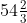Therefore, – 150 is not a term of the A.P. 11, 8, 5, 2, …

(ii) Find whether 55 is a term of the A.P. 7, 10, 13, … or not. If yes, find which term is it.

Solution:-

From the question it is given that,

The first term a = 7

Then, difference d = 10 – 7 = 3

13 – 10 = 3

Then, common difference d = 3

Let us assume 55 as nth term,

Tn = a + (n – 1)d

So, 55 = 7 + (n – 1)3

55 = 7 + 3n – 3

55 = 4 + 3n

3n = 55 – 4

3n = 51

n = 51/3

n = 17

Therefore, 55 is 17th term of the A.P. 7, 10, 13, …

7. (i) Find the 20th term from the last term of the A.P. 3, 8, 13, …, 253.

Solution:-

Let us assume 253 as nth term.

From the question,

The first term a = 3

Then, difference d = 8 – 3 = 5

13 – 8 = 5

Therefore, common difference d = 5

Tn = a + (n – 1)d

So, 253 = a + (n – 1)d

253 = 3 + (n – 1)5

253 = 3 + 5n – 5

253 = -2 + 5n

5n = 253 + 2

5n = 255

n = 255/5

n = 51

Therefore, 253 is 51th term.

Now, assume ‘P’ be the 20th term from the last.

Then, P = L – (n – 1)d

= 253 – (20 – 1) 5

= 253 – (19) 5

= 253 – 95

P = 158

Therefore, 158 is the 20th term from the last.

(ii) Find the 12th from the end of the A.P. – 2, – 4, – 6, …, – 100.

Solution:-

Let us assume -100 as nth term.

From the question,

The first term a = -2

Then, difference d = – 4 – (-2) = – 4 + 2 = -2

-6 – (-4) = -6 + 4 = – 2

Therefore, common difference d = -2

Tn = a + (n – 1)d

So, – 100 = a + (n – 1)d

– 100 = -2 + (n – 1)(-2)

– 100 = -2 – 2n + 2

– 100 = -2n

n = -100/-2

n = 50

Therefore, -100 is 50th term.

Now, assume ‘P’ be the 12th term from the last.

Then, P = L – (n – 1)d

= -100 – (12 – 1) (-2)

= -100 – (11) (-2)

= -100 + 22

P = – 78

Therefore, -78 is the 12th term from the last of the A.P. – 2, – 4, – 6, …

8. Find the sum of the two middle most terms of the A.P.

-4/3, -1, -2/3, …,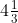Solution:-

From the question,

Last term (nth) == 13/3

First term a = -4/3

Then, difference d = -1 – (-4/3) = -1 + 4/3 = (-3 + 4)/3 = 1/3

= -2/3 – (-1) = -2/3 + 1 = (-2 + 3)/3 = 1/3

Therefore, common difference d = 1/3

We know that,

Tn = a + (n – 1)d

So, 13/3 = -4/3 + (n – 1)(1/3)

13/3 + 4/3 = 1/3n – 1/3

13/3 + 4/3 + 1/3 = 1/3n

(13 + 4 + 1)/3 = 1/3n

18/3 = 1/3n

6 = 1/3n

n = 6 × 3

n = 18

So, middle term is 18/2 and (18/2) + 1 = 9th and 10th term

Then, a9 + a10 = a + 8d + a + 9d

= 2a + 17d

Now substitute the value of ‘a’ and ‘d’.

= 2(-4/3) + 17(1/3)

= -8/3 + 17/3

= (-8 + 17)/3

= 9/3

= 3

Therefore, the sum of the two middle most terms of the A.P is 3.

9. Which term of the A.P. 53, 48, 43,… is the first negative term ?

Solution:-

From the question,

The first term a = 53

Then, difference d = 48 – 53 = -5

= 43 – 48 = -5

Therefore, common difference d = -5

Tn = a + (n – 1)d

= 53 + (n – 1)(-5)

= 53 – 5n + 5

= 58 – 5n

5n = 58

n= 58/5

n = 11.6 ≈ 12

Therefore, 12th term is the first negative term of the A.P. 53, 48, 43,…

10. Determine the A.P. whose third term is 16 and the 7th term exceeds the 5th term by 12.

Solution:-

From the question it is given that,

T3 = 16

The 7th term exceeds the 5th term by 12 = T7 – T5 = 12

We know that, Tn = a + (n – 1)d

So, T3 = a + 2d = 16 … [equation (i)]

T7 – T5 = (a + 6d) – (a + 4d) = 12 … [equation (ii)]

12 = a + 6d – a – 4d

12 = 2d

d = 12/2

d = 6

Now, substitute value of d in equation (i) we get,

Then, T3 = a + 2d

16 = a + 2(6)

a = 16 – 12

a = 4

Therefore, A.P. is 4 + 6 = 10, 10 + 6 = 16, 16 + 6 = 22

Hence, the four term of A.P. is 4, 10, 16, 22, ….

11. Find the 20th term of the A.P. whose 7th term is 24 less than the 11th term, first term being 12.

Solution:-

From the question it is given that,

First term a = 12

7th term is 24 less than the 11th term = T11 – T7 = 24

T11 – T7 = (a + 10d) – (a + 6d) = 24

24 = a + 10d – a – 6d

24 = 4d

d = 24/4

d = 6

Now, T20 = a + 19d

Substitute the values of a and d,

T20 = 12 + 19(6)

T20 = 12 + 114

T20 = 126

12. Find the 31st term of an A.P. whose 11th term is 38 and 6th term is 73.

Solution:-

From the question it is given that,

T11 = 38

T6 = 73

Let us assume ‘a’ be the first term and ‘d’ be the common difference,

So, T11 = a + 10d = 38 equation (i)

T6 = a + 5d = 73 equation (ii)

Subtracting both equation (i) and equation (i),

(a + 10d) – (a + 5d) = 73 – 38

a + 10d – a – 5d = 35

5d = 35

d = 35/5

d = 7

now, substitute the value of d in equation (i) to find out a, we get

a + 10d = 38

a + 10(7) = 38

a + 70 = 38

a = 38 – 70

a = -32

Therefore, T31 = a + 30d

= -32 + 30(7)

= -32 + 210

= 178

13. If the seventh term of an A.P. is 1/9 and its ninth term is 1/7, find its 63rd term.

Solution:-

From the question it is given that,

T9 = 1/7

T7 = 1/9

Let us assume ‘a’ be the first term and ‘d’ be the common difference,

So, T9 = a + 8d = 1/7 equation (i)

T7 = a + 6d = 1/9 equation (ii)

Subtracting both equation (i) and equation (i),

(a + 6d) – (a + 8d) = 1/9 – 1/7

a + 6d – a – 8d = (7 – 9)/63

-2d = -2/63

d = (-2/63) × (-1/2)

d = 1/63

now, substitute the value of d in equation (ii) to find out a, we get

a + 6(1/63) = 1/9

a = 1/9 – 6/63

a = (7 – 6)/63

a = 1/63

Therefore, T63 = a + 62d

= 1/63 + 62(1/63)

= 1/63 + 62/63

= (1 + 62)/63

= 63/63

= 1

14. (i) The 15th term of an A.P. is 3 more than twice its 7th term. If the 10th term of the A.P. is 41, find its nth term.

Solution:-

From the question it I s given that,

T10 = 41

T10 = a + 9d = 41 … [equation (i)]

T15 = a + 14d = 2T7 + 3

= a + 14d = 2(a + 6d) + 3

= a + 14d = 2a + 12 d + 3

-3 = 2a – a + 12d – 14d

a – 2d = -3 … [equation (ii)]

Now, subtracting equation (ii) from (i), we get,

(a + 9d) – (a – 2d) = 41 – (-3)

a + 9d – a + 2d = 41 + 3

11d = 44

d = 44/11

d = 4

Then, substitute the value of d is equation (i) to find a,

a + 9(4) = 41

a + 36 = 41

a = 41 – 36

a = 5

Therefore, nth term = Tn = a + (n – 1)d

= 5 + (n – 1)4

= 5 + 4n – 4

= 1 + 4n

(ii) The sum of 5th and 7th terms of an A.P. is 52 and the 10th term is 46. Find the A.P.

Solution:-

From the question it is given that,

a5 + a7 = 52

(a + 4d) + (a + 6d) = 52

a + 4d + a + 6d = 52

2a + 10d = 52

Divide both the side by 2 we get,

a + 5d = 26 … equation (i)

Given, a10 = a + 9d = 46

a + 9d = 46 … equation (ii)

Now subtracting equation (i) from equation (ii),

(a + 9d) – (a + 5d) = 46 – 26

a + 9d – a – 5d = 20

4d = 20

d = 20/4

d = 5

Substitute the value of d in equation (i) to find out a,

a + 5d = 26

a + 5(5) = 26

a + 25 = 26

a = 26 – 25

a = 1

Then, a2 = a + d

= 1 + 5 = 6

a3 = a2 + d

= 6 + 5

= 11

a4 = a3 + d

= 11 + 5

= 16

Therefore, 1, 6, 11, 16,… are A.P.

15. If 8th term of an A.P. is zero, prove that its 38th term is triple of its 18th term.

Solution:-

Froom the question it is given that,

T8 = 0

We have to prove that, 38th term is triple of its 18th term = T38 = 3T18

T8 = a + 7d = 0

T8 = a = -7d

T38 = a + 37d

= -7d + 37d

= 30d

Take, T18 = a + 17d

Substitute the value of a and d,

T18 = -7d + 17d

T18 = 10d

By comparing results of T38 and T18, 38th term is triple of its 18th term.

16. Which term of the A.P. 3, 10, 17,… will be 84 more than its 13th term?

Solution:-

From the question it is given that,

First term a = 3

Common difference d = 10 – 3 = 7

Then, T13 = a + 12d

= 3 + 12(7)

= 3 + 84

= 87

Let us assume that, nth term is 84 more than its 13th term

So, Tn = 84 + 87

= 171

We know that, Tn = a + (n – 1)d = 171

3 + (n – 1)7 = 171

3 + 7n – 7 = 171

7n – 4 = 171

7n = 171 + 4

7n = 175

n = 175/7

n = 25

17. (i) How many two digit numbers are divisible by 3 ?

Solution:-

The two digits numbers divisible by 3 are, 12, 15, 18, 21, 24,…..,99.

The above numbers are A.P.

So, first number a = 12

Common difference d = 15 – 12 = 3

Then, last number is 99

We know that, Tn (last number) = a + (n – 1)d

99 = 12 + (n – 1)3

99 = 12 + 3n – 3

99 = 9 + 3n

99 – 9 = 3n

3n = 90

n = 90/3

n = 30

Therefore, 30 two digits number are divisible by 3.

(ii) Find the number of natural numbers between 101 and 999 which are divisible by both 2 and 5.

Solution:-

The natural numbers which are divisible by both 2 and 5 are 110, 120, 130, 140, ….,999

The above numbers are A.P.

So, first number a = 110

Common difference d = 120 – 110 = 10

Then, last number is 999

We know that, Tn (last number) = a + (n – 1)d

999 = 110 + (n – 1)10

999 = 110 + 10n – 10

999 = 100 + 10n

999 – 100 = 10n

10n = 888

n = 888/10

n = 88

The number of natural numbers which are divisible by both 2 and 5 are 88.

18. If the numbers n – 2, 4n – 1 and 5n + 2 are in A.P., find the value of n.

Solution:-

From the question it is given that, n – 2, 4n – 1 and 5n + 2 are in A.P.

Multiplying by 2 to 4n – 1 then it becomes = 8n – 2

So, 8n – 2 = n – 2 + 5n + 2

8n – 2 = 6n

8n – 6n = 2

2n = 2

n = 2/2

n = 1

19. The sum of three numbers in A.P. is 3 and their product is – 35. Find the numbers.

Solution:-

From the question it is given that,

The sum of three numbers in A.P. = 3

Given, Their product = -35

Let us assume the 3 numbers which are in A.P. are, a – d, a, a + d

Now adding 3 numbers = a – d + a + a + d = 3

3a = 3

a = 3/3

a = 1

From the question, product of 3 numbers is – 35

So, (a – d) × (a) × (a + d) = – 35

(1 – d) × (1) × (1 + d) = – 35

12 – d2 = – 35

d2 = 35 + 1

d2 = 36

d = √36

d = ±6

Therefore, the numbers are (a – d) = 1 – 6 = – 5

a = 1

(a + d) = 1 + 6 = 7

If d = – 6

The numbers are (a – d) = 1 – (-6) = 1 + 6 = 7

a = 1

(a + d) = 1 + (-6) = 1 – 6 = -5

Therefore, the numbers -5, 1, 7,… and 7, 1, -5,… are in A.P.

20. The sum of three numbers in A.P. is 30 and the ratio of first number to the third number is 3 : 7. Find the numbers.

Solution:-

From the question it is given that, sum of three numbers in A.P. = 30

The ratio of first number to the third number is 3: 7

Let us assume the 3 numbers which are in A.P. are, a – d, a, a + d

Now adding 3 numbers = a – d + a + a + d = 30

3a = 30

a = 30/3

a = 10

Given ratio 3 : 7 = a – d : a + d

3/7 = (a – d)/(a + d)

(a – d)7 = 3(a + d)

7a – 7d = 3a + 3d

7a – 3a = 7d + 3d

4a = 10d

4(10) = 10d

40 = 10d

d = 40/10

d = 4

Therefore, the numbers are a – d = 10 – 4 = 6

a = 10

a + d = 10 + 4 = 14

So, 6, 10, 14, … are in A.P.

21. The sum of the first three terms of an A.P.is 33. If the product of the first and the third terms exceeds the second term by 29, find the A.P.

Solution:-

From the question it is given that, sum of the first three terms of an A.P. is 33.

Let us assume the 3 numbers which are in A.P. are, a – d, a, a + d

Now adding 3 numbers = a – d + a + a + d = 33

3a = 33

a = 33/3

a = 11

Given, the product of the first and the third terms exceeds the second term by 29.

(a – d) (a + d) = a + 29

a2 – d2 = 11 + 29

112 – d2 = 40

121 – 40 = d2

d2 = 81

d =√81

d = ±9

If d = 9

Therefore, the numbers are (a – d) = 11 – 9 = 2

a = 11

(a + d) = 11 + 9 = 20

If d = – 9

The numbers are (a – d) = 1 – (-9) = 11 + 9 = 20

a = 11

(a + d) = 11 + (-9) = 11 – 9 = 2

Therefore, the numbers 2, 11, 20,… and 20, 11, 2,… are in A.P.

Exercise 9.3

1. Find the sum of the following A.P.s :
(i) 2, 7, 12, … to 10 terms

Solution:-

From the question,

First term a = 2

Then, d = 7 – 2 = 5

12 – 7 = 5

So, common difference d = 5

n = 10

S10 = n/2(2a + (n – 1)d)

= 10/2 ((2 × 2) + (10 – 1)5)

= 5(4 + 45)

= 5(49)

= 245

(ii) 1/15, 1/12, 1/10, … to 11 terms

Solution:-

From the question,

First term a = 1/15

Then, d = 1/12 – 1/15

= (5 – 4)/60

= 1/60

So, common difference d = 1/60

n = 11

S11 = 11/2(2a + (n – 1)d)

= 11/2 ((2 × (1/15)) + (11 – 1)(1/60))

= 11/2 ((2/15) + (10/60))

= 11/2 (2/15 + 1/6)

= 11/2 (4 + 5)/30

= 11/2 (9/30)

= 11/2(3/10)

= 33/20

2. Find the sums given below :
(i) 34 + 32 + 30 + … + 10

Solution:-

From the question,

First term a = 34,

Difference d = 32 – 34 = -2

So, common difference d = – 2

Last term Tn = 10

We know that, Tn = a + (n – 1)d

10 = 34 + (n – 1)(-2)

-24 = – 2(n – 1)

-24 = – 2n + 2

2n = 24 + 2

2n = 26

n = 26/2

n = 13

Sn = n/2(a + 1)

= 13/2 (34 + 10)

= 13/2 (44)

= 13 (22)

= 286

(ii) – 5 + ( – 8) + ( – 11) + … + ( – 230)

Solution:-

From the question,

First term a = -5,

Difference d = -8 – (-5) = -8 + 5 = -3

So, common difference d = – 3

Last term Tn = -230

We know that, Tn = a + (n – 1)d

-230 = -5 + (n – 1)(-3)

-230 = – 5 – 3n + 3

-230 = – 2 – 3n

3n = 230 -2

3n = 228

n = 228/3

n = 76

Therefore, Sn = n/2 (a + l)

= 76/2 (-5 + (-230))

= 38 (-5 – 230)

= 38 (235)

= – 8930

3. In an A.P. (with usual notations) :
(i) given a = 5, d = 3, an = 50, find n and Sn

Solution:-

From the question,

First term a = 5

Then common difference d = 3

an = 50,

We know that, an = a + (n – 1)d

50 = 5 + (n – 1)3

50 = 5 + 3n – 3

50 = 2 + 3n

3n = 50 – 2

3n = 48

n = 48/3

n = 16

So, Sn = (n/2)(2a + (n – 1)d)

= (16/2) ((2 × 5) + (16 – 1) × 3)

= 8(10 + 45)

= 8(55)

= 440

(ii) given a = 7, a13 = 35, find d and S13

Solution:-

From the question,

First term a = 7

a13 = 35,

We know that, an = a + (n – 1)d

35 = 7 + (13 – 1)d

35 = 7 + 13d – d

35 = 7 + 12d

12d = 35 – 7

12d = 28

d = 28/12 … [divide by 4]

d = 7/3

So, S13 = (n/2)(2a + (n – 1)d)

= (13/2) ((2 × 7) + ((13 – 1) × (7/3))

= (13/2) ((14 + (12 × 7/3))

= (13/2) (14 + 28)

= (13/2) (42)

= 13 × 21

= 273

(iii) given d = 5, S9 = 75, find a and a9.

Solution:-

From the question it is given that,

Common difference d = 5

S9 = 75

We know that, an = a + (n – 1)d

a9 = a + (9 – 1)5

a9 = a + 45 – 5

a9 = a + 40 … [equation (i)]

Then, S9 = (n/2) (2a + (n – 1)d)

75 = (9/2) (2a + (9 – 1)5)

75 = (9/2) (2a + (8)5)

(75 × 2)/9 = 2a + 40

150/9 = 2a + 40

2a = 150/9 – 40

2a = 50/3 – 40

2a = (50 – 120)/3

2a = -70/3

a = -70/(3 × 2)

a = – 35/3

Now, substitute the value of a in equation (i),

a9 =a + 40

= -35/3 + 40

= (-35 + 120)/3

= 85/3

(iv) given a = 8, an = 62, Sn = 210, find n and d

Solution:-

From the question it is give that,

First term a = 8,

an = 62 and Sn = 210

We know that, an = a + (n – 1)d

62 = 8 + (n – 1)d

(n – 1)d = 62 – 8

(n – 1)d = 54 … [equation (i)]

Then, Sn = (n/2) (2a + (n – 1)d)

210 = (n/2) ((2 × 8) + 54) … [from equation (i) (n – 1)d = 54]

210 = (n/2) (16 + 54)

420 = n(70)

n = 420/70

n = 6

Now, substitute the value of n in equation (i),

(n – 1)d = 54

(6 – 1)d = 54

5d = 54

d = 54/5

Therefore, d = 54/5 and n = 6

(v) given a = 3, n = 8, S = 192, find d.

Solution:-

From the question it is given that,

First term a = 3

n = 8

S = 192

We know that, Sn = (n/2) (2a + (n – 1)d)

192 = (8/2) ((2 × 3) + (8 – 1)d)

192 = 4 (6 + 7d)

192/4 = 6 + 7d

48 = 6 + 7d

48 – 6 = 7d

42 = 7d

d = 42/7

d = 6

Therefore, common difference d is 6.

4. (i) The first term of an A.P. is 5, the last term is 45 and the sum is 400. Find the number of terms and the common difference.

Solution:-

From the question it is give that,

First term a = 5

Last term = 45

Then, sum = 400

We know that, last term = a + (n – 1)d

45 = 5 + (n – 1)d

(n – 1)d = 45 – 5

(n – 1)d = 40 … [equation (i)]

So, Sn = (n/2) (2a + (n – 1)d)

400 = (n/2) ((2 × 5) + 40) … [from equation (i) (n – 1)d = 40]

800 = n(10 + 40)

800 = 50n

n = 800/50

n = 16

(ii) The sum of first 15 terms of an A.P. is 750 and its first term is 15. Find its 20th term.

Solution:-

From the question it is give that,

First term a = 15

Therefore, sum of first n terms of an A.P. is given by,

Sn = (n/2) (2a + (n – 1)d)

S15 = (15/2)(2a + (15 – 1)d)

750 = (15/2) (2a + 14d)

(750 × 2)/15 = 2a + 14d

100 = 2a + 14d

Dividing both the side by 2 we get,

50 = a + 7d

Now, substitute the value a,

50 = 15 + 7d

7d = 50 – 15

7d = 35

d = 35/7

d = 5

So, 20th term a20 = a + 19d

= 15 + 19(5)

= 15 + 95

= 110

5. The first and the last terms of an A.P. are 17 and 350 respectively. If the common difference is 9, how many terms are there and what is their sum?

Solution:-

From the question it is give that,

First term a = 17

Last term (l) = 350

Common difference d = 9

We know that, l = Tn = a + (n – 1)d

350 = 17 + (n – 1) × 9

350 – 17 = 9n – 9

333 + 9 = 9n

342 = 9n

n = 342/9

n = 38

So, Sn = (n/2) (2a + (n – 1)d)

= (38/2) ((2 × 17) + (38 – 1)d)

= 19(34 + (37 × 9))

= 19(34 + 333)

= 19 × 367

= 6973

Therefore, n = 38 and Sn = 6973

6. Solve for x : 1 + 4 + 7 + 10 + … + x = 287.

Solution:-

From the question,

First term a = 1

Difference d = 4 – 1 = 3

n = x

x = a = (n – 1)d

x – 1 = (n – 1)d

Sn = (n/2) (2a + (n – 1)d)

287 = (n/2) ((2 × 1)+ (n – 1)3)

= n (2 + 3n – 3)

574 = n(2 + 3n – 3)

574 = 2n + 3n2 – 3n

574 = – n + 3n2

3n2 – n – 574 = 0

3n2 – 42n + 41 – 574 = 0

3n(n – 14) + 41(n – 14) = 0

(n – 14) (3n + 41) = 0

If n – 14 = 0

n = 14

or 3n + 41 = 0

3n = -41

n = -41/3

We have to take positive number so n = 14

Then, = a + (n – 1)d

= 1 + (14 – 1) 3

= 1 + (13)3

= 1 + 39

= 40

Therefore, x = 40

7. (i) How many terms of the A.P. 25, 22, 19, … are needed to give the sum 116 ? Also find the last term.

Solution:-

From the question it is given that,

First term a = 25

Common difference d = 22 – 25 = – 3

Sum = 116

Sn = (n/2) (2a + (n – 1)d)

116 = (n/2) (2a + (n – 1)d)

By cross multiplication,

232 = n ((2 × 25) + (n – 1) (-3))

232 = n (50 – 3n + 3)

232 = n (53 – 3n)

232 = 53n – 3n2

3n2 – 53n + 232 = 0

3n2 – 24n – 29n + 232 = 0

3n (n – 8) – 29 (n – 8) = 0

(n – 8) (3n – 29) = 0

If n – 8 = 0

n = 8

or 3n – 29 = 0

3n = 29

n = 29/3

not possible to take fraction,

So, n = 8

Then, T = a + (n – 1)d

= 25 +(8 – 1) (-3)

= 25 + 7 (-3)

= 25 – 21

= 4

(ii) How many terms of the A.P. 24, 21, 18, … must be taken so that the sum is 78 ? Explain the double answer.

Solution:-

From the question it is given that,

First term a = 24

Common difference d = 21 – 24 = – 3

Sum = 78

Sn = (n/2) (2a + (n – 1)d)

78 = (n/2) (2a + (n – 1)d)

By cross multiplication,

156 = n ((2 × 24) + (n – 1) (-3))

156 = n (48 – 3n + 3)

156 = n (51 – 3n)

156 = 51n – 3n2

3n2 – 51n + 156 = 0

3n2 – 12n – 39n + 156 = 0

3n (n – 4) – 39 (n – 4) = 0

(n – 4) (3n – 39) = 0

If n – 4 = 0

n = 4

or 3n – 39 = 0

3n = 39

n = 39/3

n = 13

now we have to consider both values

So, n = 4

Then, T = a + (n – 1)d

= 24 +(4 – 1) (-3)

= 24 + 3 (-3)

= 24 – 9

= 15

n = 13

Then, T = a + (n – 1)d

= 24 +(13 – 1) (-3)

= 24 + 12 (-3)

= 24 – 36

= -12

So, (12 + 9 + 6 + 3 + 0 + (-3)+ (-6) + (-9) + (-12)) = 0

Hence, the sum of 5th term to 13th term = 0

8. Find the sum of first 22 terms, of an A.P. in which d = 7 and a22 is 149.

Solution:-

From the question it is given that,

Common difference d = 7

a22 = 149

n = 22

we know that,

a22 = (n – 1)d

149 = a + (22 – 1)7

149 = a + (22)7

149 = a + 147

a = 149 – 147

a = 2

So, S22 = (n/2) (2a + (n – 1)d)

= (22/2) ((2 × 2) + (22 – 1)7)

= 11(4 + (21)7)

= 11 (4 + 147)

= 11 (151)

= 1661

9. In an A.P., the fourth and sixth terms are 8 and 14 respectively. Find the:

(i) first term (ii) common difference

(iii) sum of the first 20 terms.

Solution:

From the question it is given that,

T4 = 8 and T6 = 14

⇒ a + 3d = 8 … (i)

⇒ a + 5d = 14 … (ii)

Subtracting (i) from (ii), we get

(a + 5d) – (a + 3d) = 14 – 8

5d – 3d = 6

2d = 6

d = 6/2

d = 3

(ii) Hence, common difference d = 3

Substituting the value of d in (i), we have

a + 3(3) = 8

a = 8 – 9

a = -1

(i) Hence, the first term = -1

(iii) The sum of first 20 terms

n = 20

S20 = n/2 × [2a + (n – 1)d]

= 20/2 × [2(-1) + (20 – 1)(3)]

= 10 × [-2 + (19)(3)]

= 10 × [-2 + 57]

= 10 × 55

= 550

10. (i) Find the sum of first 51 terms of the A.P. whose second and third terms are 14 and 18 respectively.

Solution:-

From the question it is given that,

T2 = 14, T3 = 18

So, common difference d = T3 – T2

= 18 – 14

= 4

Where, a = T1 = 14 – 4 = 10

n = 51

We know that,

S51 = (n/2) (2a + (n – 1)d)

= (51/2) ((2 × 10) + (51 – 1)4)

= (51/2) (20 + (50 × 4))

= (51/2) (20 + 200)

= (51/2) × 220

= 5610

(ii) The 4th term of A.P is 22 and 15th term is 66. Find the first term and the common difference. Hence, find the sum of first 8 term of the A.P.

Solution:

From the question it is given that,

T4 = 22, T15 = 66

⇒ a + 3d = 22 … (i)

⇒ a + 14d = 66 … (ii)

Subtracting (i) from (ii), we get

(a + 14d) – (a + 3d) = 66 – 22

14d – 3d = 44

11d = 44

d = 44/11

d = 4

So, common difference d = 4

Substituting the value of d in (i), we have

a + 3(4) = 22

a = 22 – 12

a = 10

Hence, the first term a = 10

Now, the sum of first 8 terms of the A.P is

n = 8

Sn = n/2 × [2a + (n – 1)d]

S8 = 8/2 × [2(10) + (8 – 1)(4)]

= 10 × [20 + (7)(4)]

= 10 × [20 + 28]

= 10 × 48

= 480

11. If the sum of first 6 terms of an A.P. is 36 and that of the first 16 terms is 256, find the sum of first 10 terms.

Solution:-

From the question it is given that,

S6 = 36

S16 = 256

We know that,

Sn = (n/2) (2a + (n – 1)d)

S6 = (6/2) (2a + (6 – 1)d) = 36

3 (2a + 5d) = 36

Divide both the side by 3,

2a + 5d = 12 … [equation (i)]

Now, S16 = (16/2) (2a + (16 – 1)d) = 256

8 (2a + 15d) = 256

Divide both the side by 8,

2a + 15d = 32 … [equation (ii)]

Then, subtract equation (ii) from equation (i) we get,

(2a + 5d) – (2a + 15d) = 12 – 32

2a + 5d – 2a – 15d = -20

-10d = -20

d = -20/-10

d = 2

substitute the value of d in equation (i) to find a,

2a + 5d = 12

2a + 5(2) = 12

2a + 10 = 12

2a = 12 – 10

2a = 2

a = 2/2

a = 1

So, S10 = (n/2) (2a + (n – 1)d)

= (10/2) ((2 × 1) + (10 – 1)2)

= 5 (2 + 18)

= 5 (20)

= 100

Therefore, the sum of first 10 terms is 100.

12. Show that a1, a2, a3, … form an A.P. where an is defined as an = 3 + 4n. Also find the sum of first 15 terms.

Solution:-

From the question it is given that,

nth term is 3 + 4n

So, an = 3 + 4n

Now, we start giving values, 1, 2, 3, … in the place of n, we get,

a1 = 3 + (4 × 1) = 3 + 4 = 7

a2 = 3 + (4 × 2) = 3 + 8 = 11

a3 = 3 + (4 × 3) = 3 + 12 = 15

a4 = 3 + (4 × 4) = 3 + 16 = 19

So, The numbers are 7, 11, 15, 19, ….

Then, first term a = 7, common difference d = 11 – 7 = 4

We know that,

S15 = (n/2) (2a + (n – 1)d)

= (15/2) ((2 × 7) + (15 – 1) × 4)

= (15/2) (14 + (14 × 4))

= (15/2) (14 + 56)

= (15/2) × 70

= 525

Therefore, the sum of first 15 terms is 525.

13. The sum of first six terms of an arithmetic progression is 42. The ratio of the 10th term to the 30th term is 1: 3. Calculate the first and the thirteenth term.

Solution:

Given,

S6 = 42 and T10/T30 = 1/3

We know that,

Sn = (n/2) (2a + (n – 1)d)

Tn = a + (n – 1)d

So, we have

S6 = (6/2) × [2a + (6 – 1)d] = 42

3 × (2a + 5d) = 42

2a + 5d = 14 … (i) [Dividing by 3]

And,

T10/T30 = [a + (10 – 1)d]/ [a + (30 – 1)d] = 1/3

(a + 9d)/ (a + 29d) = 1/3

On cross-multiplication, we have

3(a + 9d) = a + 29d

3a + 27d = a + 29d

3a – a + 27d – 29d = 0

2a – 2d = 0

a – d = 0

a = d

Using the above the relation in (i), we get

2a + 5a = 14

7a = 14

a = 14/7

a = 2

Hence, the first term of the A.P is 2.

Now, the thirteenth term is given by

T13 = 2 + (13 – 1)(2)

= 2 + 12 × 2

= 2 + 24

= 26

14. In an A.P., the sum of its first n terms is 6n – n2. Find its 25th term.

Solution:

Given,

Sn = 6n – n2

Now,

S1 = 6(1) – (1)2 = 6 – 1 = 5

So, the first term a = 5

And,

S2 = 6(2) – (2)2 = 12 – 4 = 8

So, T1 + T2 = 8

5 + T2 = 8

T2 = 8 – 5 = 3

So, the common difference d = T2 – T1

d = 3 – 5

= -2

Thus, 25th term in the A.P is given by

T25 = 5 + (25 – 1)(-2)

= 5 + 24(-2)

= 5 – 48

= -43

15. If Sn denotes the sum of first n terms of an A.P., prove that S30 = 3 (S20 – S10).

Solution:

We know that,

Sn = n/2 × [2a + (n – 1)d]

So,

S10 = 10/2 × [2a + (10 – 1)d]

= 5 × (2a + 9d)

= 10a + 45d

S20 = 20/2 × [2a + (20 – 1)d]

= 10 × (2a + 19d)

= 20a + 190d

S30 = 30/2 × [2a + (30 – 1)d]

= 15 × (2a + 29d)

= 30a + 435d

Now, taking L.H.S we have

3 (S20 – S10) = 3 [20a + 190d – (10a + 45d)]

= 3 (20a + 190d – 10a – 45d)

= 3 (10a + 145d)

= 30a + 435d

= S30

= R.H.S

– Hence proved

16. (i) Find the sum of first 1000 positive integers.

(ii) Find the sum of first 15 multiples of 8.

Solution:

(i) First 1000 positive integers are:

1, 2, 3, 4, …….., 1000

This is an A.P with first term a = 1 and common difference d = 1

We know that,

Sn = n/2 × [2a + (n – 1)d]

Thus,

S1000 = 1000/2 × [2(1) + (1000 – 1)(1)]

= 500 × (2 + 99)

= 500 × 101

= 50500

Therefore, the sum of first 1000 positive integers is 50500.

(ii) The first 15 multiples of 8 are:

8, 16, 24, ….. where n = 15

This forms an A.P with first term a = 8 and common difference d = 16 – 8 = 8

We know that,

Sn = n/2 × [2a + (n – 1)d]

Thus,

S15 = 15/2 × [2(8) + (14 – 1)(8)]

= 15/2 × (16 + 13 × 8)

= 15/2 × (16 + 104)

= 15/2 × 120

= 15 × 60

= 900

Therefore, the sum of first 15 multiples of 8 is 900.

17. (i) Find the sum of all two digit natural numbers which are divisible by 4.

(ii) Find the sum of all natural numbers between 100 and 200 which are divisible by 4.

(iii) Find the sum of all multiples of 9 lying between 300 and 700.

(iv) Find the sum of all natural numbers less than 100 which are divisible by 4.

Solution:

(i) The two-digit natural numbers which are divisible by 4 are:

4, 8, 12, 16, …..

This form an A.P.

The last term in this series is found out by dividing 100 by 4

100/4 = 25 and remainder is zero

So, the last two-digit number which is divisible by 4 is 96

And, it is the 24th term

So, we have

a = 4, d = 4, last term l = 96 and n = 24

Thus, sum of these numbers is

S24 = 24/2 (4 + 96)

= 12 × 100

= 1200

(ii) The natural numbers between 100 and 200 which are divisible by 4 are:

104, 108, 112, …..

This form an A.P.

The last term in this series is found out by dividing 200 by 4

200/4 = 50 and remainder is zero

So, the last natural number between 100 and 200 which is divisible by 4 is 196

So, we have

a = 104, d = 4 and last term l = 96

The number of terms is found out by

Tn = 196 = 104 + (n – 1)(4)

196 = 104 + 4n – 4

4n = 196 – 104 + 4

4n = 96

n = 96/4

n = 24

Thus, sum of these numbers is

S24 = 24/2 (104 + 196)

= 12 × 200

= 2400

(iii) The multiples of 9 lying between 300 and 700 are:

306, 315, 324, ……

This form an A.P. where a = 306 and d = 9

The last term in this series is found out by dividing 700 by 9

700/9 gives 77 as quotient and 7 as remainder.

So, the last number between 300 and 700 which is a multiple is 700 – 7 = 693

Now, we have

a = 306, d = 9 and l = 693

The number of terms is found out by

Tn = 693 = 306 + (n – 1)(9)

693 = 306 + 9n – 9

9n = 693 – 306 + 9

9n = 396

n = 396/9

n = 44

Thus, sum of these numbers is

S44 = 44/2 (306 + 693)

= 22 × 999

= 21978

(iv) The natural numbers less than 100 which are divisible by 4 are:

4, 8, 12, …., 96

This forms an A.P. where a = 4, d = 4 and l = 96

Now, for calculating n

Tn = 96 = 4 + (n – 1)4

96 = 4 + 4n – 4

4n = 96

n = 96/4

n = 24

Thus, sum of these numbers is

S24 = 24/2 (4 + 96)

= 12 × 100

= 1200

Exercise 9.4

1. (i) Find the next term of the list of numbers 1/6, 1/3, 2/3, …

Solution:-

From the question,

First term a = 1/6

Then, r = (1/3) ÷ (1/6)

r = (1/3) × (6/1)

r = 6/3

r = 2

Therefore, next term = 2/3 × 2 = 4/3

(ii) Find the next term of the list of numbers 3/16, -3/8, ¾, -3/2,…

Solution:-

From the question,

First term a = 3/16

Then, r = (-3/8) ÷ (3/16)

r = (-3/8) × (16/3)

r = (-3 × 16)/(8 × 3)

r = (-1 × 2)/ (1 × 1)

r = -2

Therefore, next term = -3/2 × (-2) = 6/2 = 3

(iii) Find the 15th term of the series √3 + 1/√3 + 1/3√3 + …

Solution:-

From the question,

First term a = √3

Then, r = (1/√3) ÷ (√3)

r = (1/√3) × (1/√3)

r = (1 × 1)/( √3 × √3)

r = 1/(√3)2

r = 1/3

So, a15 = arn – 1

= √3(1/3)15 – 1

= √3(1/3)14

= √3 × (1/314)

Therefore, a15 = √3 × (1/314)

(iv) Find the nth term of the list of numbers 1/√2, -2, 4√2, – 16,…

Solution:-

From the question it is given that,

First term a = 1/√2

Then, r = -2 ÷ (1/√2)

r = (-2/1) × (√2/1)

r = (-2 × √2)/(1 × 1)

r = -2√2

So, an = arn – 1

= (1/√2)(-2√2)n – 1

= (1/√2) × (-1)n – 1 × [(√2)2 × √2]n – 1

= (-1)n – 1 × 1/√2 × [(√2)3]n – 1

= (-1)n – 1 × 1/√2 × (√2)3n – 3

= (-1)n – 1 (√2)3n – 3 – 1

= (-1)n – 1 (√2)3n – 4

= (-1)n – 1 × 2(3n 4)/2

Therefore, an = (-1)n – 1 × 2(3n 4)/2

(v) Find the 10th and nth terms of the list of numbers 5, 25, 125, …

Solution:-

From the question it is given that,

First term a = 5,

Then, r = (25) ÷ (5)

r = (25) × (1/5)

r = 5

So, a10 = arn – 1

= 5 × (5)10 – 1

= 5 × 5 9

= 59 + 1 … [by am × an = am + n]

= 510

Therefore, an = arn – 1

= 5 × 5n – 1

= 5n – 1 + 1

= 5n

(vi) Find the 6th and the nth terms of the list of numbers 3/2, ¾, 3/8,…

Solution:-

From the question it is given that,

First term a = 3/2,

Then, r = (3/4) ÷ (3/2)

r = (3/4) × (2/3)

r = (3 × 2)/(4 × 3)

r = (1 × 1)/(2 × 1)

r = ½

So, an = arn – 1

= (3/2) × (1/2)n – 1

= 3 × ½ × (½)n – 1

= 3 × (½)n – 1 + 1

= 3 × (½)n

= 3/2n

Therefore, a6 = 3/2n

= 3/26

= 3/64

(vii) Find the 6th term from the end of the list of numbers 3, – 6, 12, – 24, …, 12288.

Solution:-

From the question it is given that,

Last term = 12288

First term a = 3,

Then, r = (-6) ÷ (3)

r = (-6) × (1/3)

r = (-6 × 1)/(1 × 3)

r = (-2 × 1)/(1 × 1)

r = -2

Then, 6th term from the end,

a6 = l × (1/r)n – 1

= 12288 × (1/-2)6 – 1

= 12288 × (1/-25)

= 12288/-32

= – 384

2. Which term of the G.P.

(i) 2, 2√2, 4, … is 128?

Solution:-

From the question it is given that,

Last term = 128

First term a = 2,

Then, r = (2√2) ÷ (2)

r = (2√2)/2

r = √2

Then, an = arn – 1

So, 128 = 2(√2)n – 1

27 = 2(√2)n – 1

27/2 = (√2)n – 1

27 – 1 = (√2)n – 1

26 = (√2)n – 1

(√2)n -1 = (√2)12

Now, comparing the powers

n – 1 = 12

n = 12 + 1

n = 13

Therefore, 128 is the 13th term.

(ii) 1, 1/3, 1/9, … is 1/243

Solution:-

From the question it is given that,

Last term (an) = 1/243

First term a = 1,

Then, r = (1/3) ÷ (1)

r = (1/3) × (1/1)

r = 1/3

Then, an = arn – 1

1/243 = 1 × (1/3)n – 1

(1/3)5 = (1/3)n – 1

By comparing both left hand side and right hand side,

5 = n – 1

n = 5 + 1

n = 6

Therefore, 1/243 is 6th term.

3. Determine the 12th term of a G.P. whose 8th term is 192 and common ratio is 2.

Solution:-

From the question it is given that,

a8 = 192 and r = 2

Then, by the formula an = arn – 1

a8 = ar8 – 1

192 = a(2)8 – 1

192 = a(2)7

a = 192/27

a = 192/128

a = 3/2

Now, a12 = (3/2)(2)12 – 1

= (3/2) × (2)11

= (3/2) × 2048

= 3072

a8 = 3072

4. In a GP., the third term is 24 and 6th term is 192. Find the 10th term.

Solution:-

From the question it is given that,

a3 = 24

a6 = 192

Then, by the formula an = arn – 1

a6 = ar6 – 1

192 = ar6 – 1

192 = ar5 … [equation (i)]

Now, a3 = arn – 1

24 = ar3 – 1

24 = ar2 … [equation (ii)]

By dividing equation (i) by equation (ii)

ar5/ar2 = 192/24

r5 – 2 = 8

r3 = 8

r3 = 23

r = 2

Now, substitute the value r in equation (i),

192 = ar5

192 = a (2)5

a = 192/32

a = 6

So, a10 = ar10 – 1

= ar9

= 6(2)9

= 6 (512)

= 3072

5. Find the number of terms of a G.P. whose first term is ¾, common ratio is 2 and the last term is 384.

Solution:-

From the question it is given that,

First term of G.P. a = ¾

Common ratio (r) = 2

Last term = 384

Then, by the formula an = arn – 1

384 = (3/4) (2)n – 1

(384 × 4)/3 = (2)n – 1

(1536)/3 = (2)n – 1

512 = 2n – 1

29 = 2n – 1

By comparing both left hand side and right hand side,

9 = n – 1

n = 9 + 1

n = 10

The number of terms of a G.P. is 10.

6. Find the value of x such that,

(i) -2/7, x, -7/2 are three consecutive terms of a G.P.

Solution:-

From the question,

x2 = -2/7 × -7/2

x2 = 1

x = ± 1

Therefore, x = 1 or x = – 1

(ii) x + 9, x – 6 and 4 are three consecutive terms of a G.P.

Solution:-

From the question,

(x – 6)2 = (x + 9) × 4

x2 – 12x + 36 = 4x + 36

x2 – 12x – 4x + 36 – 36 = 0

x2 – 16x = 0

x(x – 16) = 0

Either let us take x – 16 = 16

Or x = 0

So, x = 0, 16

(iii) x, x + 3, x + 9 are first three terms of a G.P. Find the value of x.

Solution:-

From the question,

(x + 3)2 = x(x + 9)

x2 + 6x + 9 = x2 + 9x

9 = 9x – 6x

9 = 3x

X = 9/3

X = 3

7. If the fourth, seventh and tenth terms of a G.P. are x, y, z respectively, prove that x, y, z are in G.P.

Solution:-

From the question it is given that,

a4 = x

a7 = y

a10 = z

Now we have to prove that, x, y, z are in G.P.

Then, by the formula an = arn – 1

a4 = ar4 – 1

a4 = a3

a4 = x

So, a7 = a7 – 1

a7 = a6

a7 = y

a10 = a10 -1

a10 = a9

a10 = z

x, y, z are in G.P. then,

y2 = xz

Then, xz = ar3 × ar9

= a2r3 + 9

= a2r12

y2 = (ar6)2

y2 = a2r12

By comparing left hand side and right hand side

LHS = RHS

Therefore, x, y and z are in G.P.

8. The 5th, 8th and 11th terms of a G.P. are p, q and s respectively. Show that q² = ps.

Solution:-

From the question it is given that,

a5 = p

a8 = q

a11 = s

Now we have to prove that, q² = ps

Then, by the formula an = arn – 1

a5 = ar5 – 1

a5 = a4

a5 = p

So, a8 = a8 – 1

a8 = a7

a8 = q

a11 = a11 -1

a11 = a10

a11 = s

p, q, s are in G.P. then,

q2 = (ar7)2

= ar14

Then, px = ar4 × ar10

= a2r4 + 10

= a2r14

Therefore, q2 = ps

9. If a, a2+ 2 and a3 + 10 are in G.P., then find the values(s) of a.

Solution:-

From the question,

(a2 + 2)2 = a(a3 + 10)

a4 + 4 = a4 + 10a

4a2 – 10a + 4 = 0

2a2 – 5a + 2 = 0

2a2 – a – 4a + 2 = 0

a(2a – 1) – 2(2a – 1) = 0

(2a – 1) (a – 2) = 0

Then, 2a – 1 = 0

a = ½

a – 2 = 0

a = 2

Therefore, a = 2 or a= ½

10. Find the geometric progression whose 4th term is 54 and the 7th term is 1458.

Solution:-

From the question it is given that,

4th term a4 = 54

7th term a7 = 1458

ar3 = 54

ar6 = 1458

Now dividing we get,

ar6/ar3 = (1458/54)

r6 – 3 = 27

r3 = 33

r = 3

Then, ar3 = 54

a × 27 = 54

a = 54/27

a = 2

Therefore G.P. is 2, 6, 18, 54, …

11. The sum of first three terms of a G.P. is 39/10 and their product is 1. Find the common ratio and the terms.

Solution:-

From the question it is give that,

The sum of first three terms of a G.P. is 39/10

The product of first three terms of a G.P. is 1

Let us assume that a be the first term and ‘r’ be the common ratio,

And also assume that, three terms of the G.P. is a/r, a, ar,

The sum of three terms = (a/r) + a + ar = 39/10

Take out ‘a’ as common then, we get

a(1/r + 1 + r) = 39/10 … [equation (i)]

Now, product of three terms = (a/r) × a × ar = 1

a3r/r = 1

a3= 1

a3 = 13

a = 1

Substitute the value of ‘a’ in equation (i),

1(1/r + 1 + r) = 39/10

(1 + r + r2)/r = 39/10

By cross multiplication we get,

10(1 + r + r2)/r = 39r

10 + 10r + 10r2 = 39r

Transposing 39r from right hand side to left hand side it becomes – 39r,

10 + 10r + 10r2 – 39r = 0

10r2 – 29r + 10 = 0

10r2 – 25r – 4r + 10 = 0

5r(2r – 5) – 2(2r – 5) = 0

(2r – 5) (5r – 2) = 0

So, 2r – 5 = 0

r = 5/2

5r – 2 = 0

r = 2/5

Therefore, r = 5/2 or 2/5

Then the terms if r = 5/2 are, 1, 5/2, 25/4, …

The terms if r = 2/5 are, 1, 2/5, 4/25, …

12. Three numbers are in A.P. and their sum is 15. If 1, 4 and 19 are added to these numbers respectively, the resulting numbers are in G.P. Find the numbers.

Solution:-

From the question it is give that,

The sum of first three terms of a A.P. is 15

Let us assume three numbers are a – d, a, a + d.

The sum of three terms = a – d + a + a + d = 15

a = 15/3

a = 5

Then, adding 1, 4, 19 in the terms

The numbers become, a – d + 1, a + 4, a + d + 19

Therefore, b2 = ac

(a + 4)2 = (a – d + 1) (a + d + 19)

Simplify the above terms,

a2 + 8a + 16 = a2 + ad + 19a – ad – a2 – 19d + a + d + 19

a2 + 8a + 16 = a2 – d2 – 18d + 20a + 19

8a + 16 = 20a – 18d – d2 + 19

8a + 16 – 20a + 18d + d2 – 19 = 0

d2 + 18d – 12a – 3 = 0

d2 + 18d – (12 × 5) – 3 = 0

d2 + 18d – 60 – 3= 0

d2 + 18d – 63 = 0

d2 + 21d – 3d – 63 = 0

d(d + 21) – 3(d + 21) = 0

(d + 21) (d – 3) = 0

So, d + 21 = 0

d = – 21

d – 3 = 0

d = 3

Then the terms if d = 3 and a = 5,

Then G.P. 5 – 3 = 2, 5, 5 + 3 = 8

The terms if d = – 21 are 5 – (-21) = 5 + 21 = 26, 5, 5 – 21 = – 16

Exercise 9.5

1. Find the sum of:

(i) 20 terms of the series 2 + 6 + 18 + …

Solution:-

From the question,

First term a = 2,

Common ratio r = 6/2 = 3

Number of terms n = 20

So, S20 = a(rn – 1)/r – 1

= 2(320 – 1)/3 – 1

= 2(320 – 1)/2

= 320 – 1

Therefore, S20 = 320 – 1

(ii) 10 terms of series 1 + √3 + 3 + …

Solution:-

From the question,

First term a = 1,

Common ratio r = √3/1 = √3

Number of terms n = 10

So, S10 = a(rn – 1)/r – 1

= 1((√3)10 – 1)/ √3 – 1

Multiplying (√3 + 1) for both numerator and denominator we get,

= ((√310 – 1) (√3 + 1))/ ((√3 – 1) (√3 + 1)

= (35 – 1) (√3 + 1))/3 – 1 … [by rationalizing the denominator]

= ((243 – 1)( √3 + 1))/2

= 242(√3 + 1)/2

= 121(√3 + 1)

Therefore, S10 = 121(√3 + 1

(iii) 6 terms of the GP 1, -2/3, 4/9, …

Solution:-

From the question,

First term a = 1,

Common ratio r = -2/3 × 1= -2/3

Number of terms n = 6

So, S6 = a(rn – 1)/r – 1

= 1[1 – (-2/3)6]/(1 + (2/3))

= (3/5) (1 – (-26/36))

= (3/5) (1 – (64/729))

= (3/5) ((729 – 64)/729)

= 3/5 × (665/729)

= 133/243

(iv) 5 terms and n terms of the series 1 + 2/3 + 4/9 + …

Solution:-

From the question,

First term a = 1,

Common ratio r = 2/3 × 1= -/3

Number of terms n = 5

So, Sn = a(1 – rn)/1 – r

= 1[1 – (2/3)n]/(1 – 2/3)

Sn = 3[1 – (2/3)n]

Then, S5 = 3[1 – (2/3)5]

= 3[1 – (32/243)]

= 3((243 – 32)/243)

= 211/81

2. Find the sum of the series 81 – 27 + 9 … – 1/27

Solution:-

From the question it is given that,

First term a = 81

r = -27/81

= -1/3

Last term l = -1/27

Sn = (a – lr)/(l – r)

= [81 + ((1/27) × (-1/3)]/[1 + (1/3)]

= [(81 – (1/81))]/(4/3)

= (6561 – 1)/[81 × (4/3)]

= (6560 × 3)/(81 × 4)

= 1640/27

3. The nth term of a G.P. is 128 and the sum of its n terms is 255. If its common ratio is 2, then find its first term.

Solution:-

From the question it is given that,

The nth term of a G.P. Tn = 128

The sum of its n terms Sn = 255

Common ratio r = 2

We know that, Tn = arn – 1

128 = a2n – 1

a = 128/2n – 1 … [equation (i)]

Also we know that, Sn = a(rn – 1)/(r – 1)

255 = a(2n – 1)/(2 – 1)

By cross multiplication we get,

255 = a(2n – 1)

a = 255/(2n – 1) … [equation (ii)]

Now, consider both the equation(i) and equation (ii)

255/(2n – 1) = 128/(2n – 1)

By cross multiplication we get,

255 × 2n – 1 = 128(2n – 1)

255 × 2n – 1 = 128 × 2n – 128

(255 × 2n)/2 = 128 × 2n – 128

255 × 2n = 256 × 2n – 256

256 × 2n – 255 × 2n = 256

By simplification,

2n = 256

2n = 28

By comparing both LHS and RHS, we get,

Then, 128 = a27

128 = a × 128

a = 128/128

a = 1

Therefore, the value of a is 1.

4. (i) How many terms of the G.P. 3, 32, 33, … are needed to give the sum 120?

Solution:-

From the question it is given that,

Terms of the G.P. 3, 32, 33, …

Sum of the terms = 120

The first term a = 3

r = 32/3

= 9/3

= 3

We know that, Sn = a(rn – 1)/r – 1 = 120

3(3n – 1)/(3 – 1) = 120

3(3n – 1)/2 = 120

By cross multiplication we get,

3n – 1 = (120 ×2)/3

3n – 1 = 240/3

3n – 1 = 80

3n = 80 +1

3n = 81

3n = 34

Therefore, n = 4

(ii) How many terms of the G.P. 1, 4, 16, … must be taken to have their sum equal to 341?

Solution:-

From the question it is given that,

Terms of the G.P. 1, 4, 16, …

Sum of the terms = 341

The first term a = 1

r = 4/1

= 4

We know that, Sn = a(rn – 1)/r – 1 = 341

1(4n – 1)/(4 – 1) = 341

1(4n – 1)/3 = 341

By cross multiplication we get,

4n – 1 = (341 × 3)

4n – 1 = 1023

4n = 1023 + 1

4n = 1024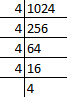4n = 45

Therefore, n = 5

5. How many terms of the 2/9 – 1/3 + ½ + … will make the sum 55/72?

Solution:-

From the question it is given that,

Terms of G.P. is 2/9 – 1/3 + ½ + …

Sum of the terms = 55/72

The first term a = 2/9

r = -1/3 ÷ 2/9 = (-1/3) × (9/2) = – 3/2

We know that, Sn = a(rn – 1)/r – 1 = 55/72

[(2/9)(1 – (-3/2)n)]/(1 + (3/2)) = 55/72

1 – (-3/2)n = (55/72) × (5/2) × (9/2)

(1 – (-1))n (3/2)n = 275/32

1 + 1(3/2)n = 275/32

(3/2)n = 275/32 – 1

(3/2)n = (275 – 32)/32

(3/2)n = 243/32

(3/2)n = (3/2)5

Therefore, n = 5

6. The 2nd and 5th terms of a geometric series are -½ and sum 1/16 respectively. Find the sum of the series up to 8 terms.

Solution:-

From the question it is given that,

a2 = -½

a5 = 1/16

We know that, a2 = arn – 1

= ar2 – 1

a2 = ar = -½ … [equation (i)]

a5 = ar5 – 1

= ar4

a5 = ar4 = 1/16 … [equation (ii)]

Now, dividing equation (ii) by (i) we get,

r3 = 1/16 ÷ (-½)

= (1/16) × (-2)

= -1/8

r3 = (-1/2)3

So, r = -½

ar = -½

a × (-½) = -½

a = – ½ × (-2/1)

a = 1

Therefore, a = 1 and r = -½

Then, S8 = a(1 – rn)/(1 – r)

= 1[1 – (-1/2)8]/(1 + ½)

= [1 – (1/256)]/(3/2)

= (255/256) × (2/3)

= (510/768)

= 85/128

7. The first term of G.P. is 27 and 8th term is 1/81. Find the sum of its first 10 terms.

Solution:-

From the question it is given that,

First term a = 27

8th term a8 = 1/81

Then, an = arn – 1

a8 = ar8 – 1 = 1/81

a8 = ar7 = 1/81

ar7 = 1/81

27r7 = 1/81

r7 = 1/(81 × 27)

r7 = 1/2187

r7 = 1/(37)

r = 1/3

So, S10 = a(1 – rn)/(1 – r)

= 27[1 – (1/3)10]/(1 – 1/3)

= 27[1 – (1/310)]/((3 – 1)/3)

= ((27 × 3)/2) [1 – 1/310]

= (81/2) [1 – 1/310]

8. Find the first term of the G.P. whose common ratio is 3, last term is 486 and the sum of whose terms is 728.

Solution:-

From the question it is given that,

Common ratio r = 3

Last term = 486

Sum of the terms = 728

We know that, Sn = a(rn – 1)/(r – 1)

= a(3n – 1)/(3 – 1) = 728

a(3n – 1)/2 = 728

a(3n – 1) = 728 × 2

a(3n – 1) = 1456 … [equation (i)]

Then, last term = arn – 1

486 = a × 3n – 1

486 = a(3n/3)

486 × 3 = a3n

1458 = a3n … [equation (ii)]

Consider equation (i), a(3n – 1) = 1456

a3n – a = 1456

Substitute the value of a3n in equation (i),

1458 – a = 1456

a = 1458 – 1456

a = 2

Therefore, the first term a is 2.

9. In a G.P. the first term is 7, the last term is 448, and the sum is 889. Find the common ratio.

Solution:-

From the question it is given that,

First term a is = 7

Then, last term is = 448

Sum = 889

We know that, last term = arn – 1

7rn -1 = 448

rn – 1 = 448/7

rn – 1 = 64 … [equation (i)]

So, sum = a(rn – 1)/(r – 1) = 889

7(rn – 1)/(r – 1) = 889

(rn – 1)/(r – 1) = 889/7

(rn – 1)/(r – 1) = 127 … [equation (ii)]

Consider the equation (i),

rn/r = 64

rn = 64r

Now substitute the value of rn in equation (ii),

(64r – 1)/(r – 1) = 127

64r – 1 = 127r – 127

127r – 64r = -1 + 127

63r = 126

r = 126/63

r = 2

Therefore, common ratio = 2

10. Find the third term of a G.P. whose common ratio is 3 and the sum of whose first seven terms is 2186.

Solution:-

From the question it is given that,

Common ratio r = 3

S7 = 2186

We know that, Sn = a(rn – 1)/(r – 1)

S7 = a(3n – 1)/(3 – 1)

2186 = a(3n – 1)/2

By cross multiplication,

(2186 × 2) = a(37 – 1)

(4372) = a(2187 – 1)

4372 = a2186

a = 4372/2186

a = 2

Then, a3 = ar3 – 1

= ar2

= 2 × 32

= 2 × 9

a3 = 18

11. If the first term of a G.P. is 5 and the sum of first three terms is 31/5, find the common ratio.

Solution:-

From the question it is given that,

First term of a G.P. is a = 5

The sum of first three terms is S3 = 31/5

We know that, Sn = a(rn – 1)/(r – 1)

S3 = a(r3 – 1)/(r – 1)

31/5 = 5(r3 – 1)/(r – 1)

31/(5 × 5) = (r3 – 1)/(r – 1)

31/25 = (r3 – 1)/(r – 1)

(r – 1) (r2 + r + 1)/(r – 1) = 31/25

r2 + r + 1 = 31/25

By cross multiplication we get,

25(r2 + r + 1) = 31

25r2 + 25r + 25 = 31

Transposing 31 from right hand side to left hand side it becomes – 31,

25r2 + 25r + 25 – 31 =

25r2 + 25r – 6 = 0

25r2 + 30r – 5r – 6 = 0

5r(5r + 6) – 1(5r + 6) = 0

(5r – 1) (5r + 6) = 0

Take 5r – 1 = 0

r = 1/5

or 5r + 6 = 0

r = -6/5

Therefore, common ratio r = 1/5 or -6/5.

Chapter – test

1. Write the first four terms of the A.P. when its first term is – 5 and the common difference is – 3.

Solution:-

From the question it is given that,

First term a = – 5

Common difference d = -3

Then the first four terms are = – 5 + (-3) = -5 – 3 = – 8

-8 + (-3) = – 8 – 3 = -11

– 11 + (-3) = – 11 – 3 = – 14

Therefore, first four terms are -5, -8, -11 and -14.

2. Verify that each of the following lists of numbers is an A.P., and the write its next three terms:

(i) 0, ¼, ½, ¾, …

Solution:-

From the question it is given that,

First term a = 0

Common difference = ¼ – 0 = ¼

So, next three numbers are ¾ + ¼ = 4/4 = 1

1 + ¼ = (4 + 1)/4 = 5/4

5/4 + ¼ = 6/4 = 3/2

Therefore, the next three term are 1, 5/4 and 3/2.

(ii) 5, 14/3, 13/3, 4, …

Solution:-

From the question it is given that,

First term a = 5

Common difference = 14/3 – 5 = (14 – 15)/3 = -1/3

So, next three numbers are 4 + (-1/3) = (12 – 1)/3 = 11/3

11/3 + (-1/3) = (11 – 1)/3 = 10/3

10/3 + (-1/3) = (10 – 1)/3 = 9/3 = 3

Therefore, the next three term are 11/3, 10/3 and 3.

3. The nth term of an A.P. is 6n + 2. Find the common difference.

Solution:-

From the question it is given that,

nth term is 6n + 2

So, Tn = 6n + 2

Now, we start giving values, 1, 2, 3, … in the place of n, we get,

T1 = (6 × 1) + 2 = 6 + 2 = 8

T2 = (6 × 2) + 2 = 12 + 2 = 14

T3 = (6 × 3) + 2 = 18 + 2 = 20

T4 = (6 × 4) + 2 = 24 + 2 = 26

Therefore, A.P. is 8, 14, 20, 26, …

So, common difference d = 14 – 8 = 6

4. Show that the list of numbers 9, 12, 15, 18, … form an A.P. Find its 16th term and the nth.

Solution:-

From the question,

The first term a = 9

Then, difference d = 12 – 9 = 3

15 – 12 = 3

18 – 15 = 3

Therefore, common difference d = 3

From the formula, an = a + (n – 1)d

Tn = a + (n – 1)d

= 9 + (n – 1)3

= 9 + 3n – 3

= 6 + 3n

So, T16 = a + (n – 1)d

= 9 + (16 – 1)3

= 9 + (15)(3)

= 9 + 45

= 54

5. Find the 6th term from the end of the A.P. 17, 14, 11, …, – 40.

Solution:-

From the question it is given that,

First term a = 17

Common difference = 14 – 17 = – 3

Last term l = – 40

L = a + (n – 1)d

-40 = 17 + (n – 1)(-3)

-40 – 17 = -3n + 3

– 57 – 3 = -3n

n = -60/-3

n = 20

Therefore, 6th term form the end = l – (n – 1)d

= – 40 – (6 – 1)(-3)

= – 40 – (5)(-3)

= – 40 + 15

= – 25

6. If the 8th term of an A.P. is 31 and the 15th term is 16 more than its 11th term, then find the A.P.

Solution:-

From the question it is given that,

a8 = 31

a15 = the 15th term is 16 more than its 11th term = a11 + 16

we know that, an = a + (n – 1)d

So, a8 = a + 7d = 31 … [equation (i)]

a15 = a + 14d = a + 10d + 16

14d – 10d = 16

4d = 16

d = 16/4

d = 4

Now substitute the value of d in equation (i) we get,

a + (7 × 4) = 31

a + 28 = 31

a = 31 – 28

a = 3

So, 3 + 4 = 7, 7 + 4 = 11, 11 + 4 = 15

Therefore, A.P. is 3, 7, 11, 15, …

7. The 17th term of an A.P. is 5 more than twice its 8th term. If the 11th term of the A.P. is 43, then find the wth term.

Solution:-

From the question it is given that,

a17 = 5 more than twice its 8th term = 2a8 + 5

a11 = 43

an = ?

We know that, a11 = a + 10d = 43 … [equation (i)]

a17 = 2a8 + 5

a + 16d = 2(a + 7d) + 5

a + 16d = 2a + 14d + 5

2a – a = 16d – 14d – 5

a = 2d – 5 … [equation (ii)]

Now substitute the value of a in equation (i) we get,

2d – 5 + 10d = 43

12d = 43 + 5

12d = 48

d = 48/12

d = 4

To find out the value of a substitute the value of d in equation (i)

a + (10 × 4) = 43

a + 40 = 43

a = 43 – 40

a = 3

Then, an = a + (n – 1)d

= 3 + 4(n – 1)

= 3 + 4n – 4

= 4n – 1

8. The 19th term of an A.P. is equal to three times its 6th term. If its 9th term is 19, find the A.P.

Solution:-

From the question it is given that,

a19 = 19th term of an A.P. is equal to three times its 6th term = 3a6

a9 = 19

As we know, an = a + (n – 1)d

a9 = a + 8d = 19 … [equation (i)]

Then, a19 = 3(a + 5d)

a + 18d = 3a + 15d

3a – a = 18d – 15d

2a = 3d

a = (3/2)d

Now substitute the value of a in equation (i) we get,

(3/2)d + 8d = 19

(3d + 16d)/2 = 19

(19/2)d = 19

d = (19 × 2)/19

d = 2

To find out the value of a substitute the value of d in equation (i)

a + 8d = 19

a + (8 × 2) = 19

a + 16 = 19

a = 19 – 16

a = 3

Therefore, A.P. is 3, 5, 7, 9, …

9. If the 3rd and the 9th terms of an A.P. are 4 and – 8 respectively, then which term of this A.P. is zero?

Solution:-

From the question it is given that,

a3 = 4

a9 = – 8

We know that, a3 = a + 2d = 4 … [equation (i)]

a9 = a + 8d = -8 … [equation (ii)]

Now, subtracting equation (i) from equation (ii)

(a + 8d) – (a + 2d) = -8 – 4

a + 8d – a – 2d = -12

6d = -12

d = -12/6

d = -2

To find out the value of a substitute the value of d in equation (i)

a + 2d = 4

a + (2 × (-2)) = 4

a – 4 = 4

a = 4 + 4

a = 8

let us assume nth term be zero, then

a + (n – 1)d = 0

8 + (n – 1)(-2) = 0

-2n + 2 = -8

-2n = -8 – 2

-2n = -10

n = -10/-2

n = 5

Therefore, 0 will be the fifth term.

10. Which term of the list of numbers 5, 2, – 1, – 4, … is – 55?

Solution:-

From the question it is given that,

First term a = 5

nth term = -55

Common difference d = 2 – 5 = – 3

We know that, an = a + (n – 1)d

– 55 = 5 + (n – 1)(-3)

-55 – 5 = – 3n + 3

-60 – 3 = -3n

-63 = -3n

n = -63/-3

n = 21

Therefore, -55 is the 21st term.

11. The 24th term of an A.P. is twice its 10th term. Show that its 72nd term is four times its 15th term.

Solution:-

From the question it is given that,

The 24th term of an A.P. is twice its 10th term = a24 = 2a10

We have to show that, 72nd term is four times its 15th term = a72 = 4a15

We know that, a24 = a + 23d = 2a10

a + 23d = 2(a + 9d)

a + 23d = 2a + 18d

2a – a = 23d – 18d

a = 5d … [equation (i)]

a72 = 4a15

a + 71d = 4(a + 14d)

Substitute the value of a we get,

5d + 71d = 4(5d + 14d)

76d = 4(19d)

Therefore, it is proved that 72nd term is four times its 15th term.

12. Which term of the list of numbers 20, 19¼, 18½, 17¾, … is the first negative term?

Solution:-

From the question it is given that,

First term a = 20

Common difference d = 19¼ – 20 = 77/4 – 20 = (77 – 80)/4 = -¾

We know that, an = a + (n – 1)d

an = 20 + (n – 1) (-¾)

an = 20 – ¾n + ¾

an = 20 + ¾ – ¾n

an = (80 + 3)/4 – ¾n

an = 83/4 – ¾n < 0

83/4 < ¾n

83 < 3n

83/3 < n

28 < n

Therefore, 28th is the first negative term.

13. How many three digit numbers are divisible by 9?

Solution:-

The three digits numbers which are divisible by 9 are 108, 117, 126, …, 999

Then, first term a = 108

Common difference = 9

Last term = 999

We know that, l = an = a + (n – 1)d

999 = 108 + (n – 1)9

999 – 108 = 9n – 9

891 + 9 = 9n

900 = 9n

n = 900/9

n = 100

Therefore, there are 100 three digits numbers.

14. The sum of three numbers in A.P. is – 3 and the product is 8. Find the numbers.

Solution:-

From the question it is given that,

The sum of three numbers in A.P. = – 3

The product of three numbers in A.P. = 8

Let us assume the 3 numbers which are in A.P. are, a – d, a, a + d

Now adding 3 numbers = a – d + a + a + d = – 3

3a = -3

a = -3/3

a = -1

From the question, product of 3 numbers is – 35

So, (a – d) × (a) × (a + d) = 8

a(a2 – d2) = 8

-1 ((-1)2 – d2 = 8

1 – d2 = 8/-1

1 – d2 = -8

d2 = 8 + 1

d2= 9

d = √9

d = ±3

Therefore, the numbers are if d = 3 (a – d) = – 1 – 3 = – 4

a = -1

(a + d) = – 1 + 3 = 2

If d = – 6

The numbers are (a – d) = – 1 – (-3) = – 1 + 3 = 2

a = -1

(a + d) = -1 + (-3) = -1 – 3 = -4

Therefore, the numbers -4, -1, 2,… and 2, -1, -4,… are in A.P.

15. The angles of a quadrilateral are in A.P. If the greatest angle is double of the smallest angle, find all the four angles.

Solution:-

From the question it is given that,

The angles of a quadrilateral are in A.P.

Greatest angle is double of the smallest angle

Let us assume the greatest angle of the quadrilateral is a + 3d,

Then, the other angles are a + d, a – d, a – 3d

So, a – 3d is the smallest

Therefore, a + 3d = 2(a – 3d)

a + 3d = 2a – 6d

6d + 3d = 2a – a

9d = a … [equation (i)]

We know that the sum of all angles of quadrilateral is 360o.

a – 3d + a – d + a + d + a+ 3d = 360o

4a = 360o

a = 360/4

a = 90o

Now, substitute the value of a in equation (i) we get,

9d = 90

d = 90/9

d = 10

Substitute the value of a and d in assumed angles,

Greatest angle = a + 3d = 90 + (3 × 10) = 90 + 30 = 120o

Then, other angles are = a + d = 90o + 10o = 100o

a – d = 90o – 10o = 80o

a – 3d = 90o – (3 × 10) = 90 – 30 = 60o

Therefore, the angles of quadrilateral are 120o, 100o, 80o and 60o.

16. Find the sum of first 20 terms of an A.P. whose nth term is 15 – 4n.

Solution:-

From the question it is given that,

nth term is 15 – 4n

So, an = 15 – 4n

Now, we start giving values, 1, 2, 3, … in the place of n, we get,

a1 = 15 – (4 × 1) = 15 – 4 = 11

a2 = 15 – (4 × 2) = 15 – 8 = 7

a3 = 15 – (4 × 3) = 15 – 12 = 3

a4 = 15 – (4 × 4) = 15 – 16 = – 1

Then, a20 = 15 – (4 × 20) = 15 – 80 = -65

So, 11, 7, 3, -1, … -65 are in A.P.

Therefore, first term a = 11

Common difference = -4

n = 20

S20 = (n/2) [2a + (n – 1)d]

= (20/2) [(2 × 11) + (20 – 1)(-4)]

= 10 [22 – (19) (-4)]

= 10 [22 – 76]

= 10(-54)

= -540

Therefore, the sum of first 20 terms of an A.P. is -540.

17. Find the sum : 18 + 15½ + 13 + … + (-49½)

Solution:-

From the question it is given that,

First term a = 18

Common difference d = 15½ – 18

= 31/2 – 18

= (31 – 36)/2

= -5/2

Last term = -49½ = -99/2

We know that, an = a + (n – 1)d

-99/2 = 18 + (n – 1)(-5/2)

(-99/2) – (18/1) = (n – 1)(-5/2)

(-99 – 36)/2 = (-5/2)(n – 1)

(-135/2) = (-5/2) (n – 1)

(-135/2) × (-2/5) = n – 1

-135/-5 = n – 1

27 = n – 1

n = 27 + 1

n = 28

Then, Sn = (n/2) [2a + (n – 1)d]

S28 = (28/2) [(2 × 18) + (28 – 1)(-5/2)]

S28 = 14[36 + (27 × (-5/2))]

S28 = 14[36 – (135/2)]

S28 = 14 [(72 – 135)/2]

S28 = 14 (-63/2)

S28 = – 441

18. (i) How many terms of the A.P. – 6, (-11/2), – 5, … make the sum – 25?

Solution:-

From the question it is given that,

Terms of the A.P. is -6, (-11/2) – 5, …

The first term a = -6

Common difference d = (-11/2) – (-6)

= (-11/2) + 6

= (-11 + 12)/2

= ½

The terms are make the sum – 25

Then, Sn = (n/2)(2a + (n – 1)d)

-25 = (n/2) [(2 × (-6)) + (n – 1) (½)]

(-25 × 2) = n [-12 + ½n – ½]

-50 = n [(-25/2) + (½n)]

½n2 – (25/2)n + 50 = 0

n2 – 25n + 100 = 0

n2 – 5n – 20n + 100 = 0

n(n – 5) – 20(n – 5) = 0

(n – 5) (n – 20) = 0

So, n – 5 = 0

n = 5

or n – 20 = 0

n = 20

Therefore, number of terms are 5 or 20.

(ii) Solve the equation 2 + 5 + 8 + … + x = 155

Solution:-

From the question it is given that,

First term a = 2

Last term = x

Common difference d = 5 – 2 = 3

Then, sum of the terms = 155

L = a + (n – 1)d

x = 2 + (n – 1)3

x = 2 + 3n – 3

x = 3n – 1 … [equation (i)]

We know that, Sn = (n/2) [2a + (n – 1)d]

155 = (n/2) [(2 × 2) + (n – 1) × 3]

155 × 2 = n[4 + 3n – 3]

310 = n(3n + 1)

310 = 3n2 + n

3n2 + n – 310 = 0

3n2 – 30n + 31n – 310 = 0

3n(n – 10) + 31(n – 10) = 0

(n – 10) (3n + 31) = 0

So, n – 10 = 0

n = 10

or 3n + 31 = 0

n = -31/3

negative is not possible.

Therefore, n = 10

Now, substitute the value of n in equation (i),

x = 3n – 1

= (3 × 10) – 1

= 30 – 1

= 29

19. If the third term of an A.P. is 5 and the ratio of its 6th term to the 10th term is 7 : 13, then find the sum of first 20 terms of this A.P.

Solution:-

From the question it is given that,

The third term of an A.P. a3 = 5

The ratio of its 6th term to the 10th term a6 : a10 = 7 : 13

We know that, an = a + (n – 1)d

a3 = a + (3 – 1)d = 5

= a + 2d = 5 … [equation (i)]

Then, a6/a10 = 7/13

(a + 5d)/(a + 9d) = 7/13

By cross multiplication we get,

13(a + 5d) = 7(a + 9d)

13a + 75d = 7a + 63d

13a – 7a + 65d – 63d = 0

6a + 2d = 0

Divide by 2 on both side we get,

3a + d = 0

d = -3a … [equation (ii)]

Substitute the value of d in equation (i),

a + 2(-3a) = 5

a – 6a = 5

-5a = 5

a = -5/5

a = -1

Now substitute the value of a in equation (ii),

d = -3(-1)

d = 3

Then, sum of first 20 terms,

= (n/2) [2a + (n – 1)d]

= (20/2)[(2 × (-3)) + (2- – 1)3]

= 10[-2 + 57]

= 10 × 55

= 550

20. The sum of first 14 terms of an A.P. is 1505 and its first term is 10. Find its 25th term.

Solution:-

From the question it is given that,

First term a = 10

The sum of first 14 terms of an A.P. = 1505

25th term = ?

We know that, Sn = (n/2) [2a + (n – 1)d]

S14 = (n/2) [2a + (n – 1)d]

1505 = (14/2) [(2 × 10) + (14 – 1)d]

1505 = 7[20 + 13d]

1505/7 = 20 + 13d

215 = 20 + 13d

13d = 215 – 20

13d = 195

d = 195/13

d = 15

Then, an = a + (n – 1)d

a25 = 10 + (25 – 1)(15)

= 10 + (24)15

= 10 + 360

= 370

21. Find the geometric progression whose 4th term is 54 and 7th term is 1458.

Solution:-

From the question it is given that,

The geometric progression whose 4th term a4 = 54

The geometric progression whose 7th term a7 = 1458

We know that, an = arn – 1

a4 = ar4 – 1

a4 = ar3 = 54

a7 = ar6 = 1458

By dividing both we get,

ar6/ar3 = 1458/54

r6 – 3 = 27

r3 = 33

r = 3

To find out a, consider ar3 = 54

a(3)3 = 54

a = 54/27

a = 2

Therefore, a = 2, r = 3

So, G.P. is 2, 6, 18, 54,…

22. The fourth term of a G.P. is the square of its second term and the first term is – 3. Find its 7th term.

Solution:-

From the question it is given that,

The fourth term of a G.P. is the square of its second term = a4 = (a2)2

The first term a1 = – 3

We know that, an = arn – 1

a4 = ar4 – 1

a4 = ar3

a2 = ar

Now, ar3 = (ar)2

ar3 = a2r2

r3/r2 = a2/a

r3 – 2 = a2 – 1 … [from am/an = am – n]

r = a

a1 = -3

a7 = ar7 – 1

a7 = ar6

= -3 × (-3)6

= -3 × 729

= -2187

Therefore, the 7th term a7 = -2187

23. If the 4th, 10th and 16th terms of a G.P. are x, y and z respectively, prove that x, y and z are in G.P.

From the question it is given that,

a4 = x

a10 = y

a16 = z

Now, we have to show that x, y and z are in G.P.

We know that,

an = arn – 1

a4 = ar4 – 1

a4 = ar3 = x

a10 = ar9 = y

a16 = ar15 = z

x, y, z are in G.P.

If y2 = xy

Substitute the value of x and y,

y2 = (ar9)2

y2 = a2r18

Then, xz = ar3 × ar15

= a1 + 1 r3 + 15 … [from am × an = am + n]

= a2r18

So, y2 = xy

Therefore, it is proved that x, y, z are in G.P.

24. How many terms of the G.P. 3, 3/2, ¾ are needed to give the sum 3069/512?

Solution:-

From the question it is given that,

Sum of the terms Sn = 3069/512

First term a = 3

Common ratio r = (3/2)/3

= (3/2) × (1/3)

= ½

We know that, Sn = a(1 – rn)/(1 – r)

(3069/512) = 3[1 – (½)n]/ (1 – ½)

(3069/512) = (2 × 3) [1 – (½)n]

1 – (½)n = 3069/(512 × 6)

1 – (½)n = 1023/1024

(½)n = 1 – (1023/1024)

(½)n = (1024 – 1023)/1024

(½)n = 1/1024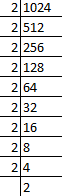(½)n = (½)10

By comparing both LHS and RHS,

n = 10

Therefore, there are 10 terms are in the G.P.